# “High-Res” Audio: Part 8b: Filter Resolution

In the previous posting, we left off with this drawing of a biquad filter: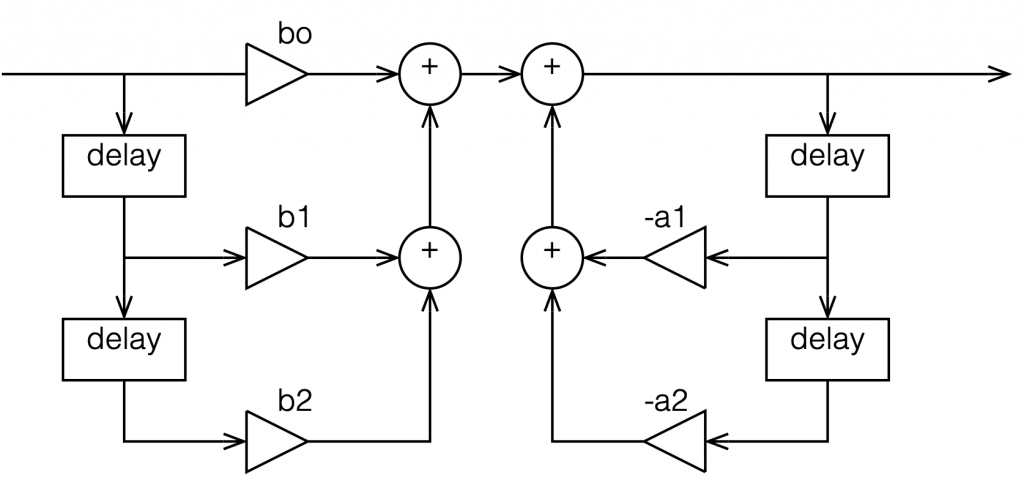Figure 1: A biquad broken into an FIR filter with two 1-sample long delays and 3 gains combined with an IIR filter with two delays and 2 gains.

This is not the normal way to draw the signal flow inside a biquad, since it has a little too much information. Normally you see something like this:

or this:

In the versions I show above, the feed-forward half of the biquad comes first, and its output feeds the start of the feedback portion. It is also possible to reverse these, putting the feedback portion first, like this:

In theory, these different implementations will all result in the same output if you match the gain values. However, in practice, they are not the same, and this difference is where we need to look for this part of the discussion on high res audio.

Let’s say I want to make a simple filter that reduces bass in a fairly narrow frequency band. I can use a biquad to do this. For example, if I want a peaking filter that reduces 20 Hz by 12 dB, with a Q of 1, then I get a magnitude response that looks like this: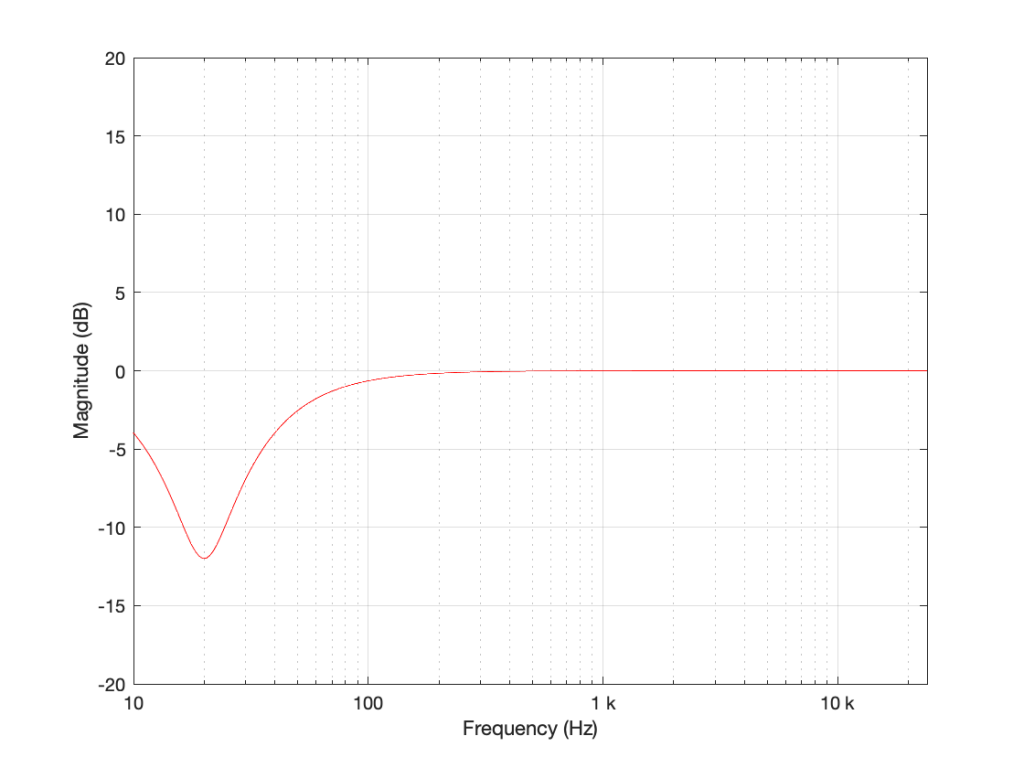Figure 5: The magnitude response of a peaking filter where F = 20 Hz, G = -12 dB, Q = 1

If I wanted to build this filter using a biquad in a system with a sampling rate of 48 kHz, it would have the following gain coefficients:

b0 = 0.998049357193933
b1 = -1.994783192754608
b2 = 0.996740671594426
a1 = -1.994783192754608
a2 = 0.994790028788359

We’ll also say that my biquad is implemented like the one shown in Figure 1, above… let’s take a look at that signal flow again: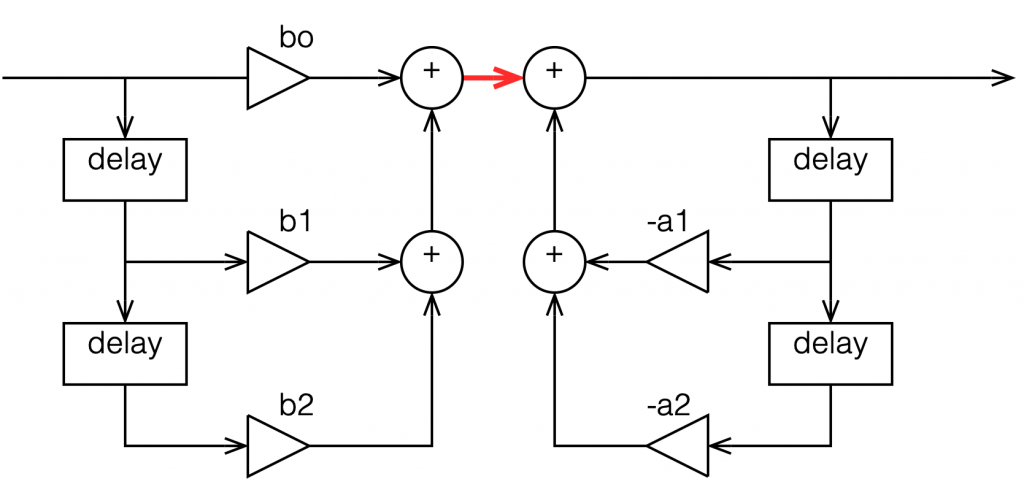Figure 6: A copy of Figure 1 with one interior point in the signal flow highlighted in red.

I’ve highlighted a point inside the biquad using a red arrow. Let’s talk about the signal right there, in the middle of the processing…

In the last post, we talked about how, when the signal frequency is very low, a single sample delay has almost the same value at its output as its input, because the phase difference is so small for such a small time. So, let’s start with the (incorrect) assumption that, for those two feed-forward delays at the beginning, their outputs ARE equal to their inputs (because we’re starting with a low frequency). What happens when the input has a value of 1? Then the value at the red arrow is just the sum of the feed forward gains (because I multiplied each of them by 1 and added them together…)

In the case of the filter I described above, this value will be 0.000006836, which is a very small number. Also, if the value coming into the input of the biquad is less than 1, the value at the red arrow will be even smaller! This means that, if you come into the biquad with a low-frequency tone with a level of 0 dB FS, the level at that red arrow will be about -103 dB FS, which is very quiet. The feed-back portion of the biquad, after the red arrow, then has a lot of gain in it to bring the signal level back up towards 0 dB FS again.

So, the issue that we have here is that the FF (Feed Forward) portion of the biquad drops the level A LOT. And the FB portion increases the level A LOT, just to do something like a little 12 dB dip at 20 Hz.

The magnitude of the gains downwards and upwards in those two portions of the biquad are dependent on the parameters of the filter that we’re trying to make, however, we can generalise a little and say that:

• the lower the frequency
OR
• the higher the Q,
• then the bigger the gain down and up.

In other words, if you have a really low frequency dip, with a really high Q, then the level of the signal at that red arrow will be really low. REALLY low.

## How low can you go?

How low is “REALLY low”? let’s see: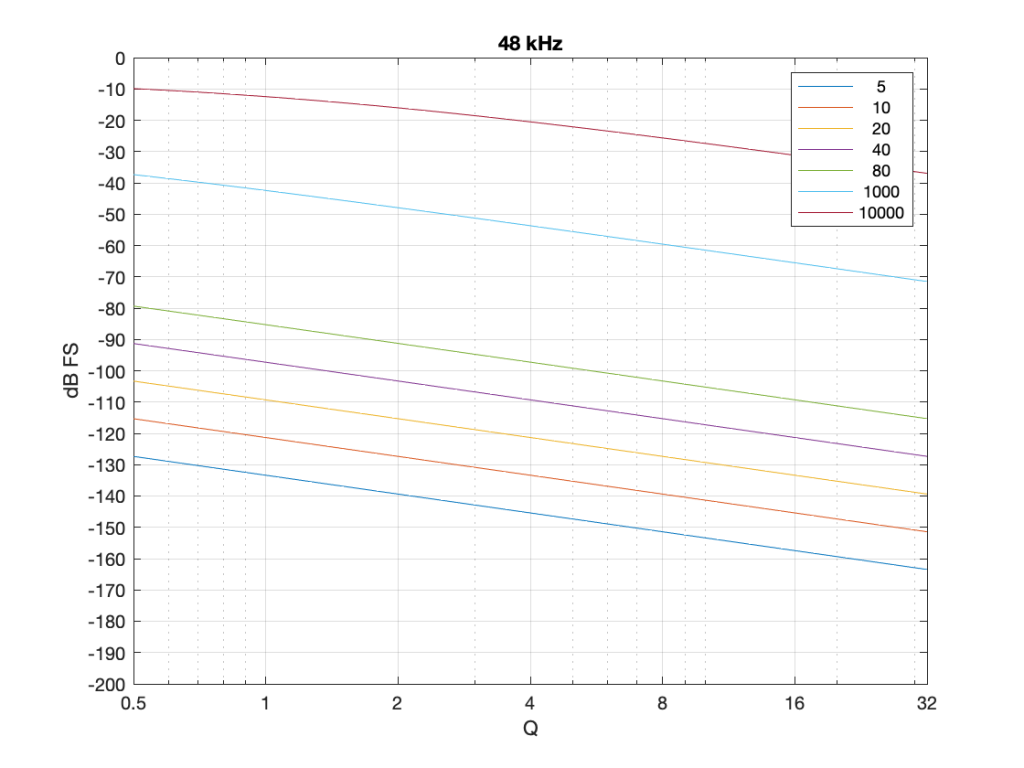Figure 7: The level of a 0 dB FS signal at various frequencies listed in the top right corner, measured inside the biquad shown in Figure 6 at the red arrow, for a peaking filter with a gain of -12 dB, and with variable Q and Fc, in a system running at 48 kHz.

Take a look at Figure 7, which shows some values for one example filter (peaking, Gain = -12 dB, variable Q and Fc, and the test frequency = Fc). Notice that when the Fc is 10 kHz, even at earn Q=32, the signal level at the middle of the biquad is about -38 dB FS or so. However, when the Fc is 20 Hz, it’s -140 dB FS… This is very low.

Now let’s try again at a higher sampling rate: 192 kHz.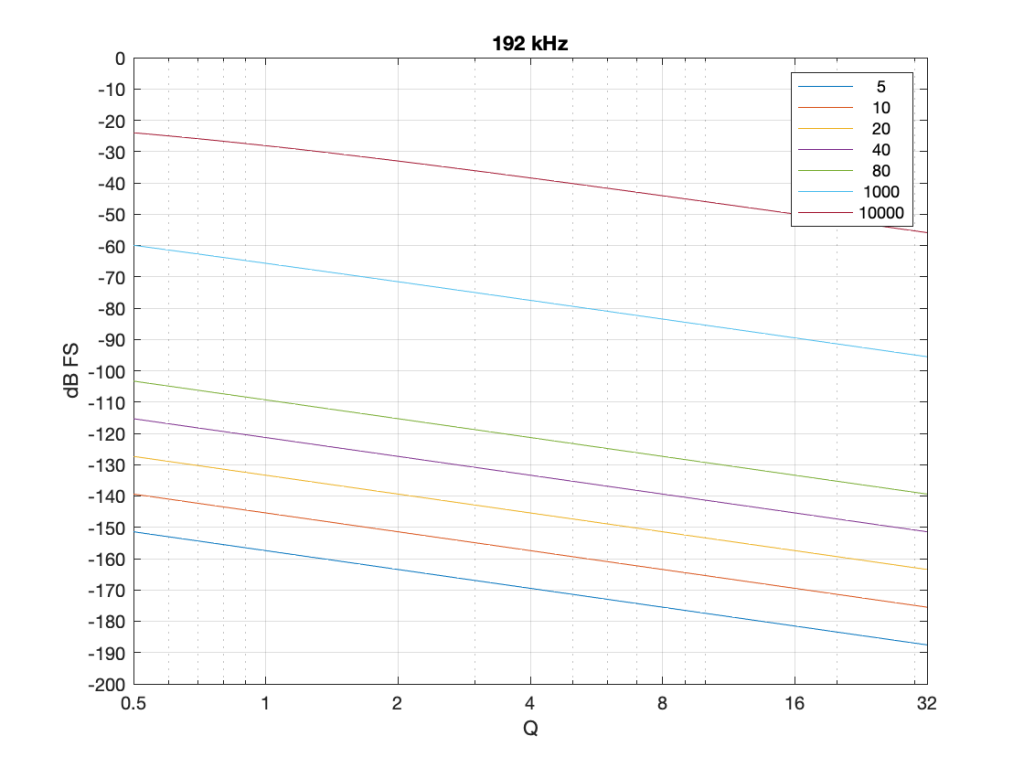Figure 8: Identical parameters to that shown in Figure 7, but in a system running at 192 kHz.

Notice that when we do exactly the same thing running at 192 kHz, the signal levels inside the biquad get much lower. Now for a 20 Hz signal and a Q of 32, the level is around -163 dB FS – a drop of more than 20 dB for 4x the sampling rate.

Why does this happen? It’s because the filter doesn’t “know” that the signal is at 20 Hz. It only knows the relationship between the frequency and the sampling rate. So, in its little world, 20 Hz doesn’t exist. In a system running at 48 kHz, what exists is 20 / 48000 = 0.0004167. This is called the “normalised frequency” where the sampling rate is 1, DC is 0, and everything else is in between. (Note that some textbooks and software say that Nyquist = 1 instead of the sampling rate – but you just need to know what the convention is for the thing you’re reading…) This means that if the sampling rate goes up to 192 kHz, then the normalised frequency for 20 Hz is 20 / 192000 = 0.0001042 (1/4 of the value because the sampling rate was multiplied by 4).

## So what?

This is important. If you want to make a low-frequency, high-Q peaking filter in a digital system with a cut of 12 dB, you are forcing the signal to a very low level inside your filter, and then bringing it back up to a normal level again on the way out. If your processing is running with a limited resolution, (e.g. 16-bits, for example) then the signal level can approach or even go below the resolution of your system inside the biquad. This means that, when the signal’s level is raised again on the way out, it’s full of quantisation distortion, and you can’t get rid of it… This is bad.

There are different ways to solve this problem.

• Increase the resolution of your processing internally. For example, even though your input and output might only be running at 16-bits or 24-bits, maybe you need more resolution inside to make the results of the math better – or at least below the limitations of the input and output.
• Change the way the biquad is implemented. For example, if you use the implementation shown in Figure 4 (with the feedback before the feed-forward) instead of the one we used, then you don’t drop the signal level and raise it again, you do the opposite. This avoids your quantisation error problem. However, depending on the system, it might overload and clip the signal inside the biquad instead, so then you just end up with a different kind of distortion instead.
• Reduce your sampling rate to make it closer to your filter’s frequency. The problem I showed above is that the centre frequency of the filter is too far away from the sampling rate. If the sampling rate were lower, then this automatically makes the filter’s centre frequency “higher” in a normalised frequency scale, thus reducing the problem.
• Other, even more clever solutions that I won’t talk about because they’re not as simple.

This means (for example) that if you’re building a subwoofer with digital filtering, and you know for sure that NOTHING will come out of it above, say 1 kHz (just to pick a random number that’s far enough away from the typical 120 Hz that people normally use…) then it would be dumb to do the filtering at 192 kHz. It’s smarter to run its internal sampling rate at 2 kHz (because we only need to go up to 1 kHz; and we’re not considering anything other issues or artefacts in this posting.)

## P.S.

For this discussion, I used the specific example of a peaking filter with a gain of -12 dB, and I was varying the Q and the Fc. I was also measuring the level of the signal using a sine wave with a frequency that was the same as Fc in each case. However, the general lesson here about low frequency and high-Q filtering holds for other filter types and implementations as well.

# “High-Res” Audio: Part 8a: Filter resolution (The Setup…)

Almost every audio system has filters or equalisers in it for some reason or another. Originally, equalisers were named that because they were put in on long-distance telephone lines to make the balance of the frequency content more equal. Nowadays, we use equalisers to do things like add bass, or to add more bass.

In the “old days” audio filters were made by building circuits with resistors, capacitors, and inductors: if you choose the relationships between the values of these devices correctly, you can affect the magnitude response as you choose. The problem was production tolerances: if you take two resistors out of the package, and both are supposed to have the same resistance – they’ll be close, but they won’t be identical.

One of the great things about audio filters implemented in a digital system is that you don’t need to worry about variations as a result of production differences. Since digital filters are “just math”, you put the same equation in every device, and you get the same answer for the same input every time. (In the same way that, if I have two calculators on my desk, and I put “2 x 3″ into both of them, and press”=”, I’ll get the same answer on both devices.)

So, to start, let’s talk a little about how a digital filter works. Generally speaking, digital filters work by taking an audio signal, delaying it, changing the level, and adding the result back to the signal itself. Let’s take a simple example, shown in Figure 1.

Let’s say that, to start, we make the gain in that signal flow = 1, and set the delay to equal 1 sample. In this case:

• At a very low frequency, the output of the delay has almost exactly the same value as its input (because 1 sample is a phase difference of almost 0º at a low frequency). When you add a signal to (nearly) itself, you get twice the output – a gain of 6 dB.
• As the frequency of the input goes higher and higher, the delay (of 1 sample) is more and more significant, and therefore its output value gets more and more different from its input value.
• When the input signal’s frequency is 1/2 of the sampling rate, then a delay of 1 sample is equal to a 90º phase shift. When you add a sine wave to itself with a “delay” (actually a “phase shift”) of 90º, the result is a magnitude that is 3 dB higher than the original.
• When the input signal’s frequency is 1/3 of the sampling rate, then a delay of 1 sample is equal to a 120º phase shift. When you add a sine wave to itself with a “delay” (actually a “phase shift”) of 120º, there’s no change in the magnitude (the level).
• When the frequency is 1/2 the sampling rate, then each consecutive sample is 180º out of phase with the previous one, so the sum of the delay and the signal results in complete cancellation, and you get no output at all.

An example of the magnitude response plot of this is shown below in Figure 2.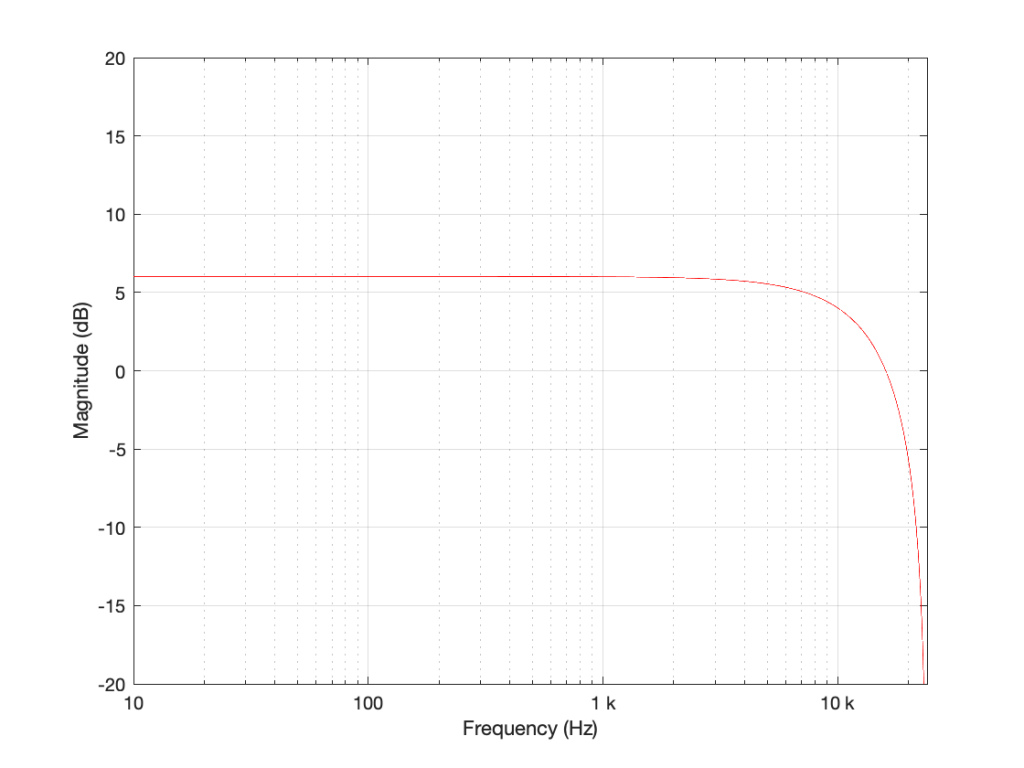Figure 2: The magnitude response of the filter shown in Figure 1 when the delay is set to 1 sample, the gain is set to 1 and Fs=48 kHz.

If we reduce the gain to, say 0.5, then the effect of adding the delayed signal is reduced. The overall shape of the magnitude response is the same, it’s just less, as shown in Figure 3.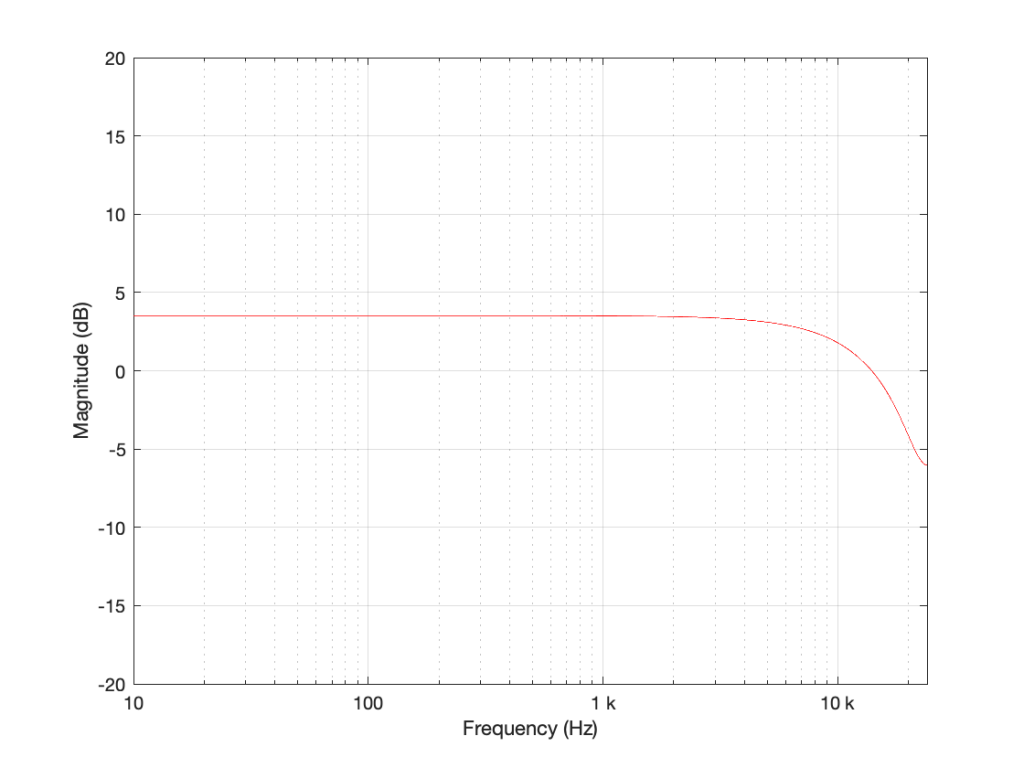Figure 3: The magnitude response of the filter shown in Figure 1 when the delay is set to 1 sample, the gain is set to 0.5 and Fs=48 kHz.

Notice in Figure 3 that the boost in the low end is less, and the dip in the high end is also less than in Figure 2. So, by adjusting the gain on the delayed signal that’s added to the original signal, we can adjust how much this filter is affecting the signal.

What happens when we change the delay? If we make it 2 samples instead of 1, then the phase difference between the output and the input of the delay will be bigger for a given frequency. This also means that the delay will be equivalent to a 180º phase shift at 1/4 Fs (instead of 1/2). Also, at 1/2 Fs, the delay will be equivalent to a 360º phase shift, so the signal adds constructively, just like it does in the low frequencies. So, the resulting magnitude response will look like Figure 4.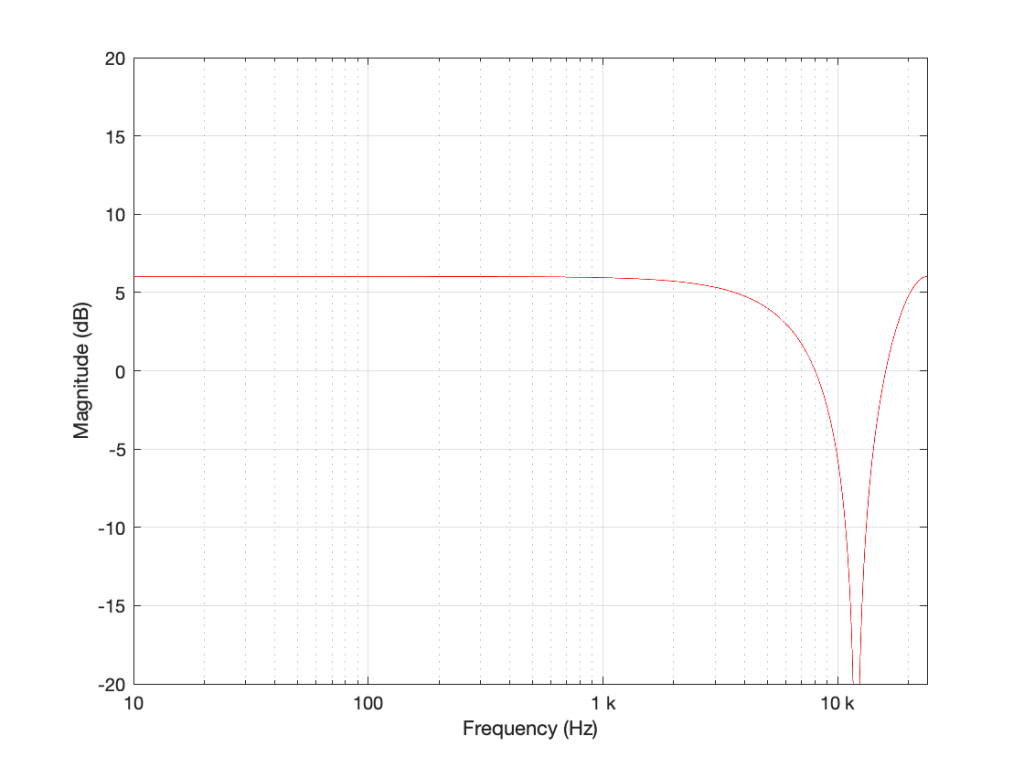Figure 4: The magnitude response of the filter shown in Figure 1 when the delay is set to 2 samples, the gain is set to 1 and Fs=48 kHz.

Again, if we reduce the gain, we reduce the effect of the filter, as can be seen in Figure 5.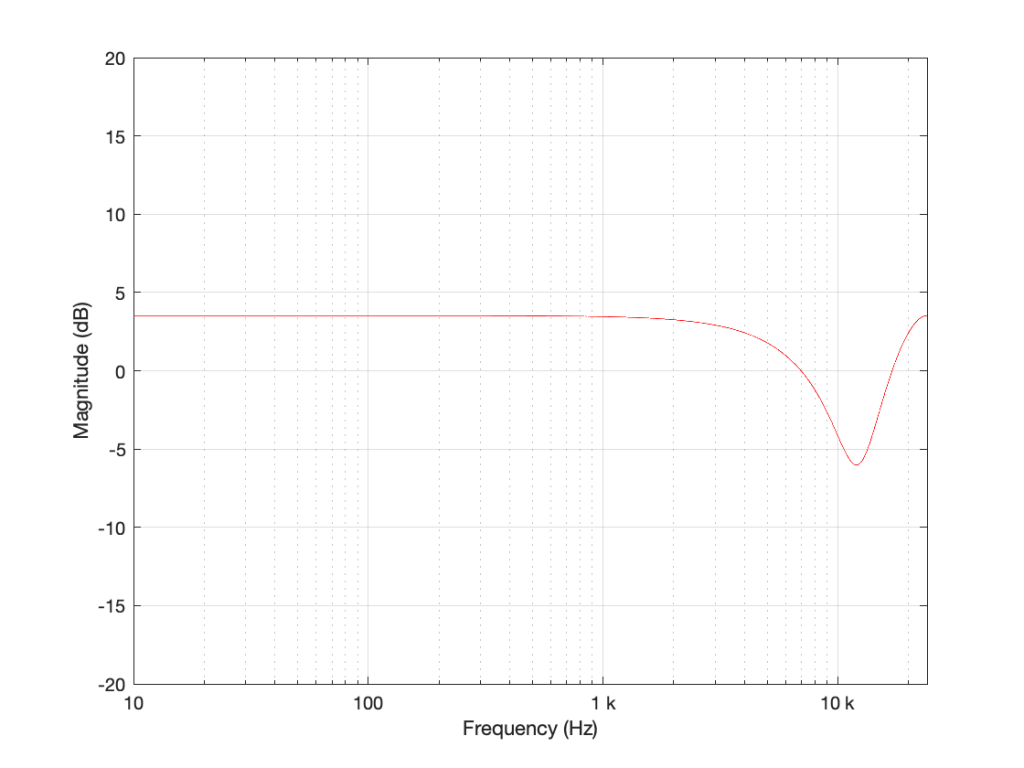Figure 5: The magnitude response of the filter shown in Figure 1 when the delay is set to 2 samples, the gain is set to 0.5 and Fs=48 kHz.

Now let’s make things a little more complicated. We can add another delay and another gain to get a little more control of things.

I’m not going to get very detailed about this – but if each of those delays is just one sample long, and we only play with the gains g1 and g2, we can start getting some nice control over the response of this filter. Below are some examples of the results we can get with just this filter, playing with the gains.

So, as you can see, all I need to do is to play with those two gains to get some nice control over the magnitude response.

Up to now, everything I’ve done is to add a delayed copy of the input to itself. This is what is known as a “feed-forward” design because (as you can see in Figures 1 and 6) I’m feeding the signal forwards in the flow to be added to itself. However, if there’s a “feed-forward”, it must be because we want to distinguish it from a “feed-back” design.

This is a filter where we delay the output (instead of the input) multiply that by a gain, and add it to the signal, as shown in Figure 11.

This feedback means that, if the gain is not equal to zero, once a signal gets into the input, the output will last forever. This is why this kind of filter is called an infinite impulse response filter (or IIR filter): because if an impulse (a short spike) gets into it, there will be a signal at the output until the end of time (theoretically, at least…).

And, yes… the output of a filter without a feedback loop will eventually stop, which means it’s a finite impulse response filter (or FIR). Stop the input signal, wait for the last delay to send its signal through, and the output stops.

## So what?

Most filters in most digital audio devices are built on a combination of these two types of designs. If you take Figure 6 and you combine it with an extended version of Figure 11 (with two delays instead of just one) you get Figure 12:Figure 12: An FIR filter with two delays and 3 gains combined with an IIR filter with two delays and 2 gains.

This combination of FIR and IIR filters is a powerful little tool that forms the heart of almost every digital audio filter in the world. (yes, there are exceptions, but they’re definitely exceptions…). There are different ways to implement it (for example, you could put the IIR before the FIR, or you could re-draw it to share the delays) but the result is the same.

This little tool is what we call a “biquadratic filter” or “biquad” for short. (The reason it’s called that is that the effect it has on the signal (its “transfer function”) can be mathematically expressed as the ratio of two quadratic equations – but I will not say anything more about that.) Whenever developers are building a new digital audio device like a loudspeaker or a pair of headphones or a car audio system, it’s common in the early meetings to hear someone ask “how many biquads will we need?” which is a way of asking “how much processing power and memory will we need?” (In the same way that I can measure prices in pizzas, or when I was a kid I would ask “how many more Sesame Streets until we’re there?”)

At this point, you may be asking why I’ve gone through all of this, since I haven’t said anything about high resolution audio. The reason is that, in the next posting, we’ll look at what’s going on inside that biquad when you use it to do filtering – and how that changes, not only with the filters you’re building, but how they relate to the sampling rate and the bit depth…

# “High-Res” Audio: Part 7: Conversion

Back in Part 5 of this series, I described an example of a pretty typical / normal signal flow for an audio signal that you’re playing from a streaming service to a “smart-ish” loudspeaker in your house. If you read through that list, you’ll see that I mentioned that the signal might be sampling-rate converted two times (once in your player, and once again in your loudspeaker or headphones).

Let me say something very clearly, before we go any further:

• There’s no guarantee that this is happening.
For example, many players don’t sampling-rate convert the signal if the device they’re sending the signal is compatible with the sampling rate of the signal. However, many players do sampling-rate convert the signal – and many devices (like DACs, for example) are not compatible with all sampling rates, so the player is forced to do something about it.
• Sampling rate conversion is not necessarily a bad thing.
There are many good sampling rate converters out there in the world. In fact, you can use a high-quality sampling rate converter to reduce problems with jitter coming in from an “upstream” device or transmission path.

However, sampling rate conversion is not necessarily a good thing either… so the more of them you have in your audio signal path, the better you want them to be. In an optimal case, the artefacts caused by the sampling rate converter will not be the “weakest link” in the audio chain.

However, this last statement is very easy to mis-interpret, as I alluded to in Part 6. The problem is that, if I say “I have a sampling rate converter with a THD+N of -100 dB relative to the signal level” this might look pretty good. However, if the signal and the SRC artefacts are in COMPLETELY different frequency bands, and you’re playing the signal out of a loudspeaker that can’t produce the signal (say, because it’s too low in frequency) then 100 dB might not be nearly good enough. In other words, it’s not a mere numbers-game… you have to know how to interpret the data…

## A what?

Maybe we should first back up a little and talk about what a sampling rate converter is. As you saw in Part 1, at its most basic level, LPCM digital audio is just a way of describing a signal by storing a long string of measurements that were made at a regular time interval. Each of those measurements is called a “sample” and the rate at which you measure the samples (per second) is called the “sampling rate”. A CD, for example, uses a standard sampling rate of 44,100 samples per second, or 44.1 kHz. Other systems use other rates.

If you want to listen to a CD on a loudspeaker with built-in digital processing, and the loudspeaker happens to have an internal sampling rate that is NOT 44.1 kHz (let’s say that it’s 48 kHz), then you need to somehow convert the sampling rate from 44.1 kHz to 48 kHz to get things to work properly. (This is a little like having a gearbox in a car – your engine does not turn at the same speed as your wheels – you put gears in-between to convert the rotational speed of the engine to the rotational speed of the wheels.)

One sneaky way to do this is to use an analogue connection – you convert the 44.1 kHz digital signal to an analogue one using a DAC, and then re-sample the analogue signal using an ADC running at 48 kHz. This is simple, and (if you choose your DAC and ADC properly) potentially a really good solution. In the “old days” (up to the 1990s) before digital SRCs became really good, this was the best way to do it (assuming you had access to some decent gear).

There are many ways to make a fully-digital SRC. For example:

Let’s say that you have an audio signal that’s been sampled at some sampling rate that we’ll call “Fs1” (for “Sampling Frequency 1”) , as is shown in Figure 1.

You then want to have the same signal, represented at a different sampling rate, which we’ll call Fs2. The old signal (in black) and the new sampling rate (the red dots and the gridlines) can be seen in Figure 2.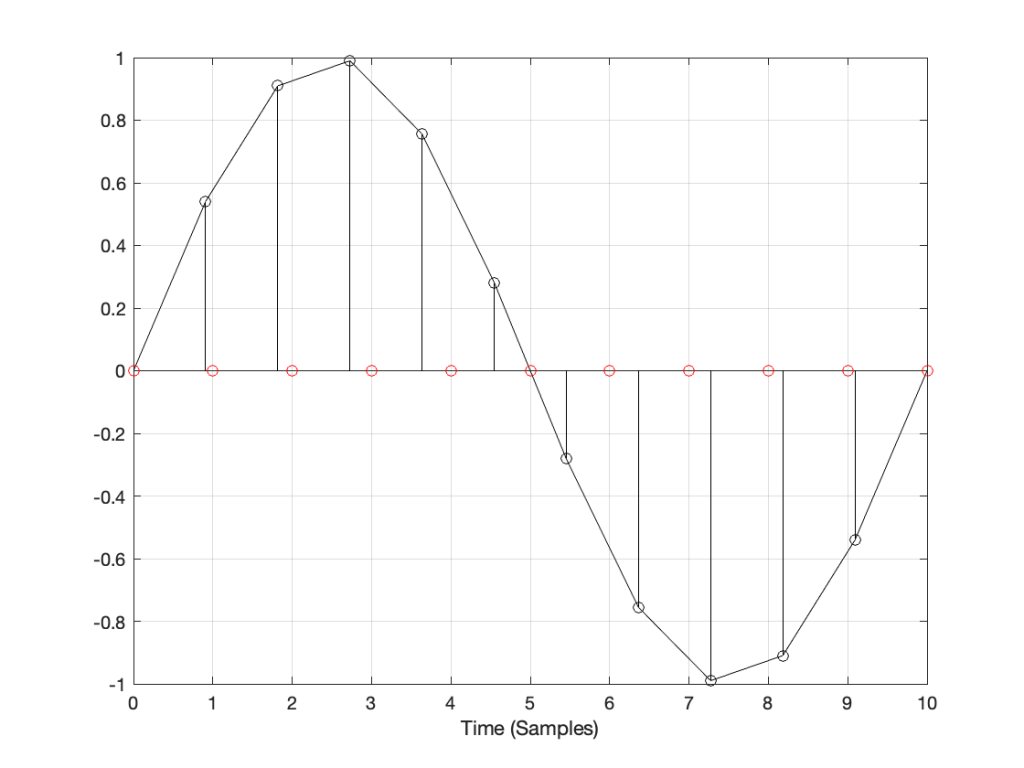Figure 2: The original signal at Fs1 and the new samples that we want to create (in red) at Fs2.

How do we do this? One way is to draw straight lines between the original samples, and calculate the values at the point on the line that corresponds with the time of the new samples. This is called “linear interpolation” (because it’s based on drawing straight lines between the original samples), and it’s shown in Figure 3.

A better way to do this is to use some fancy math to calculate where the signal would be after the reconstruction filter smoothed it back to the original (band-limited) input. There are different ways to do this (in other words, different mathematical strategies) that are outside the scope of this posting, however, I’ve shown an example of a piecewise cubic spline interpolation implementation in Figure 4, below.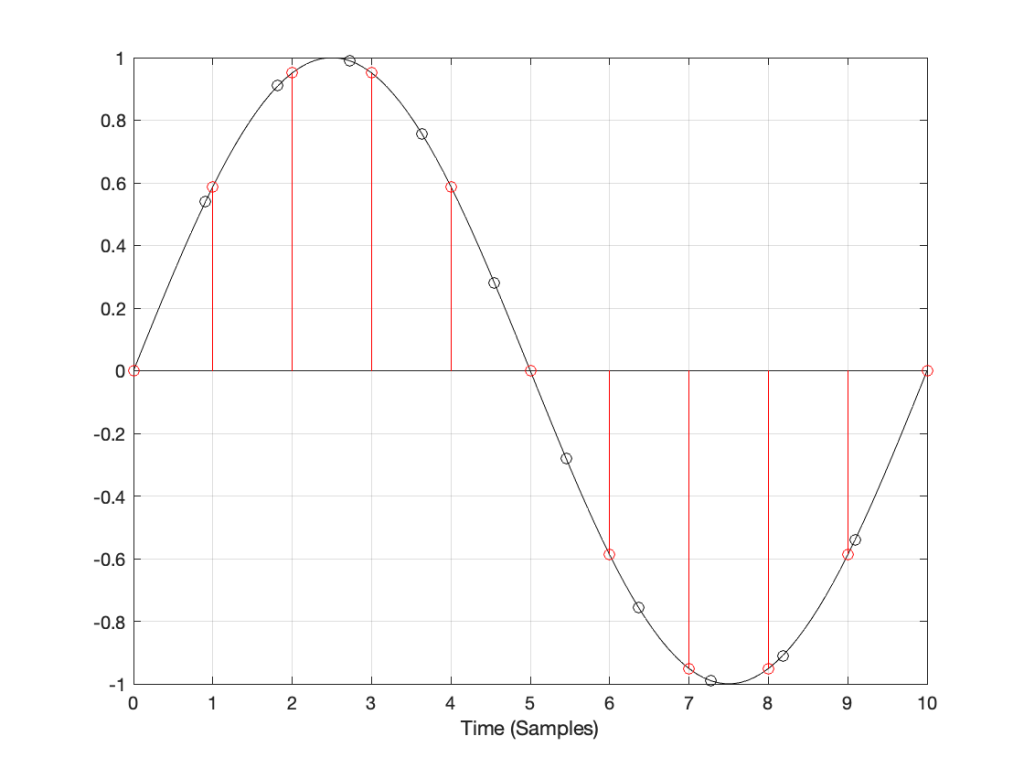Figure 4: An example of piecewise cubic spline interpolation for converting to the new sampling rate.

However, let’s say that:

• you’ve been given the job of building a sampling rate converter, but
• you think that the examples I gave above are way to complicated…

What do you do? One possibility is to look at the sample value that you want to output, find the closest sample (in time) in the original signal, and use that. This is a technique commonly called “nearest neighbour” for obvious reasons – and it’s one of the worst-performing SRC strategies you can use. An example of this is shown in Figure 5, below. Notice that the new values (the red circles) are identical to the closest original value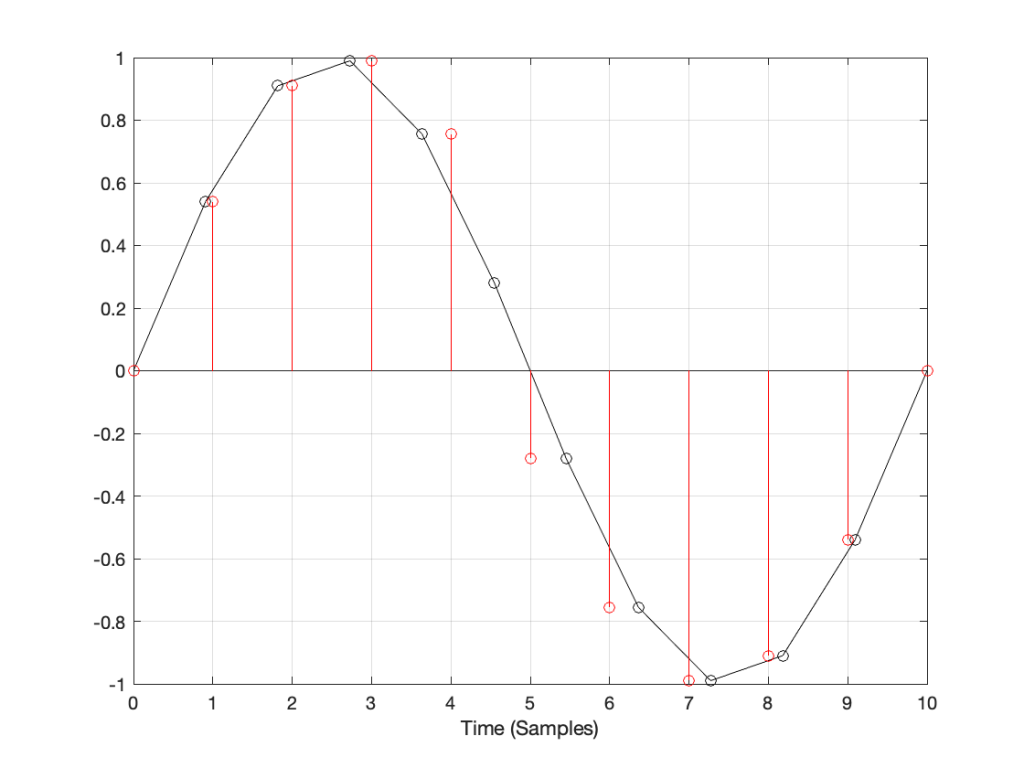Figure 5: An example of “nearest neighbour” interpolation for converting to the new sampling rate. Note that each new values in red is a copy of the closest value in black.

If we look at these two signals without the sample values, we’ll see some pretty nasty distortion in the time domain, as shown in Figure 6.

## So what?

The plots above show the results of good and bad SRCs in the time domain, but what does this look like in the frequency domain? Let’s take a couple of specific examples.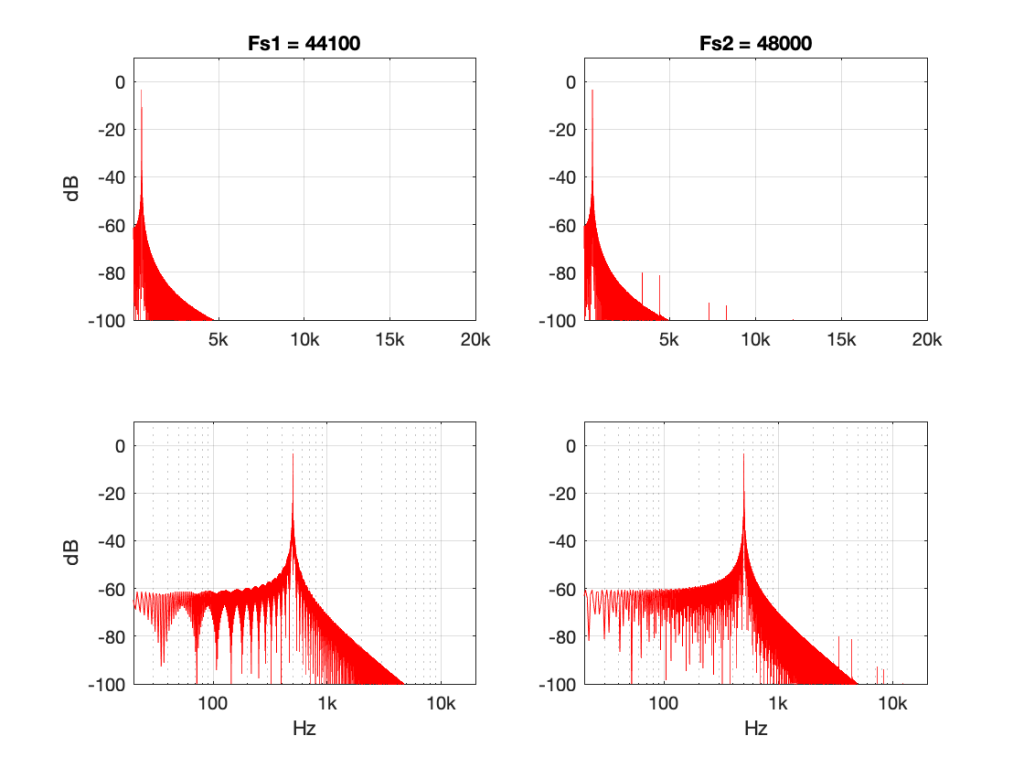Figure 7: 500 Hz sine tone at 0 dB FS, Linear interpolation from 44.1 kHz to 48 kHz.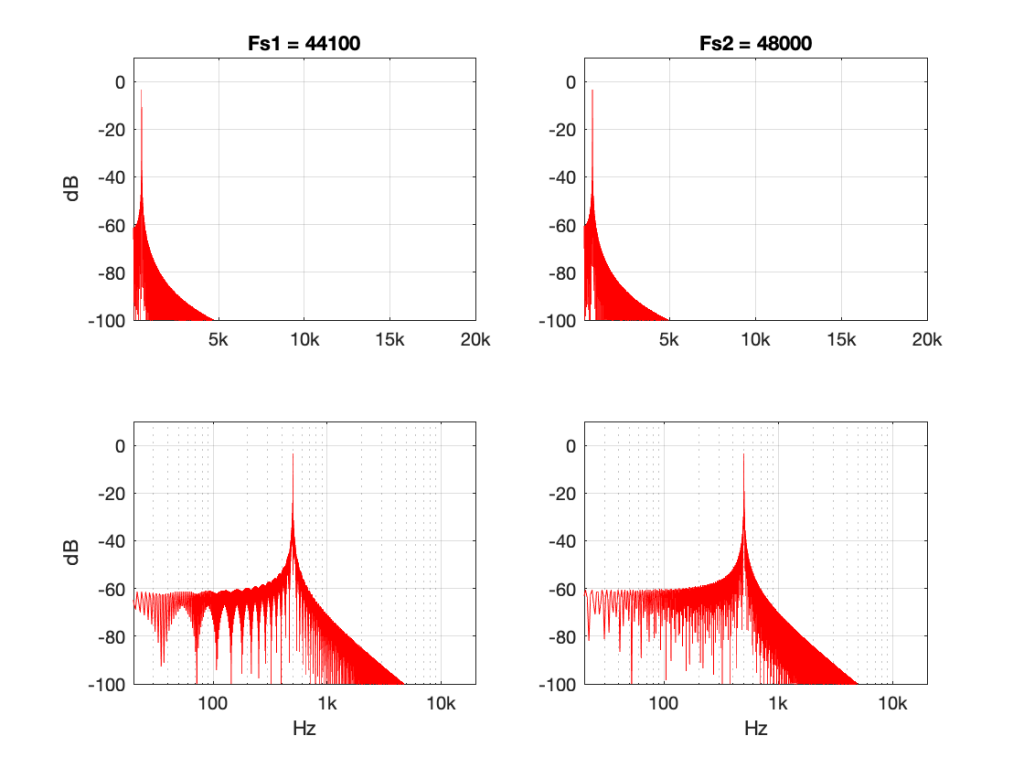Figure 8: 500 Hz sine tone at 0 dB FS, Piecewise cubic spline interpolation from 44.1 kHz to 48 kHz.

Figures 7 and 8 look almost identical. There are the windowing artefacts of the frequency analysis that I’m doing are larger than most of the artefacts caused by the interpolation implementations. However, you may notice a couple of spikes sticking up between 1 kHz and 10 kHz in Figure 7. These are the most obvious frequency-domain artefacts of the distortion caused by linear interpolation. Notice however, that those artefacts are about 80 dB down from the signal – so that’s pretty good for a cheap implementation.

However, let’s look at the same 500 Hz tone converted using the “nearest neighbour” strategy.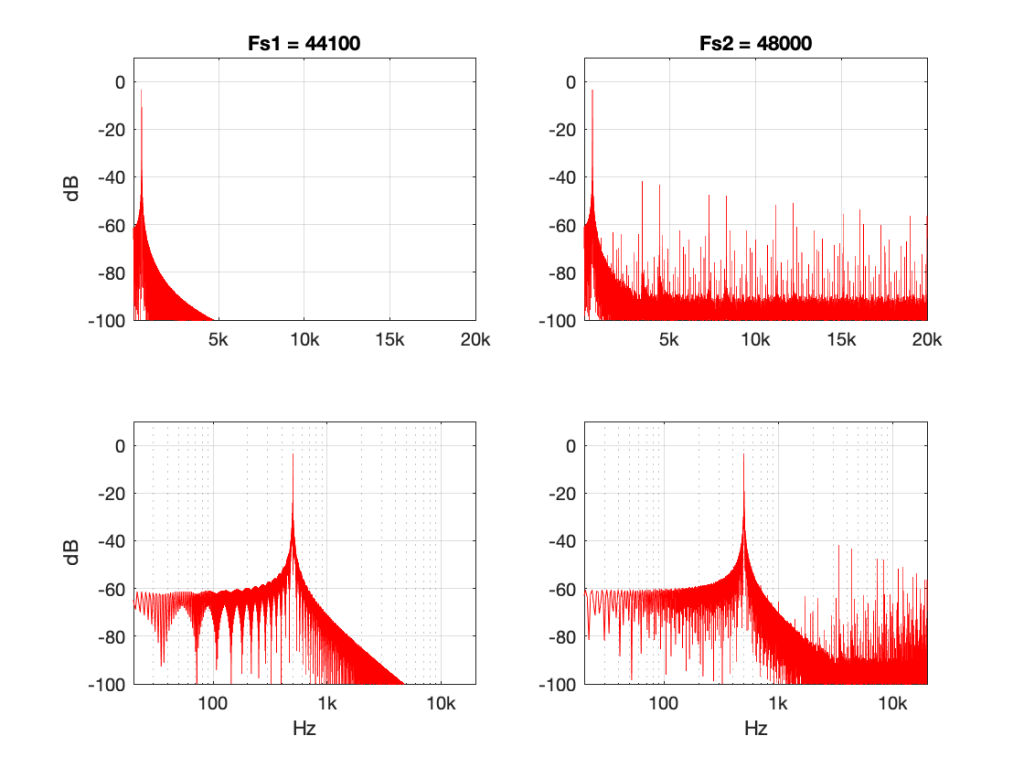Figure 9: 500 Hz sine tone at 0 dB FS, “nearest neighbour” interpolation from 44.1 kHz to 48 kHz.

Now you can see that things have really fallen apart The artefacts are almost up to 40 dB below the signal level, and they’re quite far away in frequency, so they’ll be easy to hear. Also remember that the artefacts that are generated here are inside the audio band, so they will not be eliminated later in the chain by a reconstruction filter in a DAC, for example. They’re there to stay.

There’s one more interesting thing to consider here. Let’s try the same nearest neighbour algorithm, converting between the same two sampling rates, but I’ll put in signals at different frequencies.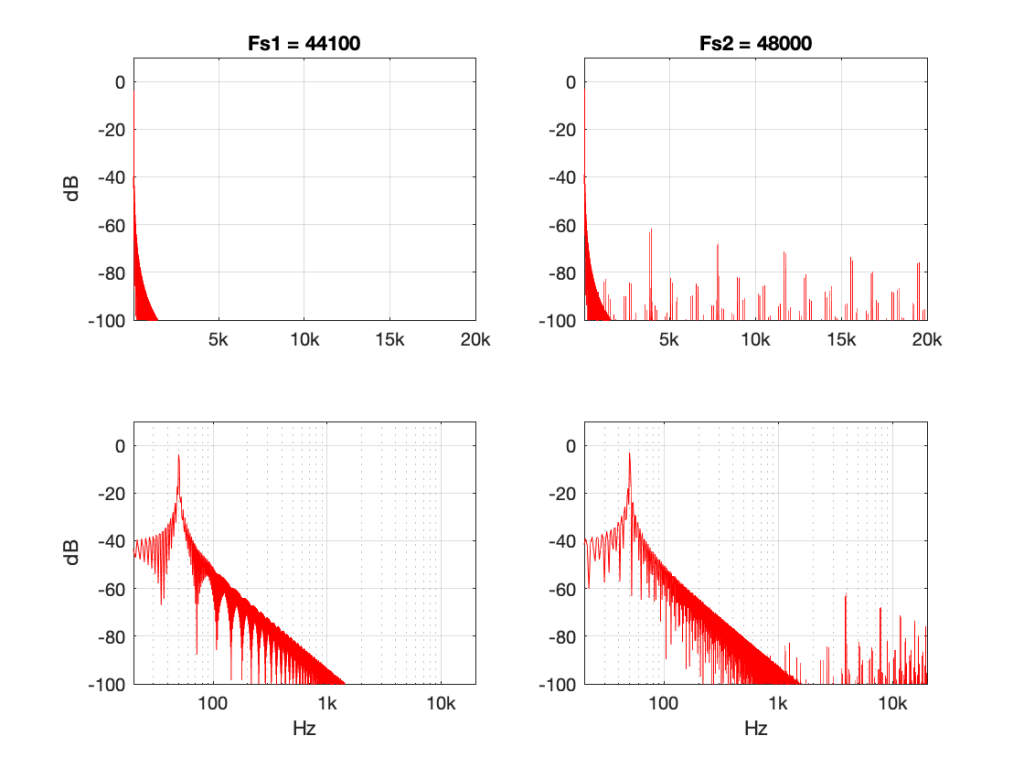Figure 10: 50 Hz sine tone at 0 dB FS, “nearest neighbour” interpolation from 44.1 kHz to 48 kHz.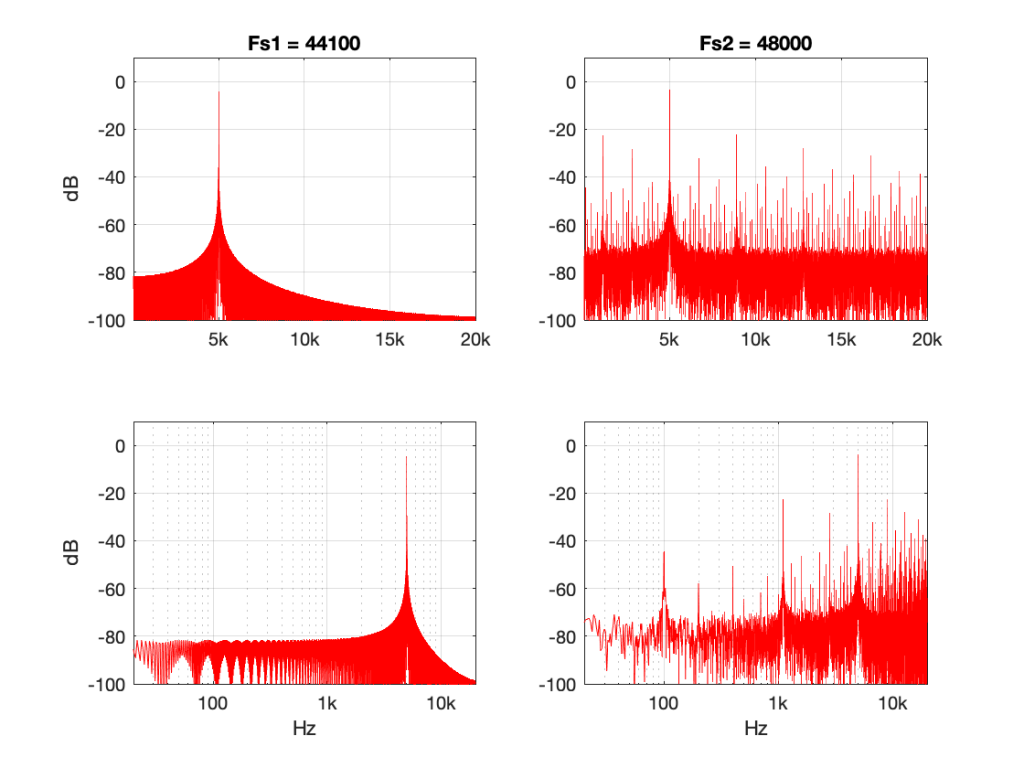Figure 11: 5 kHz sine tone at 0 dB FS, “nearest neighbour” interpolation from 44.1 kHz to 48 kHz.

Figure 10 shows the same system, but the input signal is a 50 Hz sine wave (instead of 500 Hz). Notice that the artefacts are now about 60 dB down (instead of 40 dB).

Figure 11 shows the same system again, but the input signal is a 5 kHz sine wave. Notice that the artefacts are now only about 20 dB down.

So, with this poor implementation of an SRC, the distortion-to-signal ratio is not only dependent on the algorithm itself, but the signal’s frequency content. Why is this?

Think back to the way the “nearest neighbour” strategy works. You’re simply copying-and-pasting the value of the nearest sample. However, the lower the frequency, the less change there is in the signal from sample to sample. So, as your signal’s frequency goes down (more accurately, as it gets further away from the sampling rate), the smaller the error that you create with this system. At 0 Hz, there would be no error, because all of the samples would have the same value.

So, (for example) if your job is to build the SRC in the first place, and you measure it with a 50 Hz tone, you’ll see that the artefacts are 60 dB below the signal and you’ll pat yourself on the back and go to lunch. Then, some weeks later, when the customer complaints start coming in about tweeter distortion, you’ll think it must be someone else’s fault… but it isn’t…

## Conclusion

What does this have to do with “High Resolution Audio”? Well, the problem is that most audio gear does not run at crazy-high sampling rates (this is not necessarily a bad thing), so if you play a high-res file, you’re probably sampling rate converting (this is not necessarily a bad thing).

However, if your gear does have a bad SRC in the signal flow (and, yes, this is not uncommon with modern audio gear) then you either need to

• play the signal with a different (e.g. not-high-res) sampling rate to find out if it’s better,
OR
OR
• at least check for a firmware update.

Note that first recommendation of the three: Because the quality of a sampling rate converter is very dependent upon the relationship between the input and the output sampling rates, it can happen that a “normal” resolution audio signal (say, at 44.1 kHz) will sound better on your particular equipment than a “high” resolution audio signal (say, at 192 kHz) because of this. Of course, the opposite could be true (say, because your gear is running at 48 kHz and it’s easier to get to that from 192 kHz (just multiply by 1/4) than it is to get there from 44.1 kHz (just multiply by 480/441…)

This doesn’t mean that “low-res” is better than “high-res” – it just means that your particular equipment deals with it better. (In the same way that purely from the point of view as a fuel, gasoline might have more energy per litre than diesel fuel, but it’s a terrible choice to put in the tank of a car that’s expecting diesel…)

# “High-Res” Audio: Part 6: Noise, noise, noise

In Part 2 of this series, I talked about the relationship between the noise floor of an LPCM signal and the number of bits used to encode it.

Assuming that the signal is correctly dithered using TPDF dither with a peak-to-peak amplitude of ±1 LSB, then this means that you can easily calculate the dynamic range of your system with a very simple equation:

Dynamic Range in dB = 6.02 * NumberOfBits – 3

(Note that the sampling rate is not part of this equation… That will be useful information later.)

Normally, we’re lazy and we say 6 times the number of bits -3 for the dither – but if you’re really lazy, you leave out the -3 as well.

So, this means that, in a 16-bit system, the noise floor is 93 dB below a sine wave at full scale (6 * 16 -3 = 93) and for a 24-bit system, the noise floor is 141 dB below a sine wave at full scale (you do the math as practice).

Also, we can generalise and say that “adding 1 bit halves the level of the noise floor” (because -6 dB is the same as multiplying by 0.5). However, this is only part of the story.

The noise that’s generated by dither has a “white” characteristic. This means that there is an equal probability of getting some energy per bandwidth (or some say “per Hertz”) over a period of time. This sounds a little complicated, so I’ll explain.

Noise is random. This means that you may or may not get energy at, say 1 kHz, in a given short measurement. However, if you measure white noise for long enough, you’ll eventually see that you got something in every frequency band. Also, you’ll see that, if you look back over the entire length of your measurement of white noise, you got the same amount of energy in the band from 100 Hz to 200 Hz as you did in the band from 1000 Hz to 1200 Hz and the band from 10,000 Hz to 10,200 Hz. (Each of those bandwidths is 200 Hz wide).

There are now two things to discuss:

1. This distribution of energy is not like the way we hear things. We don’t hear the distance between 100 Hz and 200 Hz as the same distance as going from 1,000 Hz to 1,200 Hz. We hear logarithmically, which means that we hear in multiples of frequency, not additions of bandwidth. So, to use 100 Hz – 200 Hz sounds like the same “distance” as 1,000 Hz to 2,000 Hz. This is why white noise sounds like it is “bright” – or it has emphasis on the high frequencies. If you have a system that has a flat response from 0 to 20,000 Hz, and you play white noise through it, you have the same amount of energy in the top octave (10 kHz to 20 kHz) as you do in all of the octaves below – which is why we hear this as “top-heavy”.
2. If you had two bands of white noise with equal levels, and let’s say that one ranges from 100 Hz to 200 Hz, and the other is 1000 Hz to 1200 Hz, then the output level of the two of them together will be 3 dB louder than the output level of either of them alone. This is because their powers add together instead of their amplitudes (because the two signals are unrelated to each other).

Let’s put all this (and one or two other things) together:

1. We know from a previous part in this series that an LPCM digital audio system cannot have signals higher than the Nyquist frequency – 1/2 the sampling rate.
2. TPDF dither is white noise at a total level that is (6.02 * NumberOfBits – 3) dB below full-scale.
3. If you add white noise signals with equal levels but different bandwidths, you get a 3 dB increase over the level of just one of them

This means that,

• if I have a 16-bit, TPDF dithered LPCM audio signal with a sampling rate of 48 kHz, it has a noise floor that is 93 dB below full scale, and that noise has a white characteristic with a bandwidth of 24 kHz (the Nyquist frequency). There will be no noise above that frequency coming out of the system.
• if I have a 16-bit, TPDF dithered LPCM audio signalwith a sampling rate of 192 kHz, it has a noise floor that is 93 dB below full scale, and that noise has a white characteristic with a bandwidth of 96 kHz (the Nyquist frequency). There will be no noise above that frequency coming out of the system.

So, the two systems have the same noise floor level overall, but with very different bandwidths… What does this mean?

Well, let’s start by looking at the level of the noise floor in the 48 kHz system (so the noise “only” extends to 24 kHz).

If I double the sampling rate (to 96 kHz), I double the bandwidth of the noise without changing its level, so this means that the portion of the noise that “lives” in the 0 Hz – 24 kHz region drops by 3 dB (because I’m ignoring the top half of the signal ranging from 24 kHz to 48 kHz in the 96 kHz system.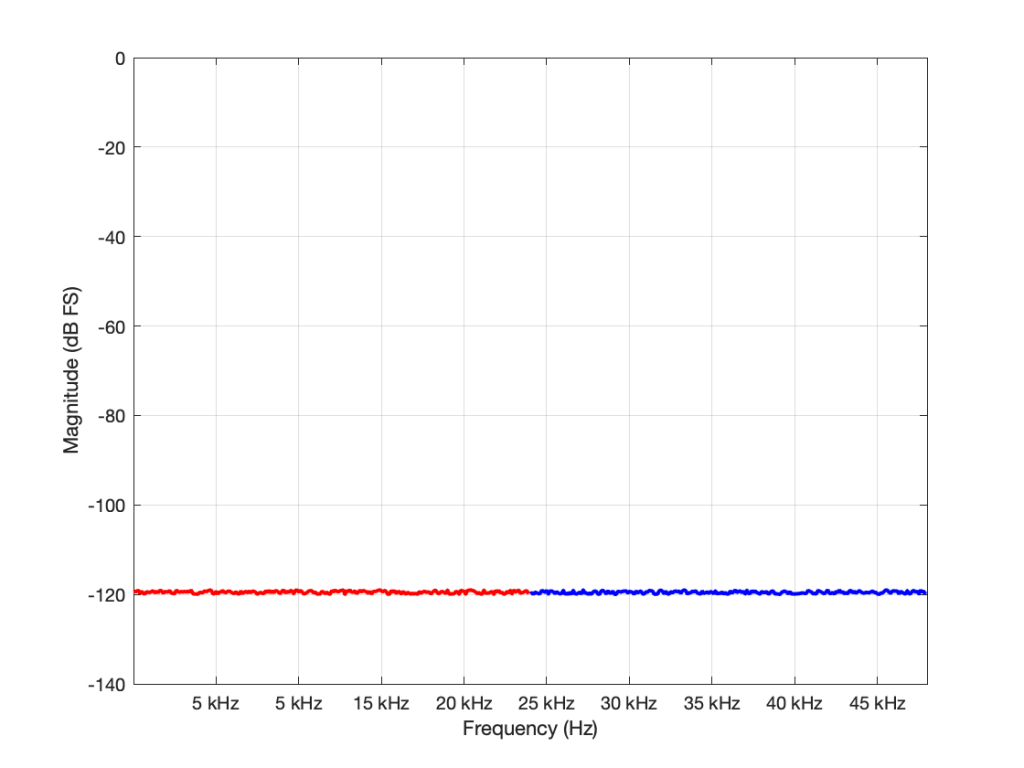Figure 1: The noise floor caused by TPDF dither in a 16-bit LPCM system with a sampling rate of 96 kHz. The total power of the noise drawn in red is the same as the total power of the noise drawn in blue. But notice that this is plotted on a linear scale for frequency.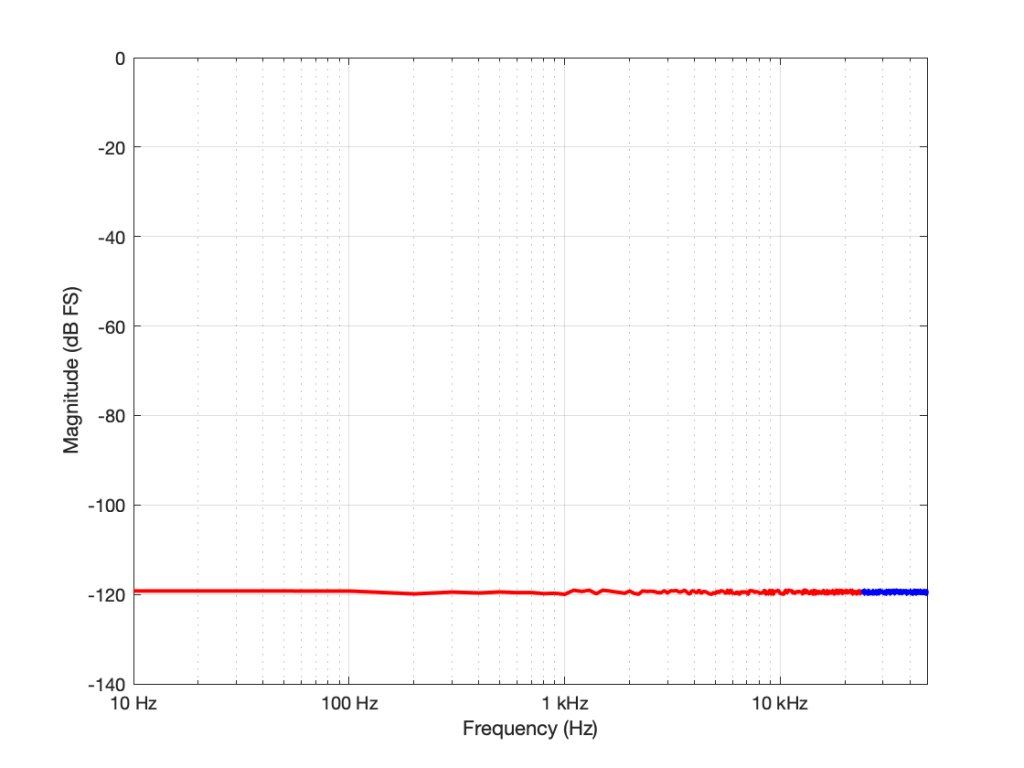Figure 1: Re-plotted on a logarithmic scale for the frequency. To us, the top octave is not so important, but to a measurement device, it’s half the signal…

If I had multiplied the original sampling rate by 4 (to 192 kHz) I multiply the bandwidth of the noise by 4 as well (to 96 kHz). This means that the overall level of the noise from 0 to 24 kHz is now 6 dB down from the original version.

In other words: if I multiply the sampling rate by two, but I don’t increase the bandwidth of the noise floor that I’m interested in (say I only care about 20 Hz – 20 kHz), then its level drops by 3 dB.

## So what?

Well, you could jump to the conclusion that this proves that higher sampling rates are better. However, that would be a bit (ha hah) premature. Consider that, if you want to drop the (band-limited) noise floor by 6 dB, you have to quadruple the sampling rate – and therefore quadrupling the data rate (and therefore the disc storage, the bandwidth of the transmission system, the error rate, and so on…) A 400% increase in the data is not insignificant.

OR, you could just add one more bit – going from 16 bits to 17 bits will give you the same result with a data increase of only 6.25% – a much smarter decision, no?

## The Real World

This little analysis above makes a basic (and possibly incorrect) assumption. The assumption is that, by quadrupling the sampling rate, all other components in the system will remain predictably identical. This may not be true. For example, many DACs (especially older ones) exhibit an increase in their own noise floor when you use them at a higher sampling rate. So, it could be that the benefit you get theoretically is negated by the detriment that you actually get. This is just one example of a flaw in the theory – but it’s a very typical one – especially if you’re building a product instead of just using one.

## P.S.

You may have looked at Figures 1 or 2 and are wondering why, if the noise floor is at -93 dB FS in a 16-bit system, I plotted it around -120 dB FS (give or take). The reason is related to the explanation I just gave above. I said in the captions that it’s from a 96 kHz system. This means that the noise extends to the Nyquist frequency at 48 kHz, and that total level is at -93 dB FS. We also know that, if I keep the noise the same, but half the bandwidth that I’m looking at, the level drops by 3 dB. Therefore I can either do math or I can make the following table:

If you look carefully at the figures, you’ll see that there’s a point every 100 Hz. (It’s most easily visible in the low-frequency range of Figure 2.) So, the level of the noise that I see on a magnitude response plot like this is not only dependent on the noise level itself, but the bandwidths of the divisions that I’ve used to slice it up. In my case, the bandwidth per “slice” is about 100 Hz, so the noise level of each of those little contributors is at about -120 dB FS. If I had used slices only 50 Hz wide, it would show up at -123 instead…

# “High-Res” Audio: Part 5 – Mirrors are bad

Let’s go back to something I said in the last post:

## Mistake #1

I just jumped to at least three conclusions (probably more) that are going to haunt me.

The first was that my “digital audio system” was something like the following:

As you can see there, I took an analogue audio signal, converted it to digital, and then converted it back to analogue. Maybe I transmitted it or stored it in the part that says “digital audio”.

However, the important, and very probably incorrect assumption here is that I did nothing to the signal. No volume control, no bass and treble adjustments… nothing.

If you consider that signal flow from the position of an end-consumer playing a digital recording, this was pretty easy to accomplish in the “old days” when we were all playing CDs. That’s because (in a theoretical, oversimplified world…)

• the output of the mixing/mastering console was analogue
• that analogue signal was converted to digital in the mastering studio
• the resulting bits were put on a disc
• you put that disc in your player which contained a DAC that converted the signal directly to analogue
• you then sent the signal to your “processing” (a.k.a. “volume control”, and maybe some bass and treble adjustment.).

So, that flowchart in Figure 1 was quite often true in 1985.

These days, things are probably VERY different… These days, the signal path probably looks something more like this (note that I’ve highlighted “alterations” or changes in the bits in the audio signal in red):

• The signal was converted from analogue to digital in the studio
(yes, I know… studios often work with digital mixers these days, but at least some of the signals within the mix were analogue to start – unless you are listening to music made exclusively with digital synthesizers)
• The resulting bits were saved on a file
• Depending on the record label, the audio signal was modified to include a “watermark” that can identify it later – in court, when you’ve been accused of theft.
• The file was transferred to a storage device (let’s say “hard drive”) in a large server farm renting out space to your streaming service
• The streaming service encodes the file
• If the streaming service does not offer an lossless option, then the file is converted to a lossy format like MP3, Ogg Vorbis, AAC, or something else.
• If the streaming service offers a lossless option, then the file is compressed using a format like FLAC or ALAC (This is not an alteration, since, with a lossless compression system, you don’t lose anything)
(it might look like an audio player – but that means it’s just a computer that you can’t use to check your social media profile)
• You press play, and the signal is decoded (either from the lossy CODEC or the compression format) back to LPCM. (Still not an alteration. If it’s a lossy CODEC, then the alteration has already happened.)
• That LPCM signal might be sample-rate converted
• The streaming service’s player might do some processing like dynamic range compression or gain changes if you’ve asked it to make all the songs have the same level.
• All of the user-controlled “processing” like volume controls, bass, and treble, are done to the digital signal.
• The signal is sent to the loudspeaker or headphones
• If you’re sending the signal wirelessly to a loudspeaker or headphones, then the signal is probably re-encoded as a lossy CODEC like AAC, aptX, or SBC.
(Yes, there are exceptions with wireless loudspeakers, but they are exceptions.)
• If you’re sending the signal as a digital signal over a wire (like S/PDIF or USB), the you get a bit-for-bit copy at the input of the loudspeaker or headphones.
• The loudspeakers or headphones might sample-rate convert the signal
• The sound is (finally) converted to analogue – either one stream per channel (e.g. “left”) or one stream per loudspeaker driver (e.g. “tweeter”) depending on the product.

So, as you can see in that rather long and complicated list (it looks complicated, but I’ve actually simplified it a little, believe it or not), there’s not much relation to the system you had in 1985.

Let’s take just one of those blocks and see what happens if things go horribly wrong. I’ll take the “volume control” block and add some distortion to see the result with two LPCM systems that have two different sampling rates, one running at 48 kHz and the other at 194 kHz – four times the rate. Both systems are running at 24 bits, with TPDF dither (I won’t explain what that means here). I’ll start by making a 10 kHz tone, and sending it through the system without any intentional distortion. If we look at those two signals in the time domain, they’ll look like this: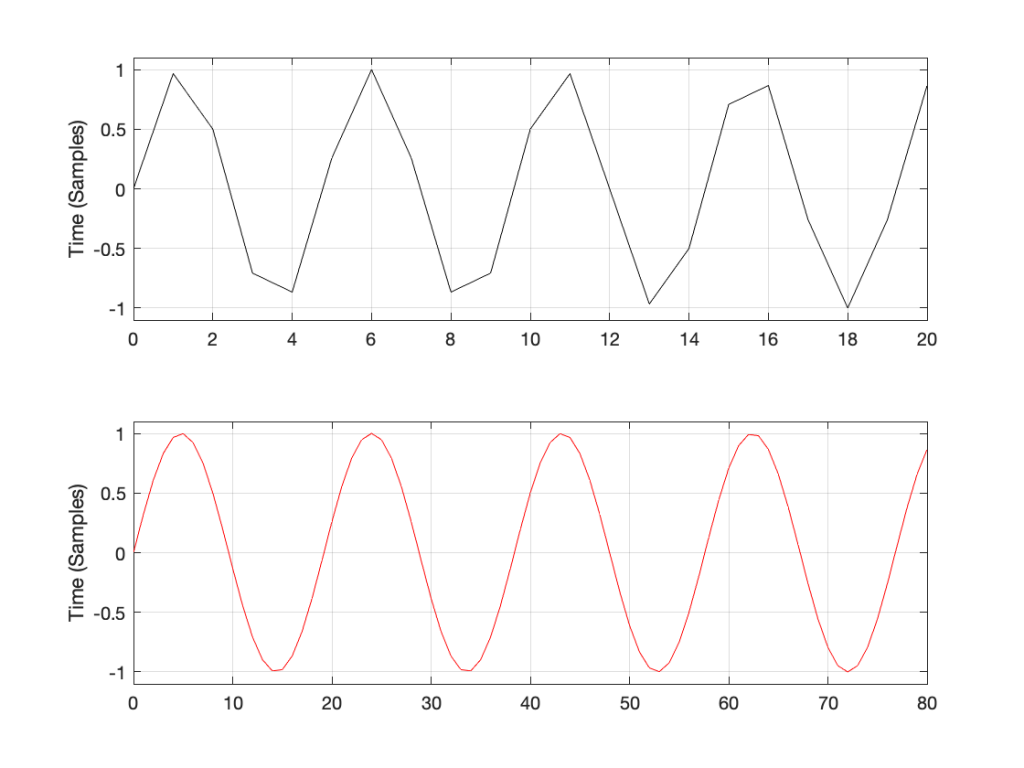Figure 1: Two 10 kHz tones. The black one is in a 48 kHz, 24 bit LPCM system. The red one is in a 192 kHz, 24 bit LPCM system.

The sine tone in the 48 kHz system may look less like a sine tone than the one in the 192 kHz system, however, in this case, appearances are deceiving. The reconstruction filter in the DAC will filter out all the high frequencies that are necessary to reproduce those corners that you see here, so the resulting output will be a sine wave. Trust me.

If we look at the magnitude responses of these two signals, they look like Figure 2, below.

You may be wondering about the “skirts” on either side of the 10 kHz spikes. These are not really in the signal, they’re a side-effect (ha ha) of the windowing process used in the DFT (aka FFT). I will not explain this here – but I did a long series of articles on windowing effects with DFTs, so you can search for it if you’re interested in learning more about this.

If you’re attentive, you’ll notice that both plots extend up to 96 kHz. That’s because the 192 kHz system on the bottom has a Nyquist frequency of 96 kHz, and I want both plots to be on the same scale for reasons that will be obvious soon.

Now I want to make some distortion. In order to make things obvious, I’m going to make a LOT of distortion. I’ve made the sine wave try to have an amplitude that is 10 times higher than my two systems will allow. In other words, my amplitude should be +/- 10, but the signal clips at +/- 1, resulting in something looking very much like a square wave, as shown in Figure 3.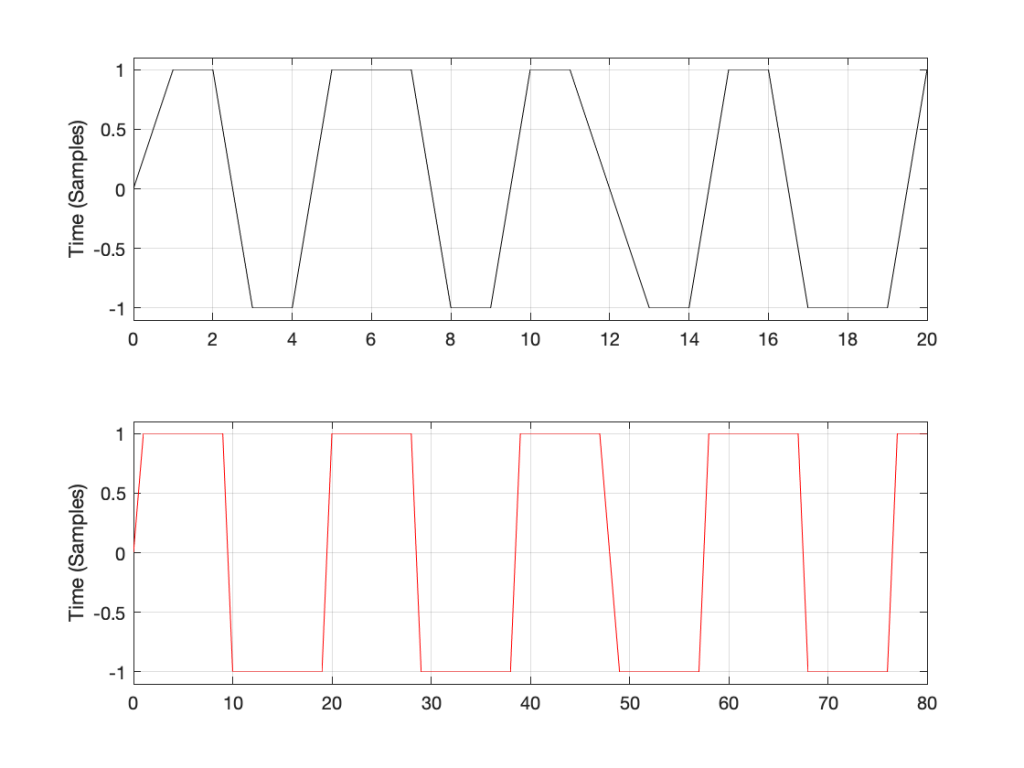Figure 3: Distorted 10 kHz sine waves. The black one is in a 48 kHz, 24 bit LPCM system. The red one is in a 192 kHz, 24 bit LPCM system.

You may already know that if you want to make a square wave by building it up using its constituent harmonics, you need to have the fundamental (which we’ll call Fc. In our case, Fc = 10 kHz) with an amplitude that we’ll say is “A”, you then add the

• 3rd harmonic (3 times Fc, so 30 kHz in our case) with an amplitude of A/3.
• 5th harmonic (5 Fc = 50 kHz) with an amplitude of A/5
• 7 Fc at A/7
• and so on up to infinity

Let’s look at the magnitude responses of the two signals above to see if that’s true.

If we look at the bottom plot first (running at 192 kHz and with a Nyquist limit of 96 kHz) the 10 kHz tone is still there. We can also see the harmonics at 30 kHz, 50 kHz, 70 kHz, and 90 kHz in amongst the mess of other spikes we’ll get to those soon…)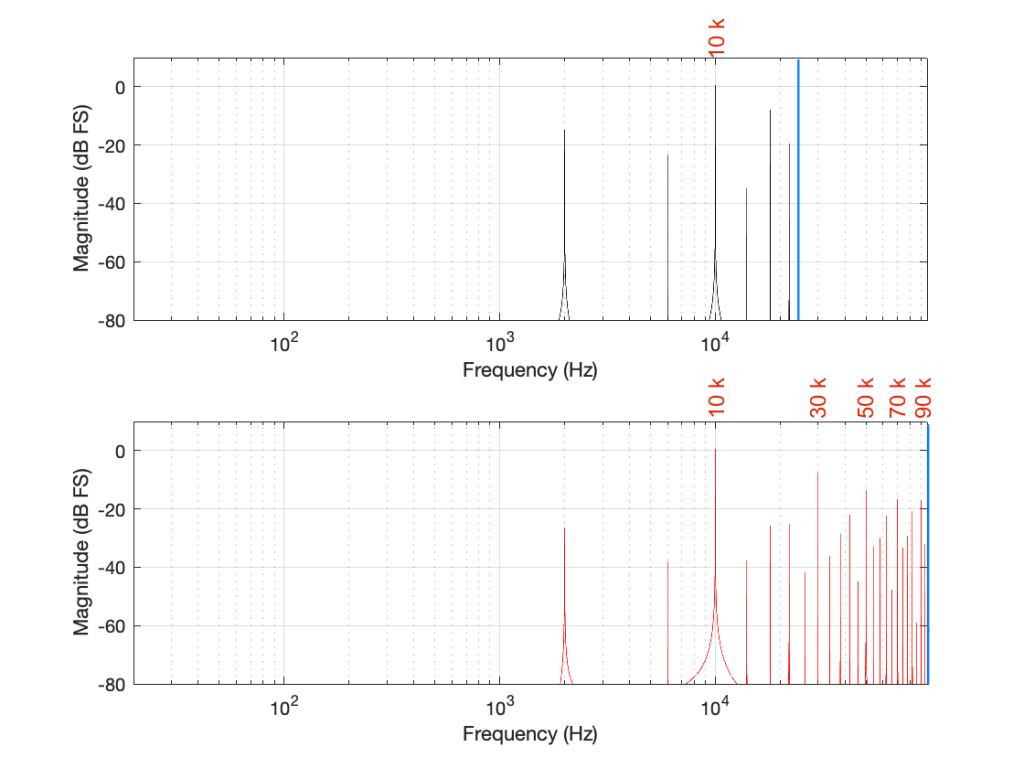Figure 5. Some labels applied to Figure 4 for clarity, showing the harmonics of the square waves that are captured by the two systems

Looking at the top plot (running at 48 kHz and with a Nyquist limit of 24 kHz), we see the 10 kHz tone, but the 30 kHz harmonic is not there – because it can’t be. Signals can’t exist in our system above the Nyquist limit. So, what happens? Think back to the images of the rotating wheel in Part 3. When the wheel was turning more than 1/2 a turn per frame of the movie, it appears to be going backwards at a different speed that can be calculated by subtracting the actual rotation from 180º (half-a-turn).

The same is true when, inside a digital audio signal flow, we try to make a signal that’s higher than Nyquist. The energy exists in there – it just “folds” to another frequency – its “alias”.

We can look at this generally using Figure 6.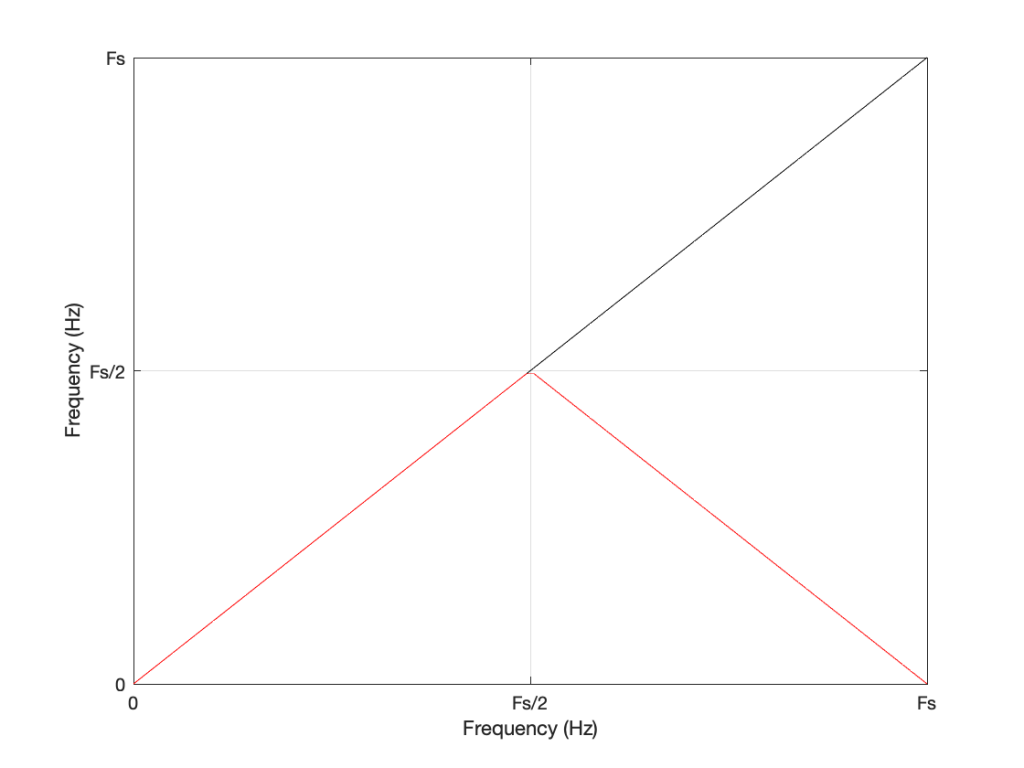Figure 6: A general plot of aliasing, showing the intended frequency in black and the actual output frequency in red.

Looking at Figure 6: If we make a sine tone that sweeps upward from 0 Hz to the Nyquist frequency at Fs/2 (half the sampling rate or sampling frequency) then the output is the same as the input. However, when the intended frequency goes above Fs/2, the actual frequency that comes out is Fs/2 minus the intended frequency. This creates a “mirror” effect.

If the intended frequency keeps going up above Fs, then the mirroring happens again, and again, and again… This is illustrated in Figure 7.

This plot is shown with linear scales for both the X- and Y-axes to make it easy to understand. If the axes in Figure 7 were scaled to a logarithmic scaling instead (which is how “Frequency Response” are normally shown, since this corresponds to how we hear frequency differences), then it would look like Figure 8.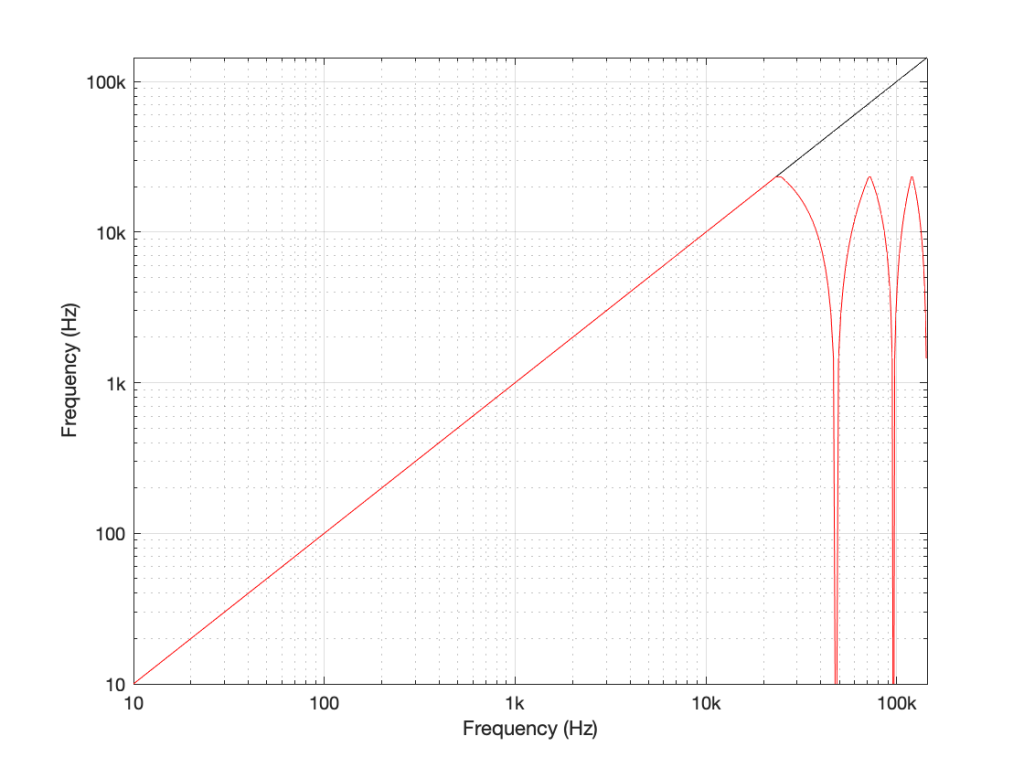Figure 8: The same information shown in Figure 7, plotted on a logarithmic scale instead. Note that this example is for a system running at 48 kHz (therefore with a Nyquist frequency of 24 kHz), and an intended input frequency (in black) going up to 3 times 48 kHz = 144 kHz.

Coming back to our missing 30 kHz harmonic in the 48 kHz LPCM system: Since 30 kHz is above the Nyquist limit of 24 kHz in that system, it mirrors down to 24 kHz – (30 kHz – 24 kHz) = 18 kHz. The 50 kHz harmonic shows up as an alias at 2 kHz. (follow the red line in Figure 7: A harmonic on the black line at 48 kHz would actually be at 0 Hz on the red line. Then, going 2000 Hz up to 50 kHz would bring the red line up to 2 kHz.)

Similarly, the 110 kHz harmonic in the 192 kHz system will produce an alias at 96 kHz – (110 kHz – 96 kHz) = 82 kHz.

If I then label the first set of aliases in the two systems, we get Figure 9.

Now we have to stop for a while and think about what’s happened.

We had a digital signal that was originally “valid” – meaning that it did not contain any information above the Nyquist frequency, so nothing was aliasing. We then did something to the signal that distorted it inside the digital audio path. This produced harmonics in both cases, however, some of the harmonics that were produced are harmonically related to the original signal (just as they ought to be) and others are not (because they’re aliases of frequency content that cannot be reproduced by the system.

What we have to remember is that, once this happens, that frequency content is all there, in the signal, below the Nyquist frequency. This means that, when we finally send the signal out of the DAC, the low-pass filtering performed by the reconstruction filter will not take care of this. It’s all part of the signal.

So, the question is: which of these two systems will “sound better” (whatever that means)? (I know, I know, I’m asking “which of these two distortions would you prefer?” which is a bit of a weird question…)

This can be answered in two ways that are inter-related.

The first is to ask “how much of the artefact that we’ve generated is harmonically related to the signal (the original sine tone)?” As we can see in Figure 5, the higher the sampling rate, the more artefacts (harmonics) will be preserved at their original intended frequencies. There’s no question that harmonics that are harmonically related to the fundamental will sound “better” than tones that appear to have no frequency relationship to the fundamental. (If I were using a siren instead of a constant sine tone, then aliased harmonics are equally likely to be going down or up when the fundamental frequency goes up… This sounds weird.)

The second is to look at the levels of the enharmonic artefacts (the ones that are not harmonically related to the fundamental). For example, both the 48 kHz and the 192 kHz system have an aliased artefact at 2 kHz, however, its level in the 48 kHz system is 15 dB below the fundamental whereas, in the 192 kHz system, it’s more than 26 dB below. This is because the 6 kHz artefact in the 48 kHz system is an alias of the 30 kHz harmonic, whereas, in the 192 kHz system, it’s an alias of the 190 kHz harmonic, which is much lower in level.

As I said, these two points are inter-related (you might even consider them to be the same point) however, they can be generalised as follows:

The higher the sampling rate, the more the artefacts caused by distortion generated within the system are harmonically related to the signal.

In other words, it gives a manufacturer more “space” to screw things up before they sound bad. The title of this posting is “Mirrors are bad” but maybe it should be “Mirrors are better when they’re further away” instead.

Of course, the distortion that’s actually generated by processing inside a digital audio system (hopefully) won’t be anything like the clipping that I did to the signal. On the other hand, I’ve measured some systems that exhibit exactly this kind of behaviour. I talked about this in another series about Typical Problems in Digital Audio: Aliasing where I showed this measurement of a real device: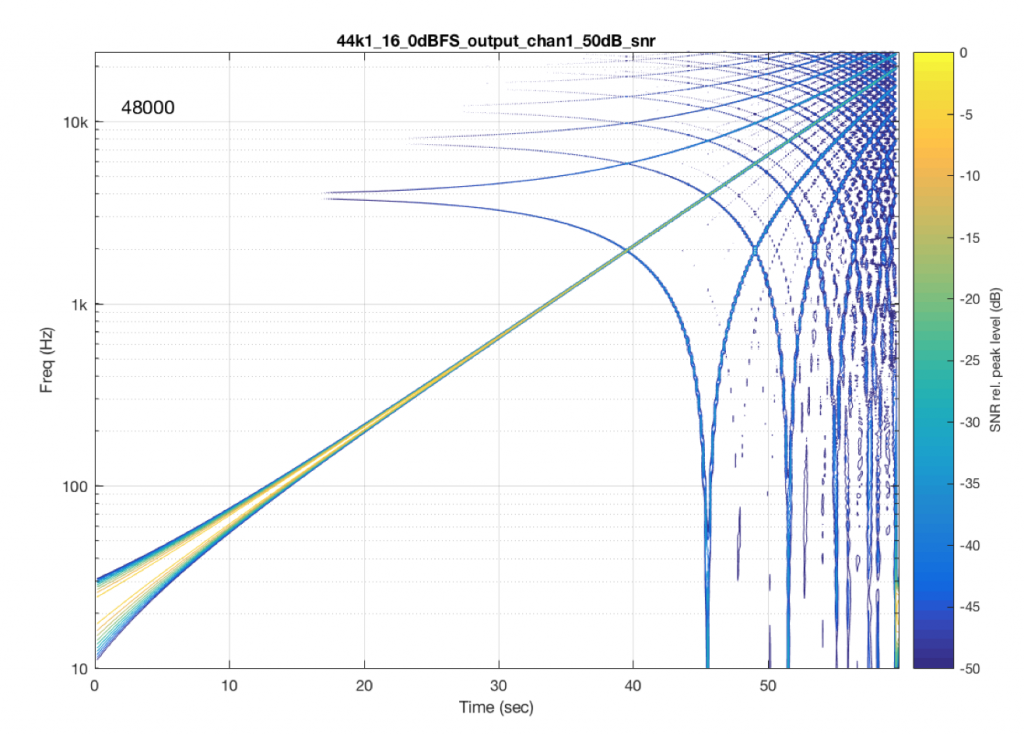Figure 10: A measurement of a real device showing some kind of distortion and aliased artefacts of a swept sine tone. Half of the aliasing is immediately recognizable as going downwards when the tone is going upwards.

However, I’m not here to talk about what you can or can’t hear – that is dependent on too many variables to make it worth even starting to talk about. The point of this series is not to prove that something is better or worse than something else. It’s only to show the advantages and disadvantages of the options so that you can make an informed choice that best suits your requirements.

On to Part 6

# “High-Res” Audio: Part 3 – Frequency Limits

Reminder: This is still just the lead-up to the real topic of this series. However, we have to get some basics out of the way first…

Just like the last posting, this is a copy-and-paste from an article that I wrote for another series. However, this one is important, and rather than just link you to a different page, I’ve reproduced it (with some minor editing to make it fit) here.

In the first posting in this series, I talked about digital audio (more accurately, Linear Pulse Code Modulation or LPCM digital audio) is basically just a string of stored measurements of the electrical voltage that is analogous to the audio signal, which is a change in pressure over time… In the second posting in the series, we looked at a “trick” for dealing with the issue of quantisation (the fact that we have a limited resolution for measuring the amplitude of the audio signal). This trick is to add dither (a fancy word for “noise”) to the signal before we quantise it in order to randomise the error and turn it into noise instead of distortion.

In this posting, we’ll look at some of the problems incurred by the way we carve up time into discrete moments when we grab those samples.

Let’s make a wheel that has one spoke. We’ll rotate it at some speed, and make a film of it turning. We can define the rotational speed in RPM – rotations per minute, but this is not very useful. In this case, what’s more useful is to measure the wheel rotation speed in degrees per frame of the film.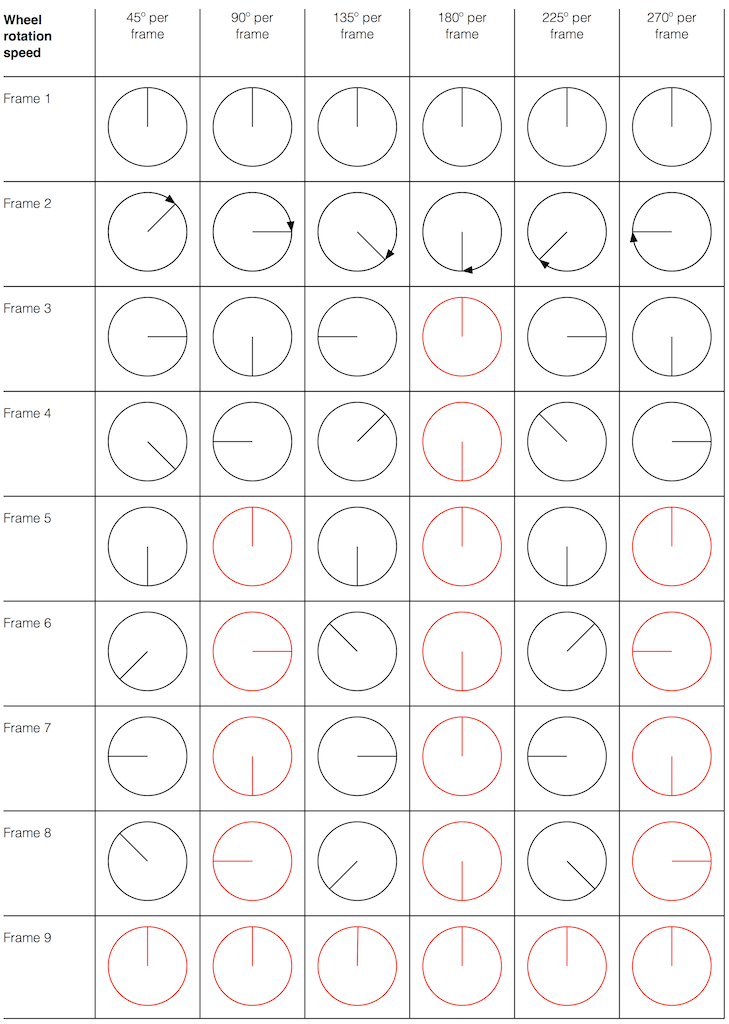Fig 1. The position of a clockwise-rotating wheel (with only one spoke) for 9 frames of a film. Each column shows a different rotational speed of the wheel. The far left column is the slowest rate of rotation. The far right column is the fastest rate of rotation. Red wheels show the frame in which the sequence starts repeating.

Take a look at the left-most column in Figure 1. This shows the wheel rotating 45º each frame. If we play back these frames, the wheel will look like it’s rotating 45º per frame. So, the playback of the wheel rotating looks the same as it does in real life.

This is more or less the same for the next two columns, showing rotational speeds of 90º and 135º per frame.

However, things change dramatically when we look at the next column – the wheel rotating at 180º per frame. Think about what this would look like if we played this movie (assuming that the frame rate is pretty fast – fast enough that we don’t see things blinking…) Instead of seeing a rotating wheel with only one spoke, we would see a wheel that’s not rotating – and with two spokes.

This is important, so let’s think about this some more. This means that, because we are cutting time into discrete moments (each frame is a “slice” of time) and at a regular rate (I’m assuming here that the frame rate of the film does not vary), then the movement of the wheel is recorded (since our 1 spoke turns into 2) but the direction of movement does not. (We don’t know whether the wheel is rotating clockwise or counter-clockwise. Both directions of rotation would result in the same film…)

Now, let’s move over one more column – where the wheel is rotating at 225º per frame. In this case, if we look at the film, it appears that the wheel is back to having only one spoke again – but it will appear to be rotating backwards at a rate of 135º per frame. So, although the wheel is rotating clockwise, the film shows it rotating counter-clockwise at a different (slower) speed. This is an effect that you’ve probably seen many times in films and on TV. What may come as a surprise is that this never happens in “real life” unless you’re in a place where the lights are flickering at a constant rate (as in the case of fluorescent or some LED lights, for example).

Again, we have to consider the fact that if the wheel actually were rotating counter-clockwise at 135º per frame, we would get exactly the same thing on the frames of the film as when the wheel if rotating clockwise at 225º per frame. These two events in real life will result in identical photos in the film. This is important – so if it didn’t make sense, read it again.

This means that, if all you know is what’s on the film, you cannot determine whether the wheel was going clockwise at 225º per frame, or counter-clockwise at 135º per frame. Both of these conclusions are valid interpretations of the “data” (the film). (Of course, there are more – the wheel could have rotated clockwise by 360º+225º = 585º or counter-clockwise by 360º+135º = 495º, for example…)

Since these two interpretations of reality are equally valid, we call the one we know is wrong an alias of the correct answer. If I say “The Big Apple”, most people will know that this is the same as saying “New York City” – it’s an alias that can be interpreted to mean the same thing.

We people in audio commit many sins. One of them is that, every time we draw a plot of anything called “audio” we start out by drawing a sine wave. (A similar sin is committed by musicians who, at the first opportunity to play a grand piano, will play a middle-C, as if there were no other notes in the world.) The question is: what, exactly, is a sine wave?

Get a Slinky – or if you don’t want to spend money on a brand name, get a spring. Look at it from one end, and you’ll see that it’s a circle, as can be (sort of) seen in Figure 2.

Since this is a circle, we can put marks on the Slinky at various amounts of rotation, as in Figure 3.

Of course, I could have put the 0º mark anywhere. I could have also rotated counter-clockwise instead of clockwise. But since both of these are arbitrary choices, I’m not going to debate either one.

Now, let’s rotate the Slinky so that we’re looking at from the side. We’ll stretch it out a little too…

Let’s do that some more…

When you do this, and you look at the Slinky directly from one side, you are able to see the vertical change of the spring from the centre as a result of the change in rotation. For example, we can see in Figure 6 that, if you mark the 45º rotation point in this view, the distance from the centre of the spring is 71% of the maximum height of the spring (at 90º).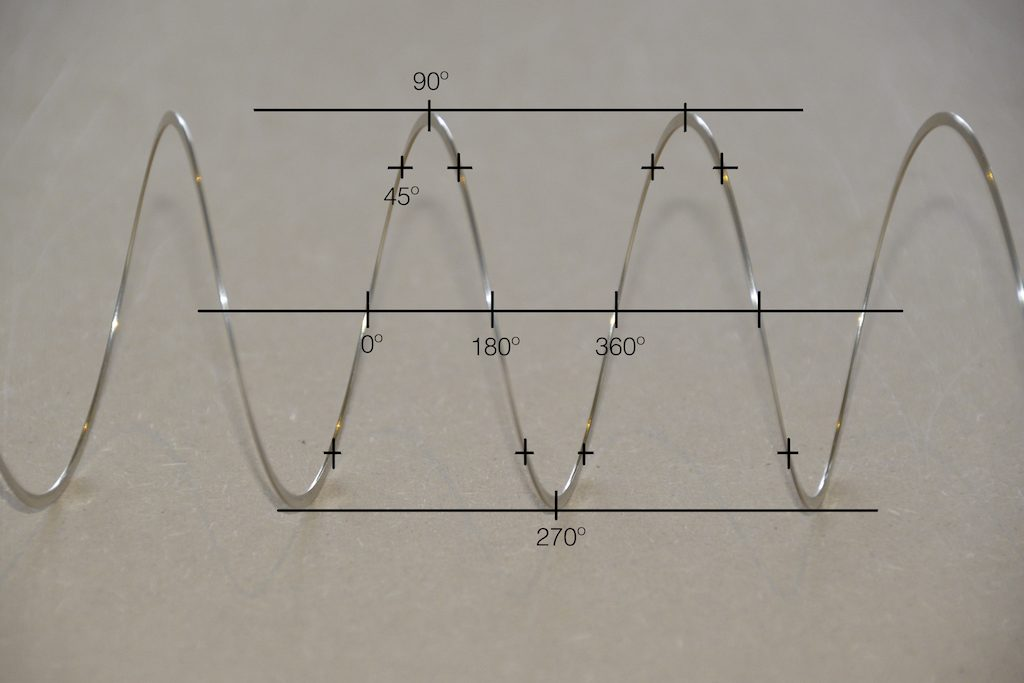Fig 6. The same markings shown in Figure 3, when looking at the Slinky from the side. Note that, if we didn’t have the advantage of a little perspective (and a spring made of flat metal), we would not know whether the 0º point was closer or further away from us than the 180º point. In other words, we wouldn’t know if the Slinky was rotating clockwise or counter-clockwise.

So what? Well, basically, the “punch line” here is that a sine wave is actually a “side view” of a rotation. So, Figure 7, shows a measurement – a capture – of the amplitude of the signal every 45º.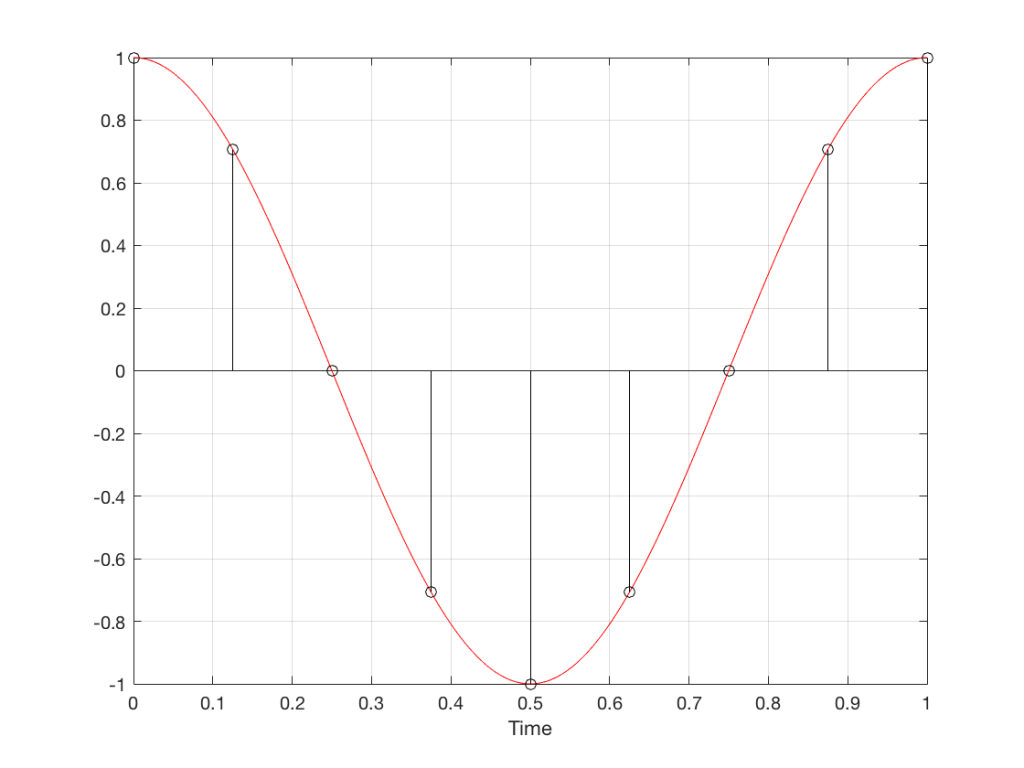Fig 7. Each measurement (a black “lollipop”) is a measurement of the vertical change of the signal as a result of rotating 45º.

Since we can now think of a sine wave as a rotation of a circle viewed from the side, it should be just a small leap to see that Figure 7 and the left-most column of Figure 1 are basically identical.

Let’s make audio equivalents of the different columns in Figure 1.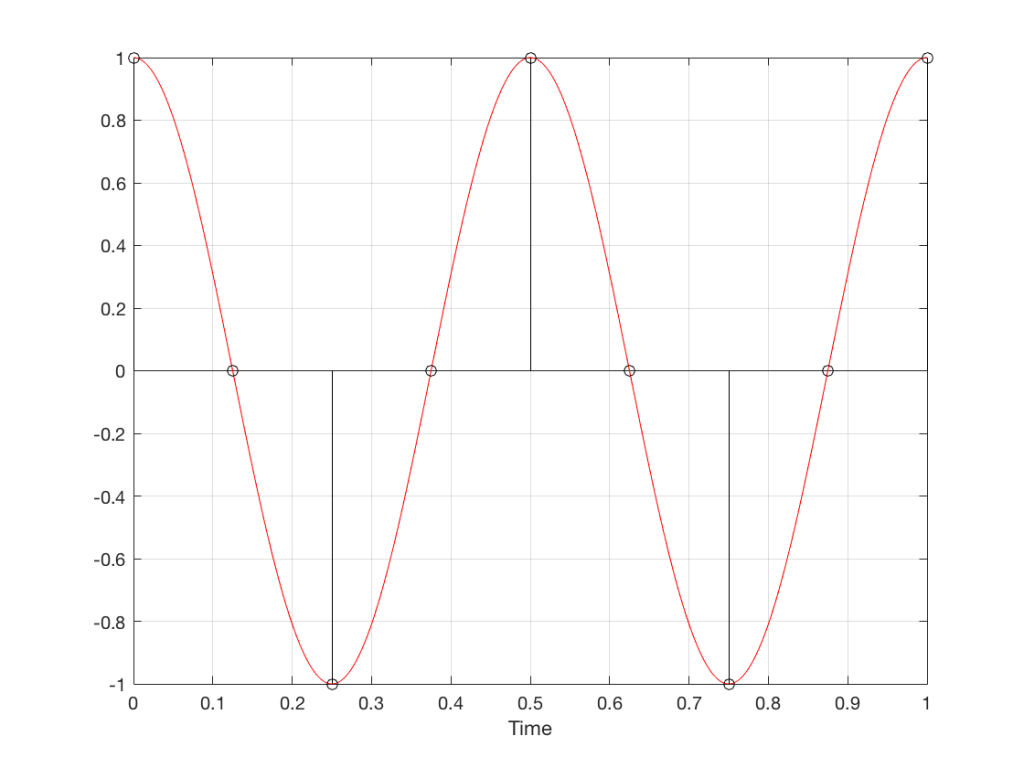Fig 8. A sampled cosine wave where the frequency of the signal is equivalent to 90º per sample period. This is identical to the “90º per frame” column in Figure 1.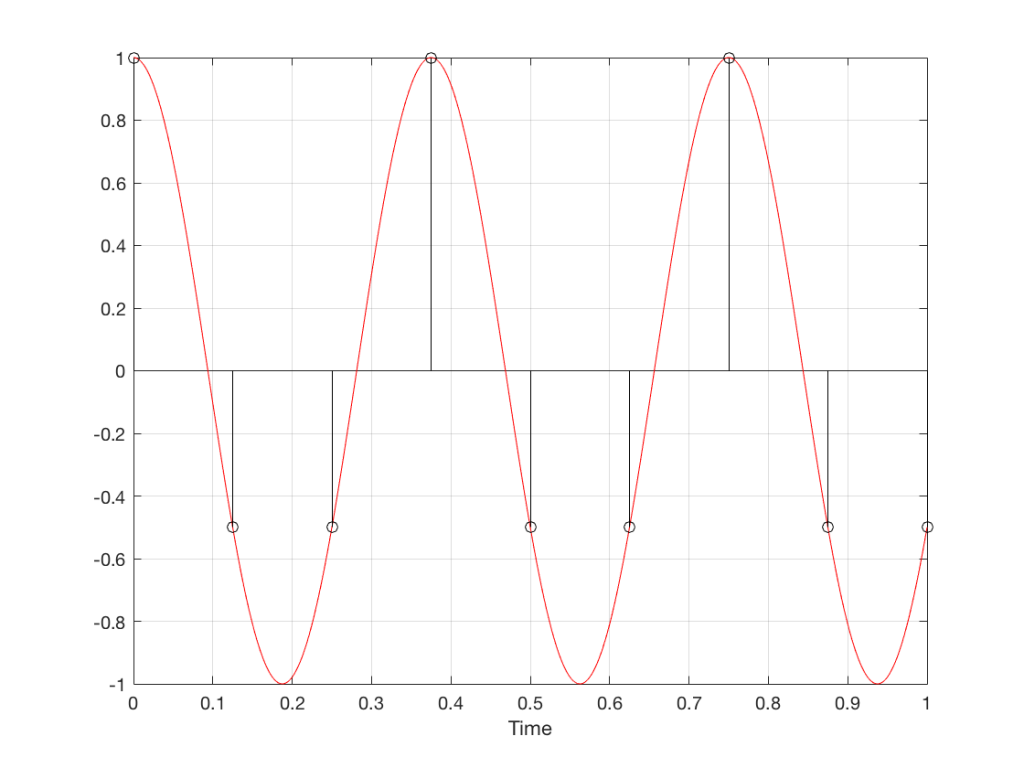Fig 9. A sampled cosine wave where the frequency of the signal is equivalent to 135º per sample period. This is identical to the “135º per frame” column in Figure 1.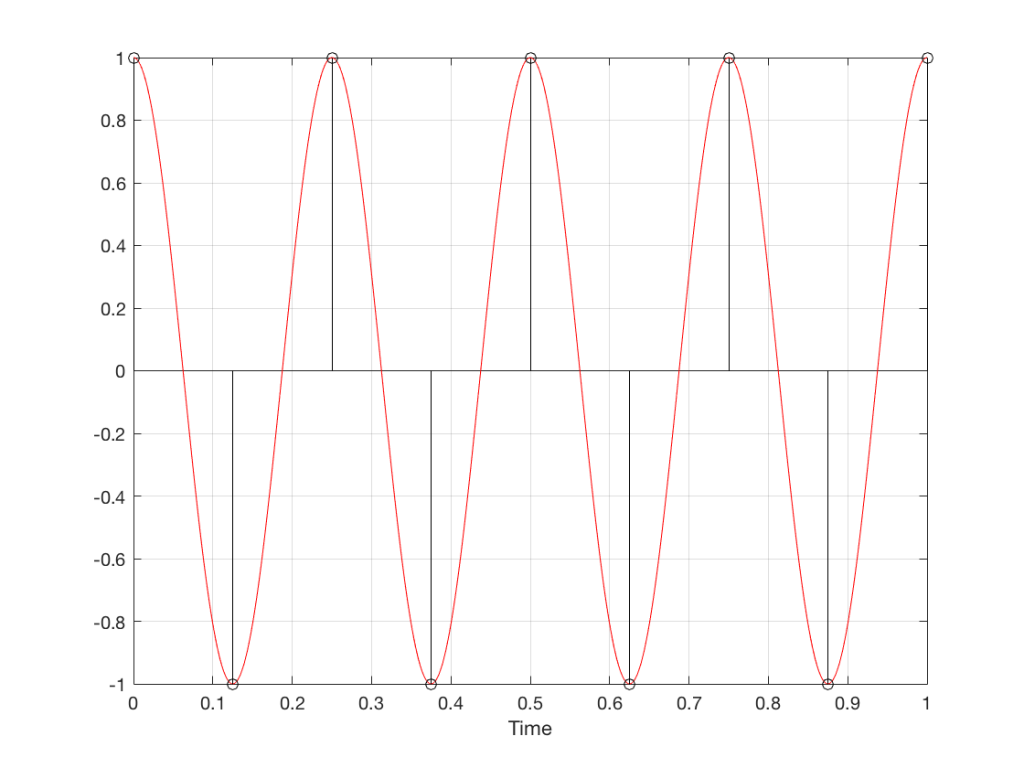Fig 10. A sampled cosine wave where the frequency of the signal is equivalent to 180º per sample period. This is identical to the “180º per frame” column in Figure 1.

Figure 10 is an important one. Notice that we have a case here where there are exactly 2 samples per period of the cosine wave. This means that our sampling frequency (the number of samples we make per second) is exactly one-half of the frequency of the signal. If the signal gets any higher in frequency than this, then we will be making fewer than 2 samples per period. And, as we saw in Figure 1, this is where things start to go haywire.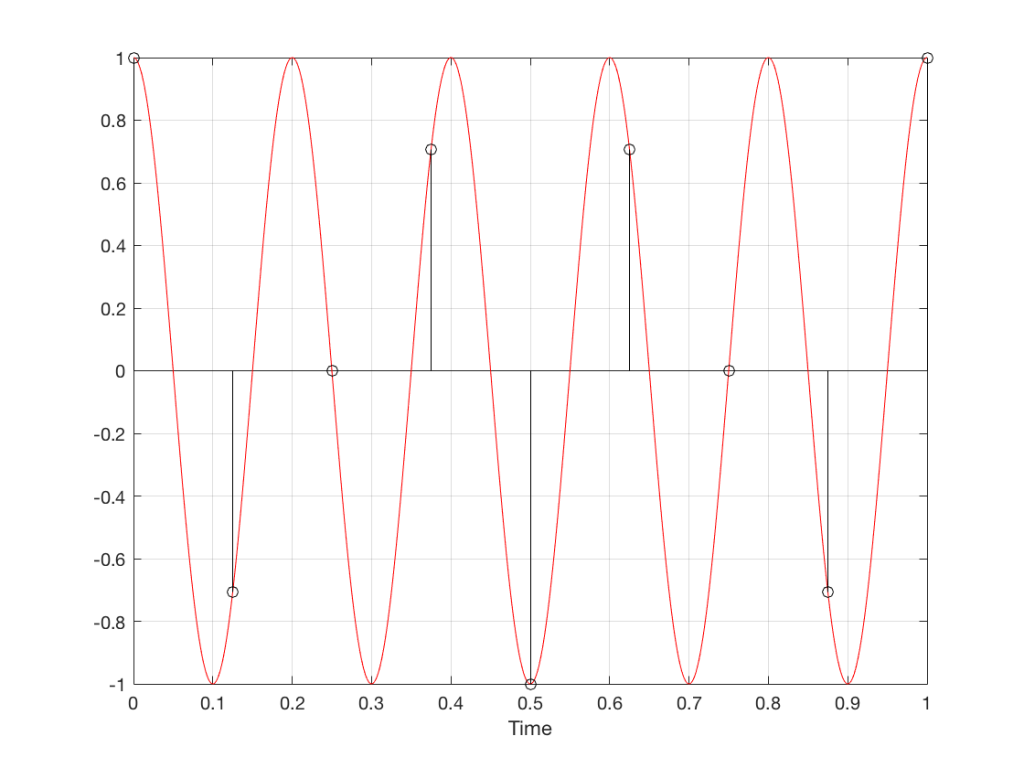Fig 11. A sampled cosine wave where the frequency of the signal is equivalent to 225º per sample period. This is identical to the “225º per frame” column in Figure 1.

Figure 11 shows the equivalent audio case to the “225º per frame” column in Figure 1. When we were talking about rotating wheels, we saw that this resulted in a film that looked like the wheel was rotating backwards at the wrong speed. The audio equivalent of this “wrong speed” is “a different frequency” – the alias of the actual frequency. However, we have to remember that both the correct frequency and the alias are valid answers – so, in fact, both frequencies (or, more accurately, all of the frequencies) exist in the signal.

So, we could take Fig 11, look at the samples (the black lollipops) and figure out what other frequency fits these. That’s shown in Figure 12.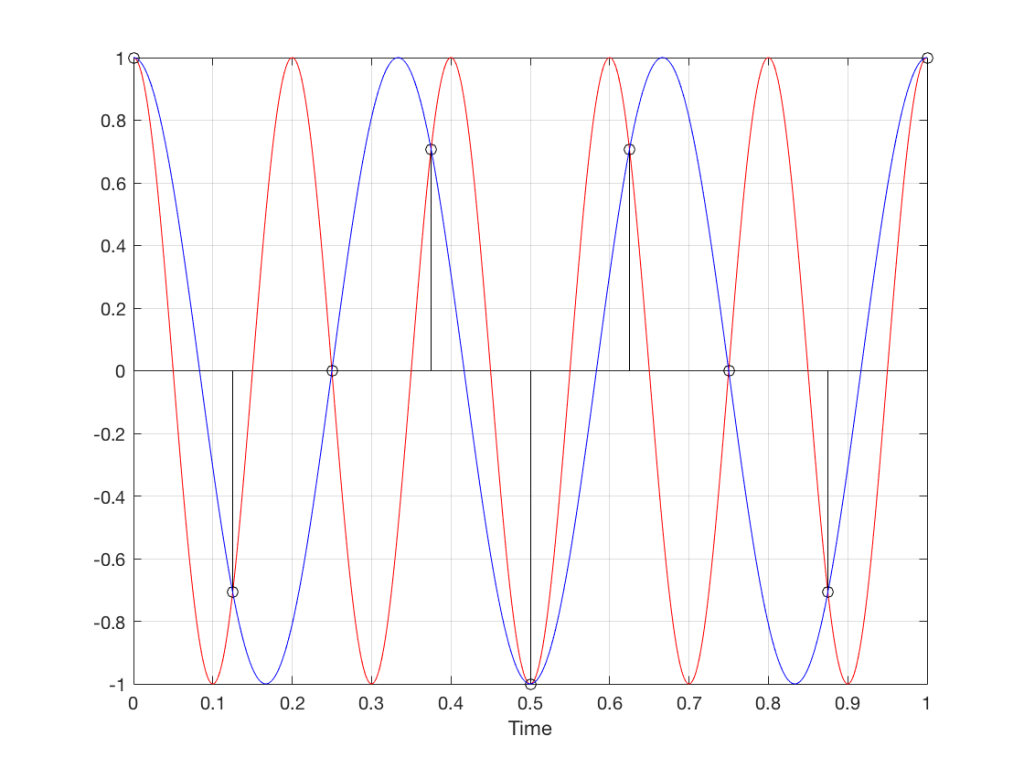Fig 12. The red signal and the black samples of it are the same as was shown in Figure 11. However, another frequency (the blue signal) also fits those samples. So, both the red signal and the blue signal exist in our system.

Moving up in frequency one more step, we get to the right-hand column in Figure 1, whose equivalent, including the aliased signal, are shown in Figure 13.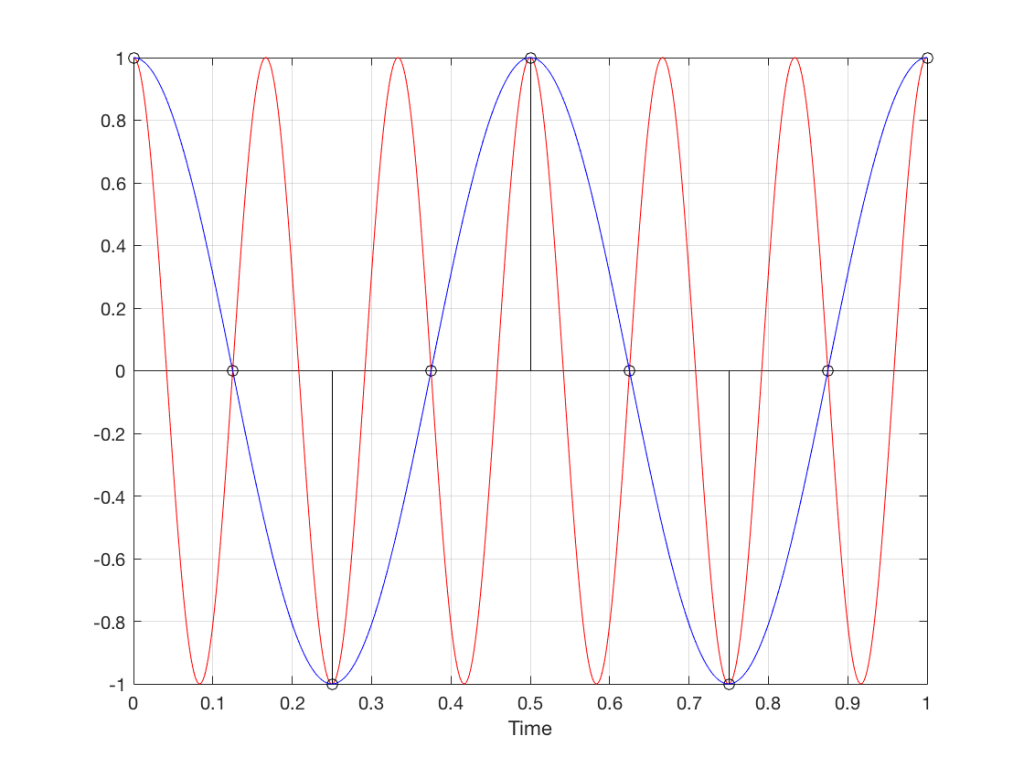Fig 13. A signal (the red curve) that has a frequency equivalent to 280º of rotation per sample, its samples (the black lollipops) and the aliased additional signal that results (the blue curve).

## Do I need to worry yet?

Hopefully, now, you can see that an LPCM system has a limit with respect to the maximum frequency that it can deal with appropriately. Specifically, the signal that you are trying to capture CANNOT exceed one-half of the sampling rate. So, if you are recording a CD, which has a sampling rate of 44,100 samples per second (or 44.1 kHz) then you CANNOT have any audio signals in that system that are higher than 22,050 Hz.

That limit is commonly known as the “Nyquist frequency“, named after Harry Nyquist – one of the persons who figured out that this limit exists.

In theory, this is always true. So, when someone did the recording destined for the CD, they made sure that the signal went through a low-pass filter that eliminated all signals above the Nyquist frequency.

In practice, however, there are many cases where aliasing occurs in digital audio systems because someone wasn’t paying enough attention to what was happening “under the hood” in the signal processing of an audio device. This will come up later.

## Two more details to remember…

There’s an easy way to predict the output of a system that’s suffering from aliasing if your input is sinusoidal (and therefore contains only one frequency). The frequency of the output signal will be the same distance from the Nyquist frequency as the frequency if the input signal. In other words, the Nyquist frequency is like a “mirror” that “reflects” the frequency of the input signal to another frequency below Nyquist.

This can be easily seen in the upper plot of Figure 14. The distance from the Input signal and the Nyquist is the same as the distance between the output signal and the Nyquist.

Also, since that Nyquist frequency acts as a mirror, then the Input and output signal’s frequencies will move in opposite directions (this point will help later).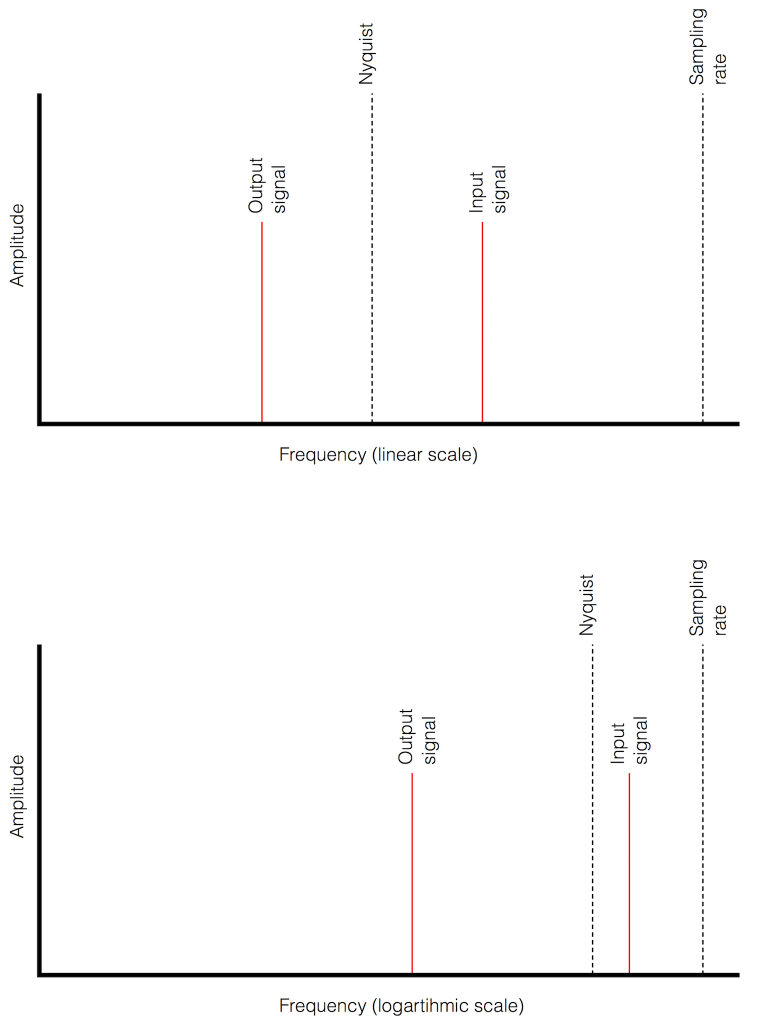Fig 14. Two plots showing the same information about an Input Signal above the Nyquist frequency and the output alias signal. Notice that, in the linear plot on top, it’s easier to see that the Nyquist frequency is the mirror point at the centre of the frequencies of the Input and Output signals.

Usually, frequency-domain plots are done on a logarithmic scale, because this is more intuitive for we humans who hear logarithmically. (For example, we hear two consecutive octaves on a piano as having the same “interval” or “width”. We don’t hear the width of the upper octave as being twice as wide, like a measurement system does. that’s why music notation does not get wider on the top, with a really tall treble clef.) This means that it’s not as obvious that the Nyquist frequency is in the centre of the frequencies of the input signal and its alias below Nyquist.

On to Part 4

# “High-Res” Audio: Part 2 – Resolution

Reminder: This is still just the lead-up to the real topic of this series. However, we have to get some basics out of the way first…

Just like the last posting, this is a copy-and-paste from an article that I wrote for another series. However, this one is important, and rather than just link you to a different page, I’ve reproduced it (with some minor editing to make it fit) here.

Back to Part 1

In the last posting, I talked about digital audio (more accurately, Linear Pulse Code Modulation or LPCM digital audio) is basically just a string of stored measurements of the electrical voltage that is analogous to the audio signal, which is a change in pressure over time…

For now, we’ll say that each measurement is rounded off to the nearest possible “tick” on the ruler that we’re using to measure the voltage. That rounding results in an error. However, (assuming that everything is working correctly) that error can never be bigger than 1/2 of a “step”. Therefore, in order to reduce the amount of error, we need to increase the number of ticks on the ruler.

Now we have to introduce a new word. If we really had a ruler, we could talk about whether the ticks are 1 mm apart – or 1/16″ – or whatever. We talk about the resolution of the ruler in terms of distance between ticks. However, if we are going to be more general, we can talk about the distance between two ticks being one “quantum” – a fancy word for the smallest step size on the ruler.

So, when you’re “rounding off to the nearest value” you are “quantising” the measurement (or “quantizing” it, if you live in Noah Webster’s country and therefore you harbor the belief that wordz should be spelled like they sound – and therefore the world needz more zees). This also means that the amount of error that you get as a result of that “rounding off” is called “quantisation error“.

In some explanations of this problem, you may read that this error is called “quantisation noise”. However, this isn’t always correct. This is because if something is “noise” then is is random, and therefore impossible to predict. However, that’s not strictly the case for quantisation error. If you know the signal, and you know the quantisation values, then you’ll be able to predict exactly what the error will be. So, although that error might sound like noise, technically speaking, it’s not. This can easily be seen in Figures 1 through 3 which demonstrate that the quantisation error causes a periodic, predictable error (and therefore harmonic distortion), not a random error (and therefore noise).

Sidebar: The reason people call it quantisation noise is that, if the signal is complicated (unlike a sine wave) and high in level relative to the quantisation levels – say a recording of Britney Spears, for example – then the distortion that is generated sounds “random-ish”, which causes people to jump to the conclusion that it’s noise.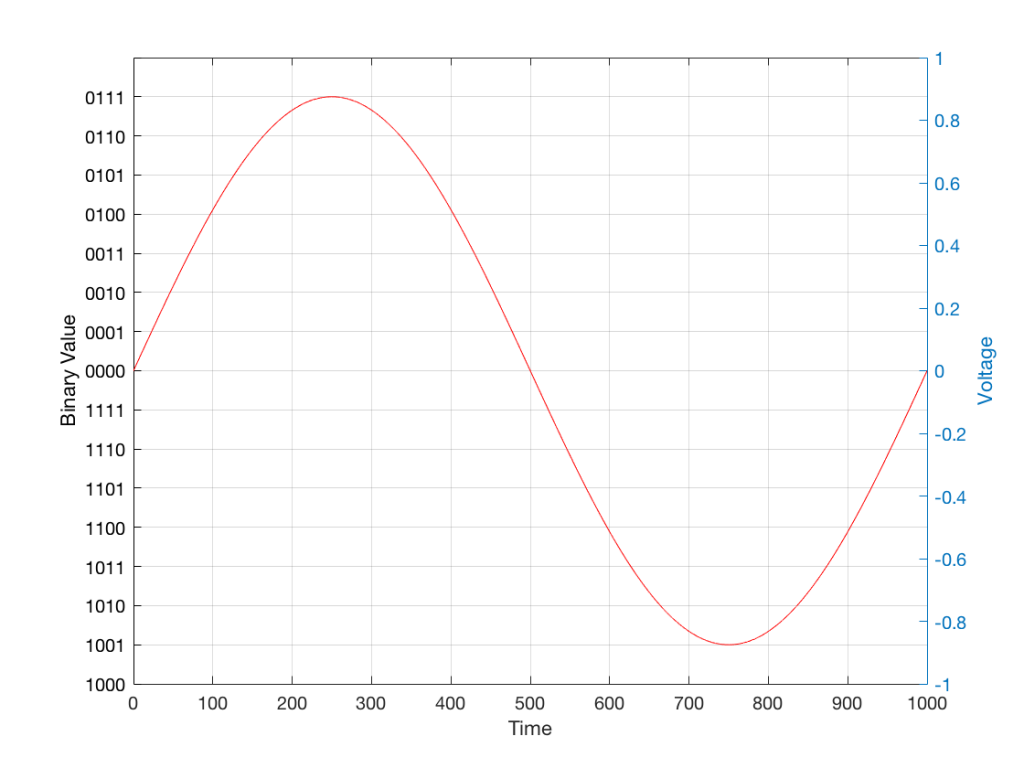Fig 1: The first cycle of a periodic signal (in this case, a sinusoidal waveform) that we are going to quantise using a 4-bit system (notice the 4 bits in the scale on the left).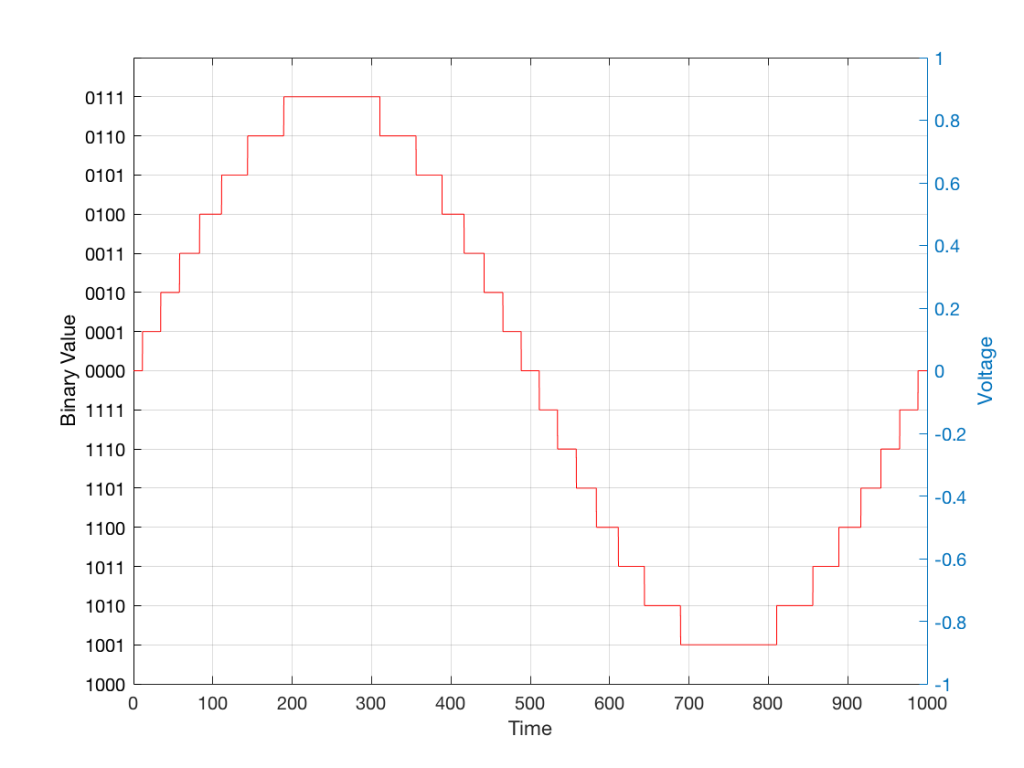Fig 2: The same waveform shown in Figure 1 after quantisation (rounding off) in a 4-bit world.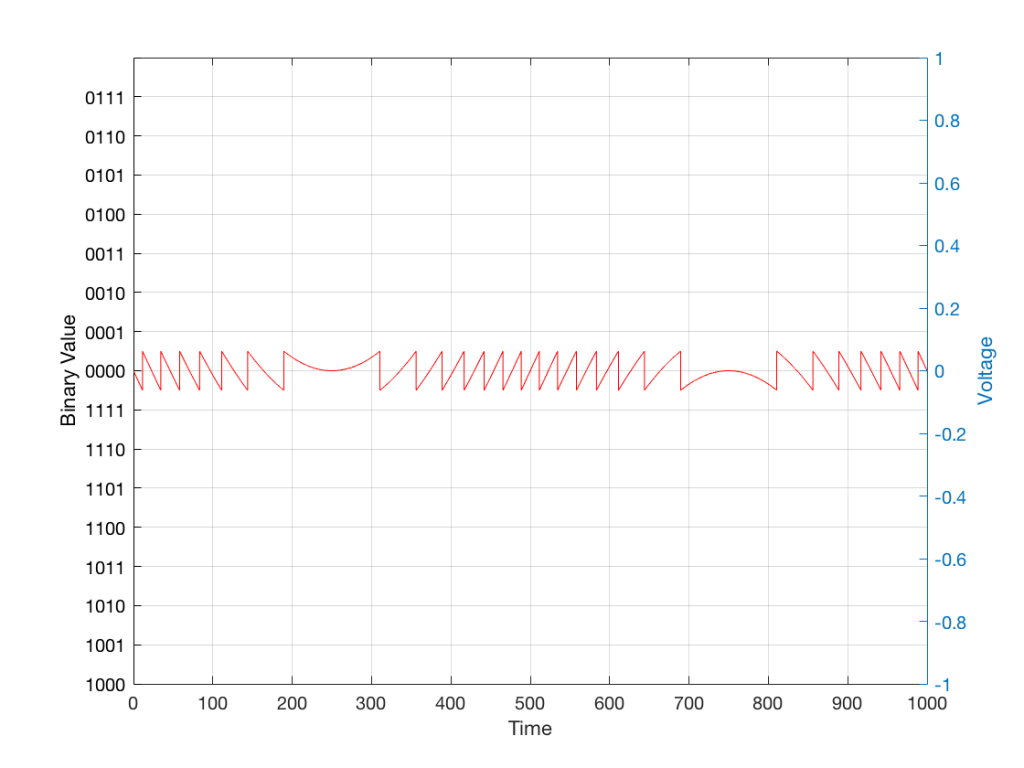Fig 3: The difference between Figure 2 and Figure 1. I made this by subtracting the original signal from the quantised version. This is the error in the quantised waveform – the quantisation error. Notice that it is not noise… it’s completely predictable and it will repeat with repetitions of the signal. Therefore the result of this is distortion, not noise…

Now, let’s talk about perception for a while… We humans are really good at detecting patterns – signals – in an otherwise noisy world. This is just as true with hearing as it is with vision. So, if you have a sound that exists in a truly random background noise, then you can focus on listening to the sound and ignore the noise. For example, if you (like me) are old enough to have used cassette tapes, then you can remember listening to songs with a high background noise (the “tape hiss”) – but it wasn’t too annoying because the hiss was independent of the music, and constant. However, if you, like me, have listened to Bob Marley’s live version of “No Woman No Cry” from the “Legend” album, then you, like me, would miss the the feedback in the PA system at that point in the song when the FoH engineer wasn’t paying enough attention… That noise (the howl of the feedback) is not noise – it’s a signal… Which makes it just as important as the song itself. (I could get into a long boring talk about John Cage at this point, but I’ll try to not get too distracted…)

The problem with the signal in Figure 2 is that the error (shown in Figure 3) is periodic – it’s a signal that demands attention. If the signal that I was sending into the quantisation system (in Figure 1) was a little more complicated than a sine wave – say a sine wave with an amplitude modulation – then the error would be easily “trackable” by anyone who was listening.

So, what we want to do is to quantise the signal (because we’re assuming that we can’t make a better “ruler”) but to make the error random – so it is changed from distortion to noise. We do this by adding noise to the signal before we quantise it. The result of this is that the error will be randomised, and will become independent of the original signal… So, instead of a modulating signal with modulated distortion, we get a modulated signal with constant noise – which is easier for us to ignore. (It has the added benefit of spreading the frequency content of the error over a wide frequency band, rather than being stuck on the harmonics of the original signal… but let’s not talk about that…)

## For example…

Let’s take a look at an example of this from an equivalent world – digital photography.

The photo in Figure 4 is a black and white photo – which actually means that it’s comprised of shades of gray ranging from black all the way to white. The photo has 272,640 individual pixels (because it’s 640 pixels wide and 426 pixels high). Each of those pixels is some shade of gray, but that shading does not have an infinite resolution. There are “only” 256 possible shades of gray available for each pixel.

So, each pixel has a number that can range from 0 (black) up to 255 (white).Fig 4: A photo of a building in Paris. Each pixel in this photo has one of 256 possible levels of gray – from white (255) down to black (0).

If we were to zoom in to the top left corner of the photo and look at the values of the 64 pixels there (an 8×8 pixel square), you’d see that they are:

86 86 90 88 87 87 90 91
86 88 90 90 89 87 90 91
88 89 91 90 89 89 90 94
88 90 91 93 90 90 93 94
89 93 94 94 91 93 94 96
90 93 94 95 94 91 95 96
93 94 97 95 94 95 96 97
93 94 97 97 96 94 97 97

What if we were to reduce the available resolution so that there were fewer shades of gray between white and black? We can take the photo in Figure 1 and round the value in each pixel to the new value. For example, Figure 5 shows an example of the same photo reduced to only 6 levels of gray.Fig 5: The same photo of the same building. Each pixel in this photo has one of 6 possible levels of gray. Notice that some details are lost – like the smooth transitions in the clouds, or the stripes in the marble in the pillars.

Now, if we look at those same pixels in the upper left corner, we’d see that their values are

102 102 102 102 102 102 102 102
102 102 102 102 102 102 102 102
102 102 102 102 102 102 102 102
102 102 102 102 102 102 102 102
102 102 102 102 102 102 102 102
102 102 102 102 102 102 102 102
102 102 102 102 102 102 102 102
102 102 102 102 102 102 102 102

They’ve all been quantised to the nearest available level, which is 102. (Our possible values are restricted to 0, 51, 102, 154, 205, and 255).

So, we can see that, by quantising the gray levels from 256 possible values down to only 6, we lose details in the photo. This should not be a surprise… That loss of detail means that, for example, the gentle transition from lighter to darker gray in the sky in the original is “flattened” to a light spot in a darker background, with a jagged edge at the transition between the two. Also, the details of the wall pillars between the windows are lost.

If we take our original photo and add noise to it – so were adding a random value to the value of each pixel in the original photo (I won’t talk about the range of those random values…) it will look like Figure 6. This photo has all 256 possible values of gray – the same as in Figure 1.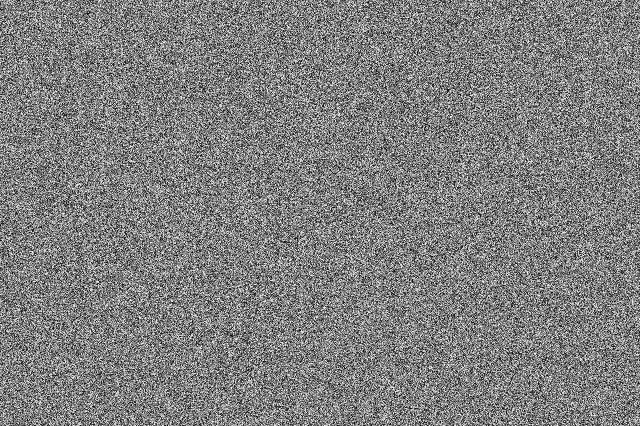Fig 6: A photo of noise with the same width and height as the original photo, with random values (ranging from 0 to 255) in each pixel.

If we then quantise Figure 6 using our 6 possible values of gray, we get Figure 7. Notice that, although we do not have more grays than in Figure 5, we can see things like the gradual shading in the sky and some details in the walls between the tall windows.Fig 7: The same photo of the same building in Figure 4. Each pixel in this photo ALSO only has one of 6 possible levels of gray – just like in Figure 5. However, this version is the result of quantising the original photo with the noise added before quantisation. The result is admittedly noisy – but we are able to see pattens in the noise that preserve some of the details that we lost in Figure 5.

That noise that we add to the original signal is called dither – because it is forcing the quantiser to be indecisive about which level to quantise to choose.

I should be clear here and say that dither does not eliminate quantisation error. The purpose of dither is to randomise the error, turning the quantisation error into noise instead of distortion. This makes it (among other things) independent of the signal that you’re listening to, so it’s easier for your brain to separate it from the music, and ignore it.

## Addendum: Binary basics and SNR

We normally write down our numbers using a “base 10” notation. So, when I write down 9374 – I mean
9 x 1000 + 3 x 100 + 7 x 10 + 4 x 1
or
9 x 103 + 3 x 102 + 7 x 101 + 4 x 100

We use base 10 notation – a system based on 10 digits (0 through 9) because we have 10 fingers.

If we only had 2 fingers, we would do things differently… We would only have 2 digits (0 and 1) and we would write down numbers like this:
11101

which would be the same as saying
1 x 16 + 1 x 8 + 1 x 4 + 0 x 2 + 1 x 1
or
1 x 24 + 1 x 23 + 1 x 22 + 0 x 21 + 1 x 20

The details of this are not important – but one small point is. If we’re using a base-10 system and we increase the number by one more digit – say, going from a 3-digit number to a 4-digit number, then we increase the possible number of values we can represent by a factor of 10. (in other words, there are 10 times as many possible values in the number XXXX than in XXX.)

If we’re using a base-2 system and we increase by one extra digit, we increase the number of possible values by a factor of 2. So XXXX has 2 times as many possible values as XXX.

Now, remember that the error that we generate when we quantise is no bigger than 1/2 of a quantisation step, regardless of the number of steps. So, if we double the number of steps (by adding an extra binary digit or bit to the value that we’re storing), then the signal can be twice as “far away” from the quantisation error.

This means that, by adding an extra bit to the stored value, we increase the potential signal-to-error ratio of our LPCM system by a factor of 2 – or 6.02 dB.

So, if we have a 16-bit LPCM signal, then a sine wave at the maximum level that it can be without clipping is about 6 dB/bit * 16 bits – 3 dB = 93 dB louder than the error. The reason we subtract the 3 dB from the value is that the error is +/- 0.5 of a quantisation step (normally called an “LSB” or “Least Significant Bit”).

Note as well that this calculation is just a rule of thumb. It is neither precise nor accurate, since the details of exactly what kind of error we have will have a minor effect on the actual number. However, it will be close enough.

On to Part 3.

# “High-Res” Audio: Part 1

I’ve been debating writing a series of postings about “high resolution” audio for a long time – years. Lately, (probably because of some hype generated by some recent press releases) I’ve been getting lots of question (no, that’s not a typo) about it, so it appears the time has come…

To start: the question that I get (a lot) is “If I can’t hear above 20 kHz, then what’s the use of high-res?” As I’ll explain as we go through, this is only one, rather small aspect to consider in this topic. In fact, it might be the least important issue to consider.

However, before I write too much, I’ll say that I’m not going to argue for or against higher resolutions in digital audio systems. I’m only going to go through a bunch of issues that can be used to argue either for or against them. So, there’s not going to be a big reveal at the end of this series telling you that high-res is either better, worse, or no different than whatever you’re using now. It’s merely going to be a discussion of a number of issues that need to be weighed. The problem is that this entire topic is complicated – and there’s no single “right” answer, as I’ll argue as we go along.

To start, let’s get down to basics and look (once again, from the perspectives of this website) at what sound is, and how it’s converted from an analogue electrical signal into a digital representation. The good thing is that I’ve written this introduction before in a different series of postings. So, I’m going to be extremely lazy and just copy-and-paste that information here. I’m not just referring you to another page because I’m intentionally leaving some things out because we’re headed into having a different discussion this time.

## A quick introduction to sound

At the simplest level, sound can be described as a small change in air pressure (or barometric pressure) over short periods of time. If you’d like to have a better and more edu-tain-y version of this statement with animations and pretty colours, you could take 10 minutes to watch this video, for example.

That change in pressure can be “captured” by using a microphone, that is (at the simplest level) a device that has a change in air pressure at its input and a change in electrical voltage at its output. Ignoring a lot of details, we could say that if you were to plot a measurement of the air pressure (at the input of the microphone) over time, and you were to compare it to a plot of the measurement of the voltage (at the output of the microphone) over time, you would see the same curve on the two graphs. This means that the change in voltage is analogous to the change in air pressure.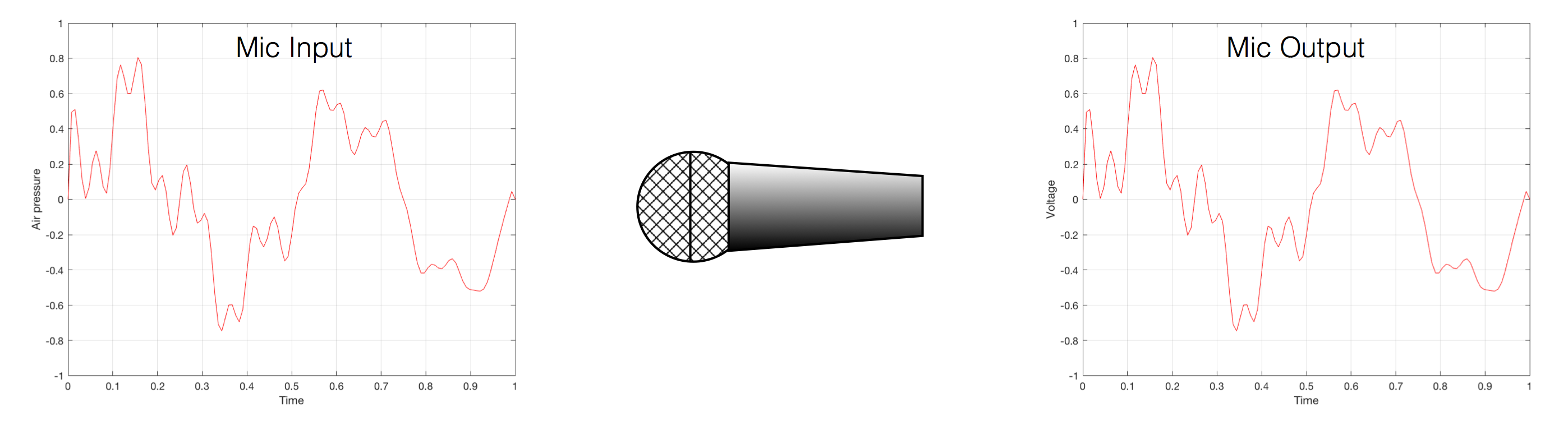Fig 1. Notice that (in theory, and ignoring a lot of things…) the change in air pressure over time at the input of the microphone is identical to the change in voltage over time at its output. Of course, this is not true in real life – microphones lie like a cheap rug…

At this point in the conversation, I’ll make a point to say that, in theory, we could “zoom in” on either of those two curves shown in Figure 1 and see more and more details. This is like looking at a map of Canada – it has lots of crinkly, jagged lines. If you zoom in and look at  the map of Newfoundland and Labrador, you’ll see that it has finer, crinkly, jagged lines. If you zoom in further, and stand where the water meets the shore in Trepassey and take a photo of your feet, you could copy it to draw a map of the line of where the water comes in around the rocks – and your toes – and you would wind up with even finer, crinkly, jagged lines… You could take this even further and get down to a microscopic or molecular level – but you get the idea… The point is that, in theory, both of the plots in Figure 1 have infinite resolution, both in time and in air pressure or voltage.

Now, let’s say that you wanted to take that microphone’s output and transmit it through a bunch of devices and wires that, in theory, all do nothing to the signal. Let’s say, for example, that you take the mic’s output, send it through a wire to a box that makes the signal twice as loud. Then take the output of that box and send it through a wire to another box that makes it half as loud. You take the output of that box and send it through a wire to a measuring device. What will you see? Unfortunately, none of the wires or boxes in the chain can be perfect, so you’ll probably see the signal plus something else which we’ll call the “error” in the system’s output. We can call it the error because, if we measure the input voltage and the output voltage at any one instant, we’ll probably see that they’re not identical. Since they should be identical, then the system must be making a mistake in transmitting the signal – so it makes errors…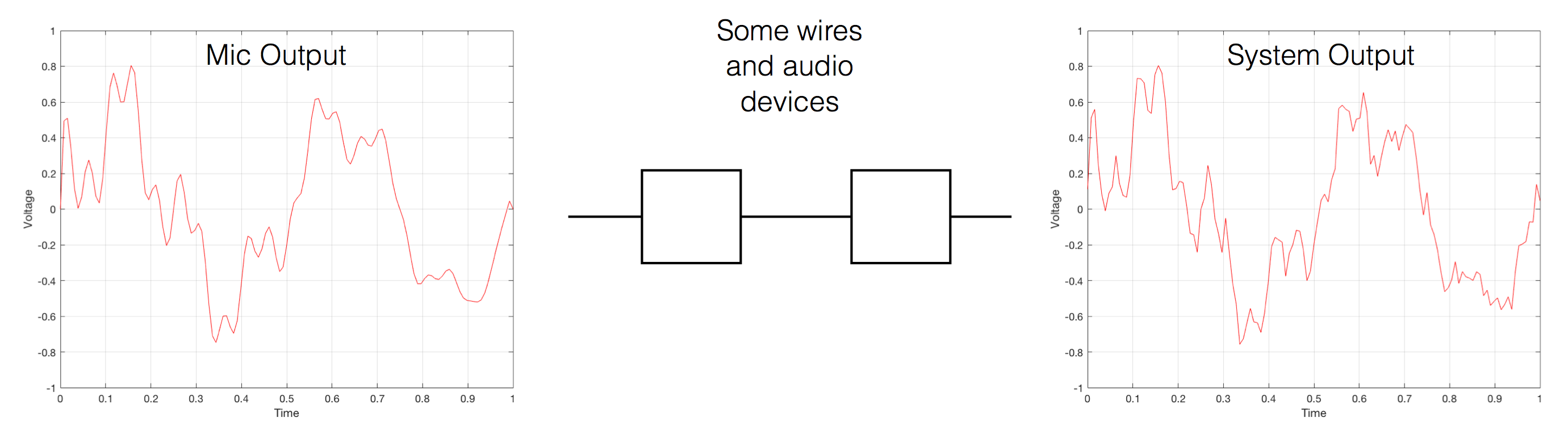Fig 2. If you send an audio signal through some wires and devices that (in theory) do nothing to the signal, you’ll find out that they add some extra stuff that you don’t want.

Pedantic Sidebar: Some people will call that error that the system adds to the signal “noise” – but I’m not going to call it that. This is because “noise” is a specific thing – noise is random – so if it’s not random, it’s not noise. Also, although the signal has been distorted (in that the output of the system is not identical to the input) I won’t call it “distortion” either, since distortion is a name that’s given to something that happens to the signal because the signal is there. (We would probably get at least some of the error out of our system even if we didn’t send any audio into it.) So, we could be slightly geeky and adequately vague and call the extra stuff “Distortion plus noise” but not “THD+N” – which stands for “Total Harmonic Distortion Plus Noise” – because not all kinds of distortion will produce a harmonic of the signal… but I’m getting ahead of myself…

So, we want to transmit (or store) the audio signal – but we want to reduce the noise caused by the transmission (or storage) system. One way to do this is to spend more money on your system. Use wires with better shielding, amplifiers with lower noise floors, bigger power supplies so that you don’t come close to their limits, run your magnetic tape twice as fast, and so on and so on. Or, you could convert the analogue signal (remember that it’s analogous to the change in air pressure over time) to one that is represented (and therefore transmitted or stored) digitally instead.

What does this mean?

## Conversion from analogue to digital and back(but skipping important details)

In the example above, we made a varying voltage that was analogous to the varying air pressure. If we wanted to store this, we could do it by varying the amount of magnetism on a wire or a coating on a tape, for example. Or we could cut a wiggly groove in a bit of vinyl that has a similar shape to the curve in the plots in Figure 1. Or, we could do something else: we could get a metronome (or a clock) and make a measurement of the voltage every time the metronome clicks, and write down the measurements.

For example, let’s zoom in on the first little bit of the signal in the plots in Figure 1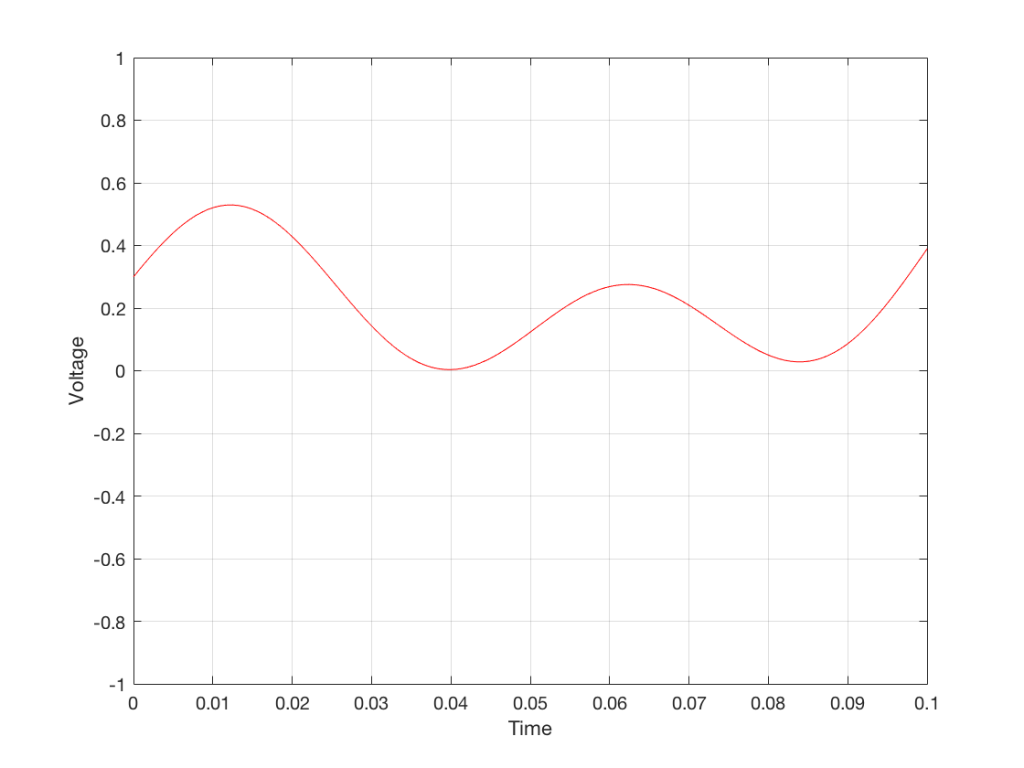Fig. 3 The same curve as was shown in Figure 1 – but zoomed in to the very beginning.

We’ll then put on a metronome and make a measurement of the voltage every time we hear the metronome click…

We can then keep the measurements (remembering how often we made them…) and write them down like this:

0.3000
0.4950
0.5089
0.3351
0.1116
0.0043
0.0678
0.2081
0.2754
0.2042
0.0730
0.0345
0.1775

We can store this series of numbers on a computer’s hard disk, for example. We can then come back tomorrow, and convert the measurements to voltages. First we read the measurements, and create the appropriate voltage…

We then make a “staircase” waveform by “holding” those voltages until the next value comes in.

All we need to do then is to use a low-pass filter to smooth out the hard edges of the staircase.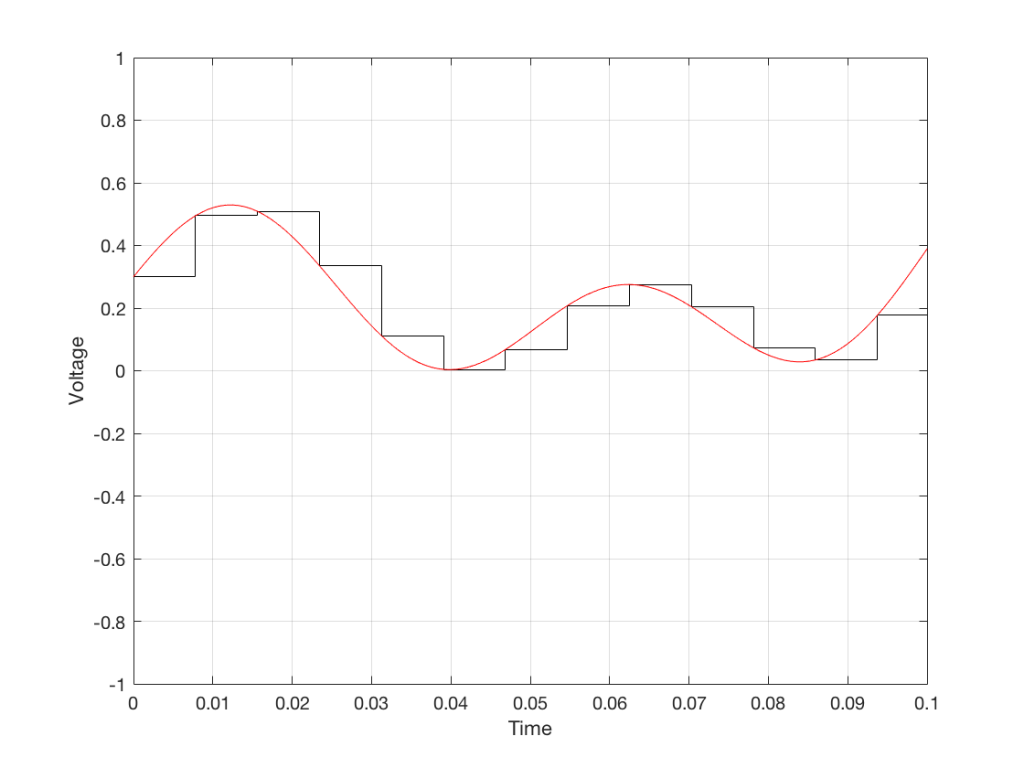Fig 7. When we smooth out the staircase, we get back the original signal (in red).

So, in this example, we’ve gone from an analogue signal (the red curve in Figure 3) to a digital signal (the series of numbers), and back to an analogue signal (the red curve in Figure 7).

In some ways, this is a bit like the way a movie works. When you watch a movie, you see a series of still photographs, probably taken at a rate of 24 pictures (or frames) per second. If you play those photos back at the same rate (24 fps or frames per second), you think you see movement. However, this is because your eyes and brain aren’t fast enough to see 24 individual photos per second – so you are fooled into thinking that things on the screen are moving.

However, digital audio is slightly different from film in two ways:

• The sound (equivalent to the movement in the film) is actually happening. It’s not a trick that relies on your ears and brain being too slow.
• If, when you were filming the movie, something were to happen between frames (say, the flash of a gunshot, for example) then it would never be caught on film. This is because the photos are discrete moments in time – and what happens between them is lost. However, if something were to make a very, very short sound between two samples (two measurements) in the digital audio signal – it would not be lost. This is because of something that happens at the beginning of the chain that I haven’t described… yet…

However, there are some “artefacts” (a fancy term for “weird errors”) that are present both in film and in digital audio that we should talk about.

The first is an error that happens when you mess around with the rate at which you take the measurements (called the “sampling rate”) or the photos (called the “frame rate”) – and, more importantly, when you need to worry about this. Let’s say that you make a film at 24 fps. If you play this back at a higher frame rate, then things will move very quickly (like old-fashioned baseball movies…). If you play them back at a lower frame rate, then things move in slow motion. So, for things to look “normal” you have to play the movie at the same rate that it was filmed. However, as long as no one is looking, you can transfer the movie as fast as you like. For example, if you wanted to copy the film, you could set up a movie camera so it was pointing at a movie screen and film the film. As long as the movie on the screen is running in sync with the camera, you can do this at any frame rate you like. But you’ll have to watch the copy at the same frame rate as the original film… (Note that this issue is not something that will come up in this series of postings about high resolution audio)

The second is an easy artefact to recognise. If you see a car accelerating from 0 to something fast on film, you’ll see the wheels of the car start to get faster and faster, then, as the car gets faster, the wheels slow down, stop, and then start going backwards… This does not happen in real life (unless you’re in a place lit with flashing lights like fluorescent bulbs or LED’s). I’ll do a posting explaining why this happens – but the thing to remember here is that the speed of the wheel rotation that you see on the film (the one that’s actually captured by the filming…) is not the real rotational speed of the wheel. However, those two rotational speeds are related to each other (and to the frame rate of the film). If you change the real rotational rate or the frame rate, you’ll change the rotational rate in the film. So, we call this effect “aliasing” because it’s a false version (an alias) of the real thing – but it’s always the same alias (assuming you repeat the conditions…) Digital audio can also suffer from aliasing, but in this case, you put in one frequency (which is actually the same as a rotational speed) and you get out another one. This is not the same as harmonic distortion, since the frequency that you get out is due to a relationship between the original frequency and the sampling rate, so the result is almost never a multiple of the input frequency. (We’re going to dig into this a lot deeper through this series of postings about high resolution audio, so if it doesn’t immediately make sense, don’t worry…)

## Some important details that I left out…

One of the things I said above was something like “we measure the voltage and store the results” and the example I gave was a nice series of numbers that only had 4 digits after the decimal point. This statement has some implications that we need to discuss.

Let’s say that I have a thing that I need to measure. For example, Figure 8 shows a piece of metal, and I want to measure its width.

Using my ruler, I can see that this piece of metal is about 57 mm wide. However, if I were geeky (and I am) I would say that this is not precise enough – and therefore it’s not accurate. The problem is that my ruler is only graduated in millimetres. So, if I try to measure anything that is not exactly an integer number of mm long, I’ll either have to guess (and be wrong) or round the measurement to the nearest millimetre (and be wrong).

So, if I wanted you to make a piece of metal the same width as my piece of metal, and I used the ruler in Figure 8, we would probably wind up with metal pieces of two different widths. In order to make this better, we need a better ruler – like the one in Figure 9.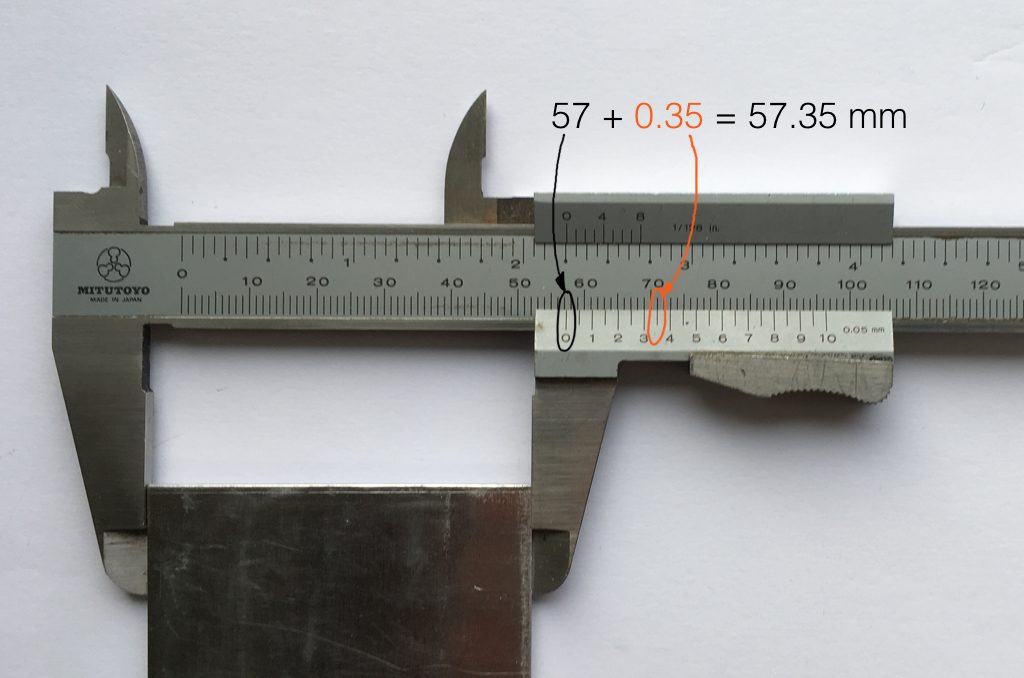Fig 9. The same piece of metal being measured with a vernier caliper. This gives us additional precision (down to 0.05 mm) so we can make a more accurate measurement.

Figure 9 shows a vernier caliper (a fancy type of ruler) being used to measure the same piece of metal. The caliper has a resolution of 0.05 mm instead of the 1 mm available on the ruler in Figure 8. So, we can make a much more accurate measurement of the metal because we have a measuring device with a higher precision.

The conversion of a digital audio signal is the same. As I said above, we measure the voltage of the electrical signal, and transmit (or store) the measurement. The question is: how accurate and precise is your measurement? As we saw above, this is (partly) determined by how many digits are in the number that you use when you “write down” the measurement.

Since the voltage measurements in digital audio are recorded in binary rather than decimal (we use 0 and 1 to write down the number instead of 0 up to 9) then we use Binary digITS – or “bits” instead of decimal digits (which are not called “dits”). The number of bits we have in the number that we write down (partly) determines the precision of the measurement of the voltage – and therefore (possibly), our accuracy…

Just like the example of the ruler in Figure 8, above, we have a limited resolution in our measurement. For example, if we had only 4 bits to work with then the waveform in 4 – the one we have to measure – would be measured with the “ruler” shown on the left side of Figure 10, below.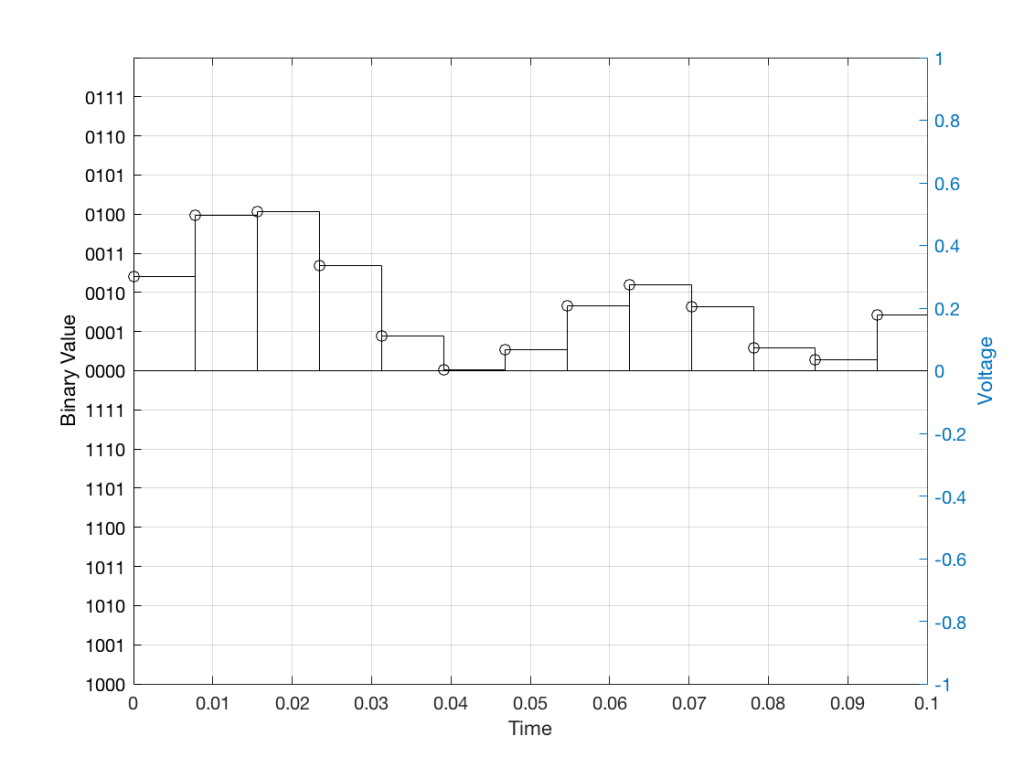Fig 10: The waveform from Figure 4 as a voltage (notice the Y-axis on the right). We have to measure these values using the ruler with the resolution shown on the Y-axis on the left.

When we do this, we have to round off the value to the nearest “tick” on our ruler, as shown in Figure 11.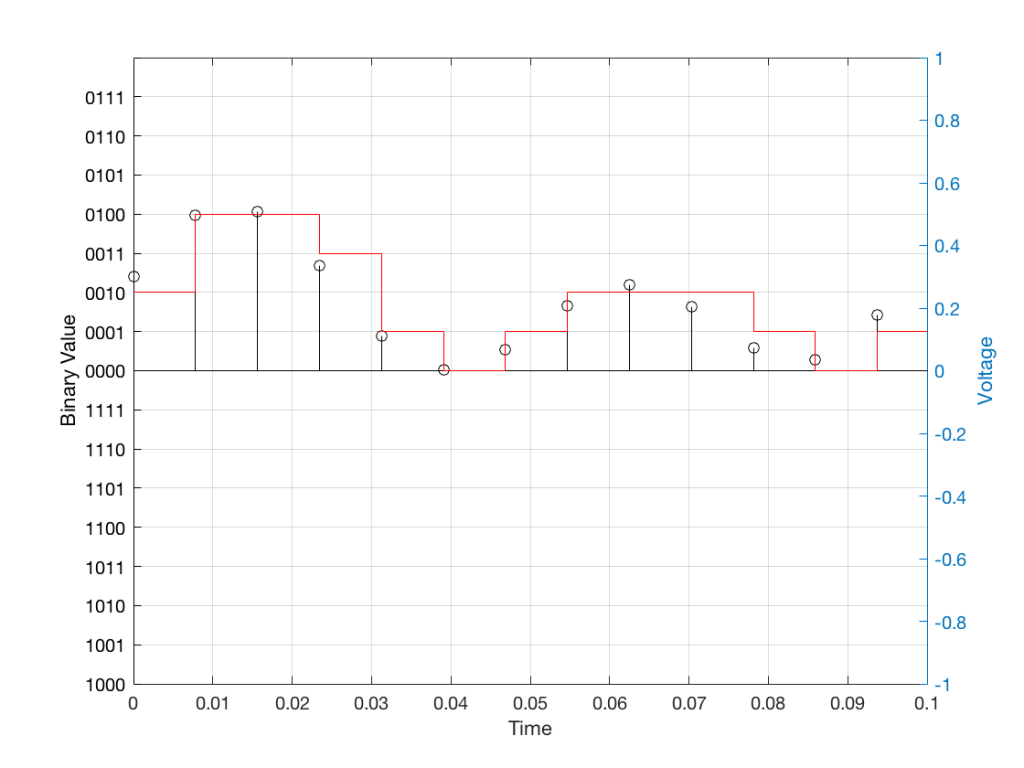Fig 11: The values from figure 10 (shown as the circles) rounded off to the nearest value on our 4-bit ruler (the red staircase).

Using this “ruler” which gives a write-down-able “quantity” to the measurement, we get the following values for the red staircase:

0010
0100
0100
0011
0001
0000
0001
0010
0010
0010
0001
0000
0001

When we “play these back” we get the staircase again, shown in Figure 12.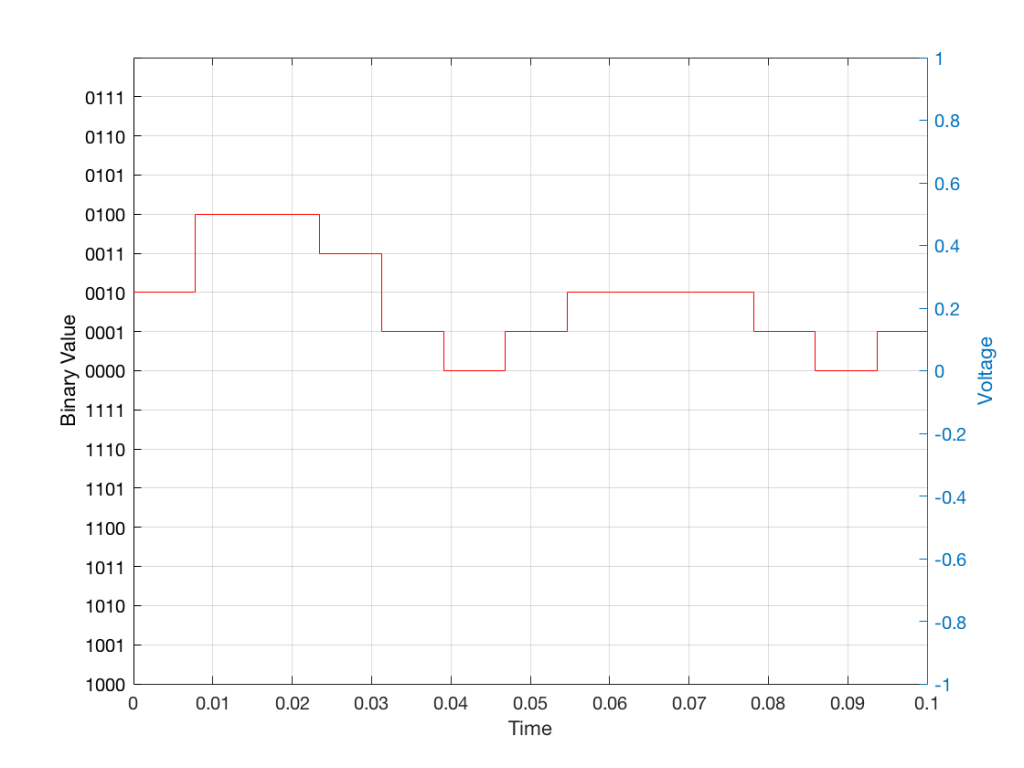Fig 12: The output of the measurements. Notice that all values sit exactly on one of the values for the “ruler” on the left Y-axis of the plot.

Of course, this means that, by rounding off the values, we have introduced an error in the system (just like the measurement in Figure 8 has a bigger error than the one in Figure 9). We can calculate this error if we just subtract the original signal from the output signal (in other words, Figure 12 minus Figure 10) to get Figure 13.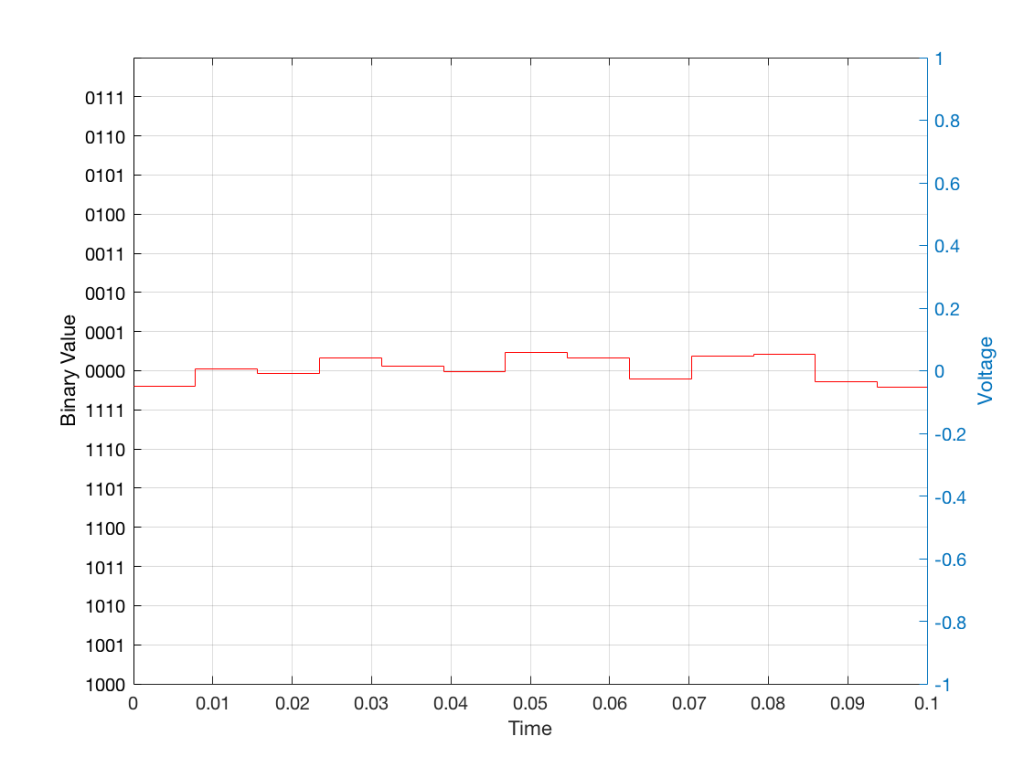Fig 13: The error that we produced due to the rounding off of the signal when we did the measurements. Notice that the error is always less than 0.5 of a “tick” of the ruler on the left Y-axis.

In order to improve our accuracy of the measurement, we have to increase the precision of the values. We can do this by adding an extra digit (or bit) to the number that we use to record the value.

If we were using decimal numbers (0-9) then adding an extra digit to the number would give us 10 times as many possibilities. (For example, if we were using 4 digits after the decimal in the example at the start of this posting, we have a total of 10,000 possible values – 0.0000 to 0.9999. If we add one more digit, we increase the resolution to 100,000 possible values – 0.00000 to 0.99999 ).

In binary, adding one extra digit gives us twice as many “ticks” on the ruler. So, using 4 bits gives us 16 possible values. Increasing to 5 bits gives us 32 possible values.

If you’re listening to a CD, then the individual measurements of each voltage – the “sample values” – are stored with 16 bits, which means that we have 65,536 possible values to pick from.

Remember that this means that we have more “ticks” on our ruler – but we don’t necessarily increase its range. So, for example, we’re still measuring a voltage from -1 V to 1 V – we just have more and more resolution with which we can do that measurement.

On to Part 2…

# Turn it down half-way…

#81 in a series of articles about the technology behind Bang & Olufsen loudspeakers

Bertrand Russell once said, “In all affairs it’s a healthy thing now and then to hang a question mark on the things you have long taken for granted.”

This article is a discussion, both philosophical and technical about what a volume control is, and what can be expected of it. This seems like a rather banal topic, but I find it surprising how often I’m required to explain it.

## Why am I writing this?

I often get questions from colleagues and customers that sound something like the following:

• Why does my Beovision television’s volume control only go to 90%? Why can’t I go to 100%?
• I set the volume on my TV to 40, so why is it so quiet (or loud)?

The first question comes from people who think that the number on the screen is in percent – but it’s not. The speedometer in your car displays your speed in kilometres per hour (km/h), the tachometer is in revolutions of the engine per minute (RPM) the temperature on your thermostat is in degrees Celsius (ºC), and the display on your Beovision television is on a scale based on decibels (dB). None of these things are in percent (imagine if the speed limit on the highway was 80% of your car’s maximum speed… we’d have more accidents…)

The short reason we use decibels instead of percent is that it means that we can use subtraction instead of division – which is harder to do. The shortcut rule-of-thumb to remember is that, every time you drop by 6 dB on the volume control, you drop by 50% of the output. So, for example, going from Volume step 90 to Volume step 84 is going from 100% to 50%. If I keep going down, then the table of equivalents looks like this: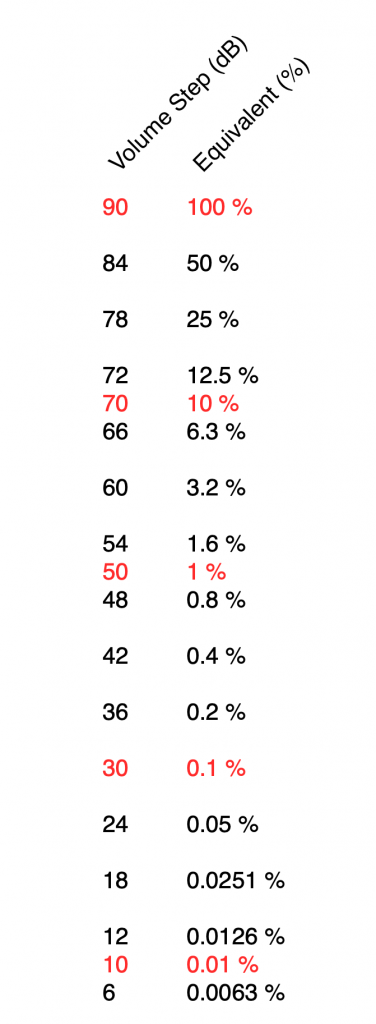Volume steps on a Beovision Eclipse, Beovision 11, etc… and the equivalent displayed as a percentage.

I’ve used two colours there to illustrate two things:

• Every time you drop by 6 volume steps, you cut the percentage in half. For example, 60 is five drops of 6 steps, which is 1/2 of 1/2 of 1/2 of 1/2 of 1/2 of 100%, or 3.2% (notice the five halves there…)
• Every time you drop by 20, you cut the percentage to 1/10. So, Volume Step 50 is 1% of Volume Step 90 because it’s two drops of 20 on the volume control.

If I graph this, showing the percentage equivalent of all 91 volume steps (from 0 to 90) then it looks like this:

Of course, the problem this plot is that everything from about Volume Step 40 and lower looks like 0% because the plot doesn’t have enough detail. But I can fix that by changing the way the vertical axis is displayed, as shown below.

That plot shows exactly the same information. The only difference is that the vertical scale is no longer linearly counting from 0% to 100% in equal steps.

Why do we (and every other audio company) do it this way? The simple reason is that we want to make a volume slider (or knob) where an equal distance (or rotation) corresponds to an equal change in output level. We humans don’t perceive things like change in level in percent – so it doesn’t make sense to use a percent scale.

For the longer explanation, read on…

## Basic concepts

We need to start at the very beginning, so here goes:

### Volume control and gain

1. An audio signal is (at least in a digital audio world) just a long list of numbers for each audio channel.
2. The level of the audio signal can be changed by multiplying it by a number (called the gain).
1. If you multiply by a value larger than 1, the audio signal gets louder.
2. If you multiply by a number between 0 and 1, the audio signal gets quieter.
3. If you multiply by zero, you mute the audio signal.
3. Therefore, at its simplest core, a volume control implemented in a digital audio system is a multiplication by a gain. You turn up the volume, the gain value increases, and the audio is multiplied by a bigger number producing a bigger result.

That’s the first thing. Now we move on to how we perceive things…

### Perception of Level

Speaking very generally, our senses (that we use to perceive the world around us) scale logarithmically instead of linearly. What does this mean? Let’s take an example:

Let’s say that you have \$100 in your bank account. If I then told you that you’d won \$100, you’d probably be pretty excited about it.

However, if you have \$1,000,000 in your bank account, and I told you that you’re won \$100, you probably wouldn’t even bother to collect your prize.

This can be seen as strange; the second \$100 prize is not less money than the first \$100 prize. However, it’s perceived to be very different.

If, instead of being \$100, the cash prize were “equal to whatever you have in your bank account” – so the person with \$100 gets \$100 and the person with \$1,000,000 gets \$1,000,000, then they would both be equally excited.

The way we perceive audio signals is similar. Let’s say that you are listening to a song by Metallica at some level, and I ask you to turn it down, and you do. Then I ask you to turn it down by the same amount again, and you do. Then I ask you to turn it down by the same amount again, and you do… If I were to measure what just happened to the gain value, what would I find?

Well, let’s say that, the first time, you dropped the gain to 70% of the original level, so (for example) you went from multiplying the audio signal by 1 to multiplying the audio signal by 0.7 (a reduction of 0.3, if we were subtracting, which we’re not). The second time, you would drop by the same amount – which is 70% of that – so from 0.7 to 0.49 (notice that you did not subtract 0.3 to get to 0.4). The third time, you would drop from 0.49 to 0.343. (not subtracting 0.3 from 0.4 to get to 0.1).

In other words, each time you change the volume level by the “same amount”, you’re doing a multiplication in your head (although you don’t know it) – in this example, by 0.7. The important thing to note here is that you are NOT subtracting 0.3 from the gain in each of the above steps – you’re multiplying by 0.7 each time.

What happens if I were to express the above as percentages? Then our volume steps (and some additional ones) would look like this:

100%
70%
49%
34%
24%
17%
12%
8%

Notice that there is a different “distance” between each of those steps if we’re looking at it linearly (if we’re just subtracting adjacent values to find the difference between them). However, each of those steps is a reduction to 70% of the previous value.

This is a case where the numbers (as I’ve expressed them there) don’t match our experience. We hear each reduction in level as the same as the other steps, but they don’t look like they’re the same step size when we write them all down the way I’ve done above. (In other words, the numerical “distance” between 100 and 70 is not the same as the numerical “distance” between 49 and 34, but these steps would sound like the same difference in audio level.)

SIDEBAR: This is very similar / identical to the way we hear and express frequency changes. For example, the figure below shows a musical staff. The red brackets on the left show 3 spacings of one octave each; the distance between each of the marked frequencies sound the same to us. However, as you can see by the frequency indications, each of those octaves has a very different “width” in terms of frequency. Seen another way, the distance in Hertz in the octave from 440 Hz to 880 Hz is equal to the distance from 440 Hz all the way down to 0 Hz (both have a width of 440 Hz). However, to us, these sound like very different intervals.

SIDEBAR to the SIDEBAR: This also means that the distance in Hertz covered by the top octave on a piano is larger than the the distance covered by all of the other keys.

SIDEBAR to the SIDEBAR to the SIDEBAR: This also means that changing your sampling rate from 48 kHz to 96 kHz doubles your bandwidth, but only gives you an extra octave. However, this is not an argument against high-resolution audio, since the frequency range of the output is a small part of the list of pro’s and con’s.)

This is why people who deal with audio don’t use percent – ever. Instead, we use an extra bit of math that uses an evil concept called a logarithm to help to make things make more sense.

### What is a logarithm?

If I say the following, you should not raise your eyebrows:

2*3 = 6, therefore 6/2 = 3 and 6/3 = 2

In other words, division is just multiplication done backwards. This can be generalised to the following:

if a*b=c, then c/a=b and c/b=a

Logarithms are similar; they’re just exponents done backwards. For example:

102 = 100, therefore Log10(100) = 2

and generally:

AB=C, therefore LogA(C) = B

### Why use a logarithm?

The nice thing about logarithms is that they are a convenient way for a mathematician to do addition instead of multiplication.

For example, if I have the following sequence of numbers:

2, 4, 8, 16, 32, 64, and so on…

It’s easy to see that I’m just multiplying by 2 to get the next number.

What if I were to express the number sequence above as a series of exponents? Then it would look like this:

21, 22, 23, 24, 25, 26

Not useful yet…

What if I asked you to multiply two numbers in that sequence? Say, for example, 1024 * 8192. This would take some work (or at least some scrambling, looking for the calculator app on your phone…). However, it helps to know that this is the same as asking you to multiply 210 * 213 – to which the answer is 223. Notice that 23 is merely 10+13. So, I’ve used exponents to convert the problem from multiplication (1024*8192) to addition (210 * 213 = 2(10+13)).

How did I find out that 8192 = 213? By using a logarithm : Log2(8192) = 13.

In the old days, you would have been given a book of logarithmic tables in school, which was a way of looking up the logarithm of 8192. (Actually, those books were in base 10 and not base 2, so you would have found out that Log10(8192) = 3.9013, which would have made this discussion more confusing…) Nowadays, you can use an antique device called a “calculator” – a simulacrum of which is probably on a device you call a “phone” but is rarely used as such.

I will leave it to the reader to figure out why this quality of logarithms (that they convert multiplication into addition) is why slide rules work.

### So what?

Let’s go back to the problem: We want to make a volume slider (or knob) where an equal distance (or rotation) corresponds to an equal change in level. Let’s do a simple one that has 10 steps. Coming down from “maximum” (which we’ll say is a gain of 1 or 100%), it could look like these:

The plot above shows four different options for our volume controller. Starting at the maximum (volume step 10) and working downwards to the left, each one drops by the same perceived amount per step. The Black plot shows a drop of 90% per step, the red plot shows a drop of 70% per step (which matches the list of values I put above), Blue is 50% per step, and green is 30% per step.

As you can see, these lines are curved. As you can also see, as you get lower and lower, they get to the point where it gets harder to see the value (for example, the green curve looks like it has the same gain value for Volume steps 1 through 4).

However, we can view this a different way. If we change the scale of our graph’s Y-Axis to a logarithmic one instead of a linear one, the exact same information will look like this:

Notice now that the Y-axis has an equal distance upwards every time the gain multiplies by 10 (the same way the music staff had the same distance every time we multiplied the frequency by 2). By doing this, we now see our gain curves as straight lines instead of curved ones. This makes it easy to read the values both when they’re really small and when they’re (comparatively) big (those first 4 steps on the green curve don’t look the same on that plot).

So, one way to view the values for our Volume controller is to calculate the gains, and then plot them on a logarithmic graph. The other way is to build the logarithm into the gain itself, which is what we do. Instead of reading out gain values in percent, we use Bels (named after Alexander Graham Bell). However, since a Bel is a big step, we we use tenths of a Bel or “decibels” instead. (… In the same way that I tell people that my house is 4,000 km, and not 4,000,000 m from my Mom’s house because a metre is too small a division for a big distance. I also buy 0.5 mm pencil leads – not 0.0005 m pencil leads. There are many times when the basic unit of measurement is not the right scale for the thing you’re talking about.)

In order to convert our gain value (say, of 0.7) to decibels, we do the following equation:

20 * Log10(gain) = Gain in dB

So, we would say

20 * Log10(0.7) = -3.01 dB

I won’t explain why we say 20 * the logarithm, since this is (only a little) complicated.

I will explain why it’s small-d and capital-B when you write “dB”. The small-d is the convention for “deci-“, so 1 decimetre is 1 dm. The capital-B is there because the Bel is named after Alexander Graham Bell. This is similar to the reason we capitalize Hz, V, A, and so on…

So, if you know the linear gain value, you can calculate the equivalent in decibels. If I do this for all of the values in the plots above, it will look like this:

Notice that, on first glance, this looks exactly like the plot in the previous figure (with the logarithmic Y-Axis), however, the Y-Axis on this plot is linear (counting from -100 to 0 in equal distances per step) because the logarithmic scaling is already “built into” the values that we’re plotting.

For example, if we re-do the list of gains above (with a little rounding), it becomes

100% = 0 dB
70% = -3 dB
49% = -6 dB
34% = -9 dB
24% = -12 dB
17% = -15 dB
12% = -18 dB
8% = -21 dB

Notice coming down that list that each time we multiplied the linear gain by 0.7, we just subtracted 3 from the decibel value, because, as we see in the equation above, these mean the same thing.

This means that we can make a volume control – whether it’s a slider or a rotating knob – where the amount that you move or turn it corresponds to the change in level. In other words, if you move the slider by 1 cm or rotate the knob by 10º – NO MATTER WHERE YOU ARE WITHIN THE RANGE – the change is level will be the same as if you made the same movement somewhere else.

This is why Bang & Olufsen devices made since about 1990 (give or take) have a volume control in decibels. In older models, there were 79 steps (0 to 78) or 73 steps (0 to 72), which was expanded to 91 steps (0 to 90) around the year 2000, and expanded again recently to 101 steps (0 to 100). Each step on the volume control corresponds to a 1 dB change in the gain. So, if you change the volume from step 30 to step 40, the change in level will appear to be the same as changing from step 50 to step 60.

## Volume Step ≠ Output Level

Up to now, all I’ve said can be condensed into two bullet points:

• Volume control is a change in the gain value that is multiplied by the incoming signal
• We express that gain value in decibels to better match the way we hear changes in level

Notice that I didn’t say anything in those two statements about how loud things actually are… This is because the volume setting has almost nothing to do with the level of the output, which, admittedly, is a very strange thing to say…

For example, get a DVD or Blu-ray player, connect it to a television, set the volume of the TV to something and don’t touch it for the rest of this experiment. Now, put in a DVD copy of any movie that has ONLY dialogue, and listen to how loud it sounds. Then, take out the DVD and put in a CD of Metallica’s Death Magnetic, press play. This will be much, much louder. In fact, if you own a B&O TV, the difference in level between those two things is the same as turning up the volume by 31 steps, which corresponds to 31 dB. Why?

When re-recording engineers mix a movie, they aim to make the dialogue sit around a level of 31 dB below maximum (better known as -31 dB FS or “31 decibels below Full Scale”). This gives them enough “headroom” to get much louder for explosions and gunshots to be exciting.

When a mixing engineer and a mastering engineer work on a pop or rock album, it’s not uncommon for them to make it as loud as possible, aiming for maximum (better known as 0 dB FS).

This means that a movie’s dialogue is much quieter than Metallica or Billie Eilish or whomever is popular when you’re reading this.

The volume setting is just a value that changes that input level… So, If I listen to music at volume step 42 on a Beovision television, and you watch a movie at volume step 71 on the same Beovision television, it’s possible that we’re both hearing the same sound pressure level in our living rooms, because the music is louder than the movie by the same amount that I’ve turned down my TV relative to yours.

In other words, the Volume Setting is not a predictor of how loud it is. A Volume Setting is a little like the accelerator pedal in your car. You can use the pedal to go faster or slower, but there’s no way of knowing how fast you’re driving if you only know how hard you’re pushing on the pedal.

## What about other brands and devices?

This is where things get really random:

• take any device (or computer or audio software)
• play a sine wave (because thats easy to measure)
• measure the change in output level as you change the volume setting
• graph the result
• Repeat everything above for different devices

You’ll see something like this:

there are two important things to note in the above plot.

1. These are the measurements of 8 different devices (or software players i.e. “apps”) and you get 8 different results (although some of them overlap, but this is because those are just different versions of the same apps).
• Notice as well that there’s a big difference here. At a volume setting of “50%” there’s a 20 dB difference between the blue dashed line and the black one with the asterisk markings. 20 dB is a LOT.
2. None of them look like the straight lines seen in the previous plot, despite the fact that the Y-axis is in decibels. In ALL of these cases, the biggest jumps in level happen at the beginning of the volume control (some are worse than others). This is not only because they’re coming up from a MUTE state – but because they’re designed that way to fool you. How?

Think about using any of these controllers: you turn it 25% of the way up, and it’s already THIS loud! Cool! This speaker has LOTS of power! I’m only at 25%! I’ll definitely buy it! But the truth is, when the slider / knob is at 25% of the way up, you’re already pushing close to the maximum it can deliver.

These are all the equivalent of a car that has high acceleration when starting from 0 km/h, but if you’re doing 100 km/h on the highway, and you push on the accelerator, nothing happens.

First impressions are important…

On the other hand (in support of thee engineers who designed these curves), all of these devices are “one-offs” (meaning that they’re all stand-alone devices) made by companies who make (or expect to be connected to) small loudspeakers. This is part of the reason why the curves look the way they do.

If B&O used those style of gain curves for a Beovision television connected to a pair of Beolab 90s, you’d either

• be listening at very loud levels, even at low volume settings;
• or you wouldn’t be able to turn it up enough for music with high dynamic range.

## Some quick conclusions

Hopefully, if you’ve read this far and you’re still awake:

• you will never again use “percent” to describe your volume level
• you will never again expect that the output level can be predicted by the volume setting
• you will never expect two devices of two different brands to output the same level when set to the same volume setting
• you understand why B&O devices have so many volume steps over such a large range.

# That’s a wrap…

I spent some time this week helping to track down the source of an error in a digital audio signal flow chain, and we wound up having a discussion that I thought might be worth repeating here.

Let’s start at the very beginning.

Let’s take an analogue audio signal and convert it to a Linear Pulse Code Modulation (LPCM) representation in the dumbest possible way.

In order to save this signal as a string of numerical values, we have to first accept the fact that we don’t have an infinite number of numbers to use. So, we have to round off the signal to the nearest usable value or “quantisation value”. This process of rounding the value is called “quantisation”.

Let’s say for now that our available quantisation values are the ones shown on the grid. If we then take our original sine wave and round it to those values, we get the result shown below.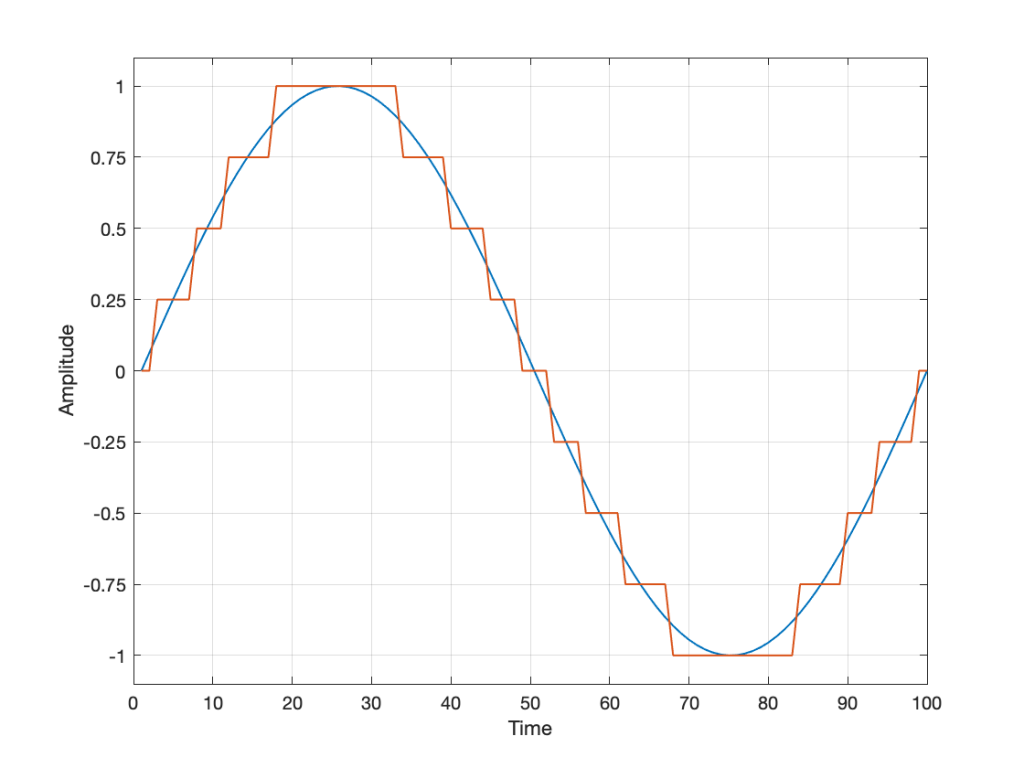Fig 2. The original signal is shown as the blue line. The quantized version of it is shown as the red line.

Of course, I’m leaving out a lot of important details here like anti-aliasing filtering and dither (I said that we were going to be dumb…) but those things don’t matter for this discussion.

So far so good. However, we have to be a bit more specific: an LPCM system encodes the values using binary representations of the values. So, a quantisation value of “0.25”, as shown above isn’t helpful. So, let’s make a “baby” LPCM system with only 3 bits (meaning that we have three Binary digITs available to represent our values).

To start, let’s count using a 3-bit system:

and that’s as far as we can go before needing 4 bits. However, for now, that’s enough.

Take a look at our signal. It ranges from -1 to 1 and 0 is in the middle. So, if we say that the “0” in our original signal is encoded as “000” in our 3-bit system, then we just count upwards from there as follows: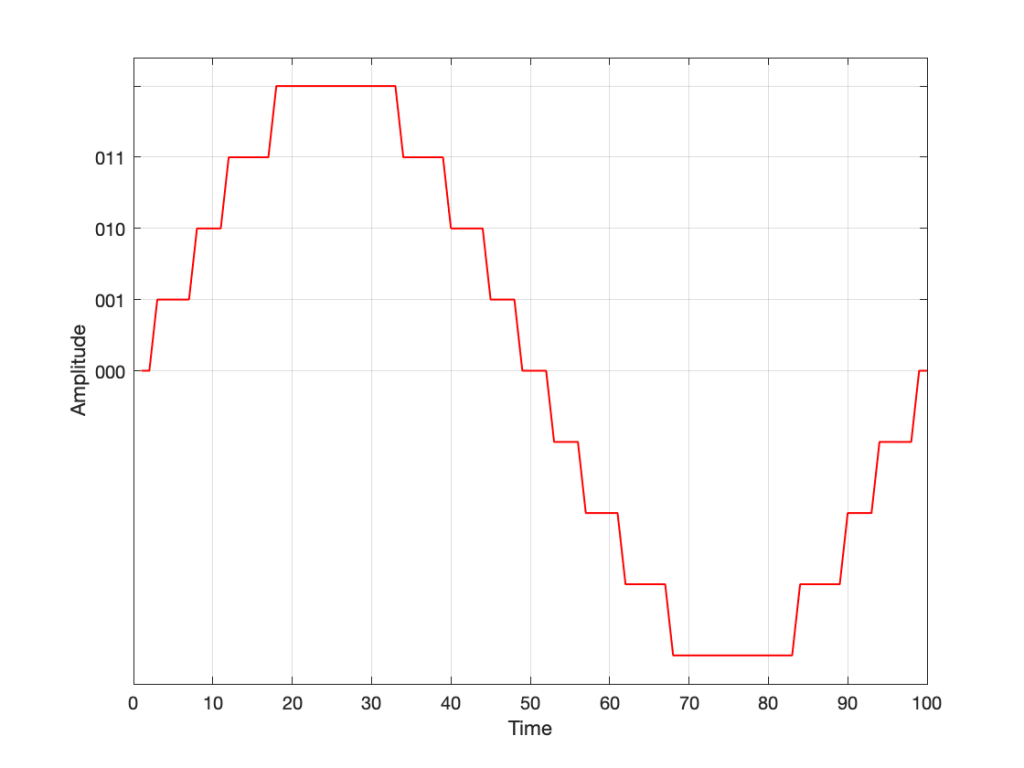Fig 3. Starting at 000 for the “0” value, and counting upwards into the positive values.

Now what? Well, let’s look at this a little differently. If we were to divide a circle into the same number of quantisation values, make the “12:00” position = 000, and count clockwise, it would look like this:

The question now is “how do we number the negative values?” but the answer is already in the circle shown above… If I make it a little more obvious, then the answer is shown below.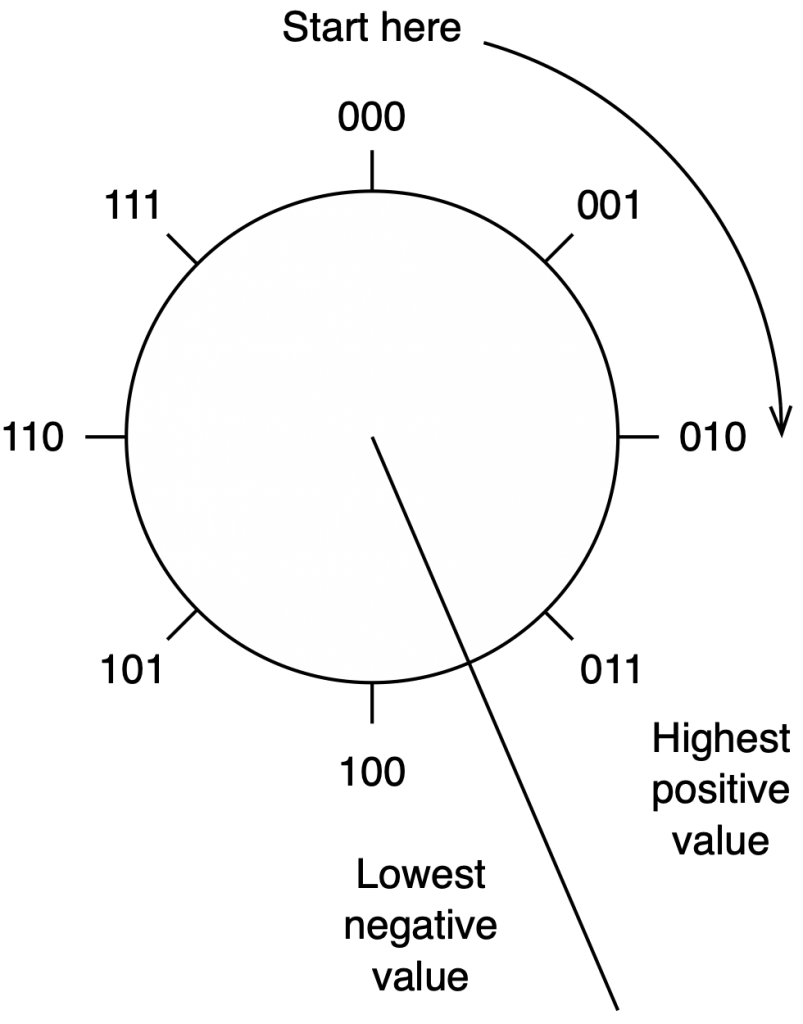Fig 5. Relating the values on the circle to the values we’ll need to represent the audio signal…

If we use the convention shown above, and represent that on the graph of our audio signal, then it looks like this:

One nice thing about this way of doing things is that you just need to look at the first digit in the binary word to know whether the value is positive or negative. A 0 means it’s positive, and a 1 means it’s negative.

However, there are two issues here that we need to sort out… The first is that, since we have an even number of values, but an odd number of quantisation steps (4 above zero, 4 below zero, and zero = 9 steps) then we had to do something asymmetrical. As you can see in the plot above, there are no numbers assigned to the top quantisation value, which actually means that it doesn’t exist.

So, if we’re still being dumb, then the result of our quantisation will either look like this: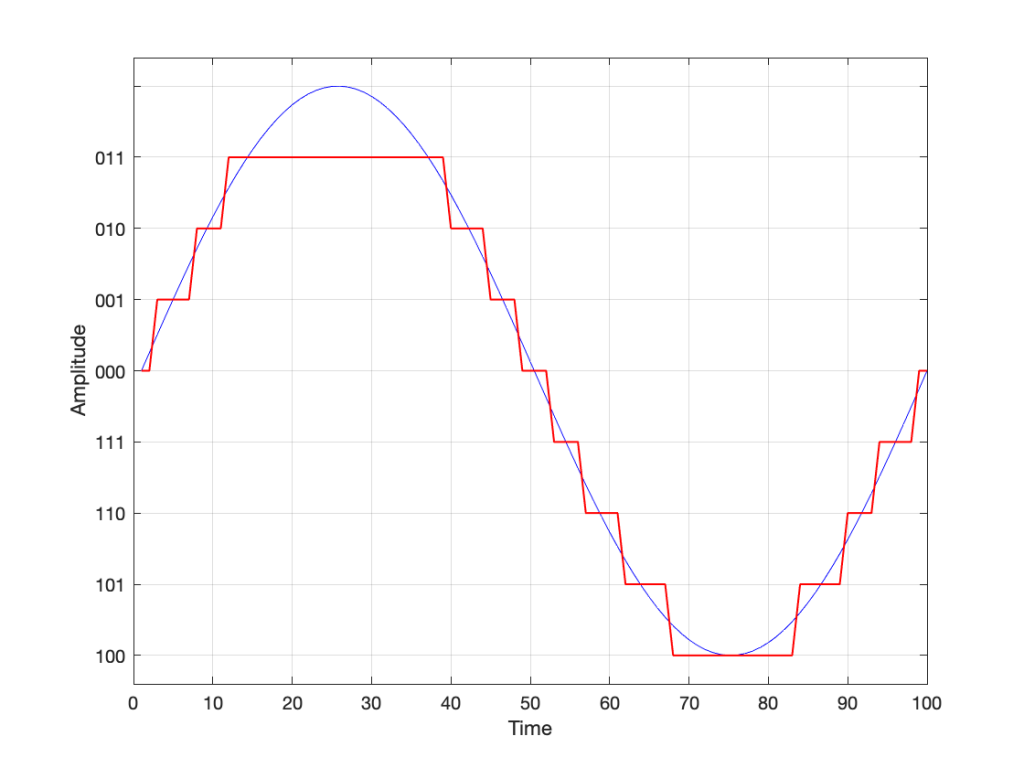Fig 7. The dumb way to deal with the asymmetrical quantisation problem. Notice that the result is asymmetrically clipped on the positive side, but not the negative.

or this: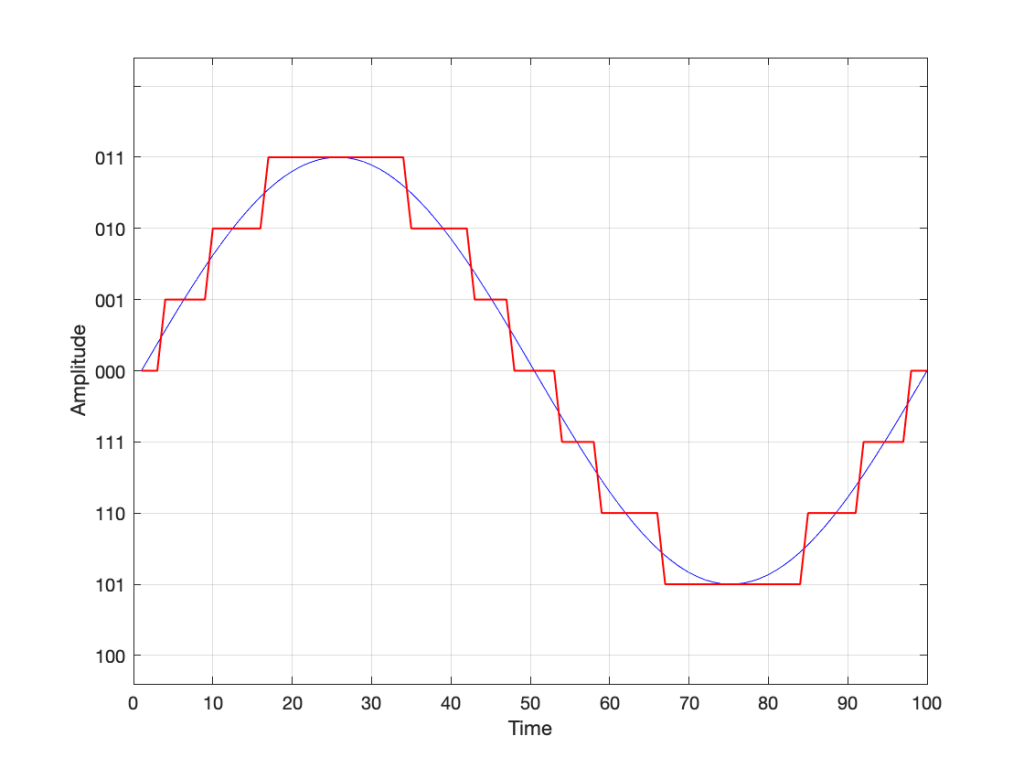Fig 8. A smarter way to deal with the asymmetrical quantisation problem. Notice that we’ll never use the bottom value.

## Wrapping up…

But what happens when you make two mistakes simultaneously? Let’s go back and look at an earlier plot.

Let’s say that you’re writing some DSP code, and you forget about the asymmetry problem, so you scale things so they’ll TRY to look like the plot above.

However, as we already know, that top quantisation value doesn’t exist – but the code will try to put something there. If you’ve forgotten about this, then the system will THINK that you want this: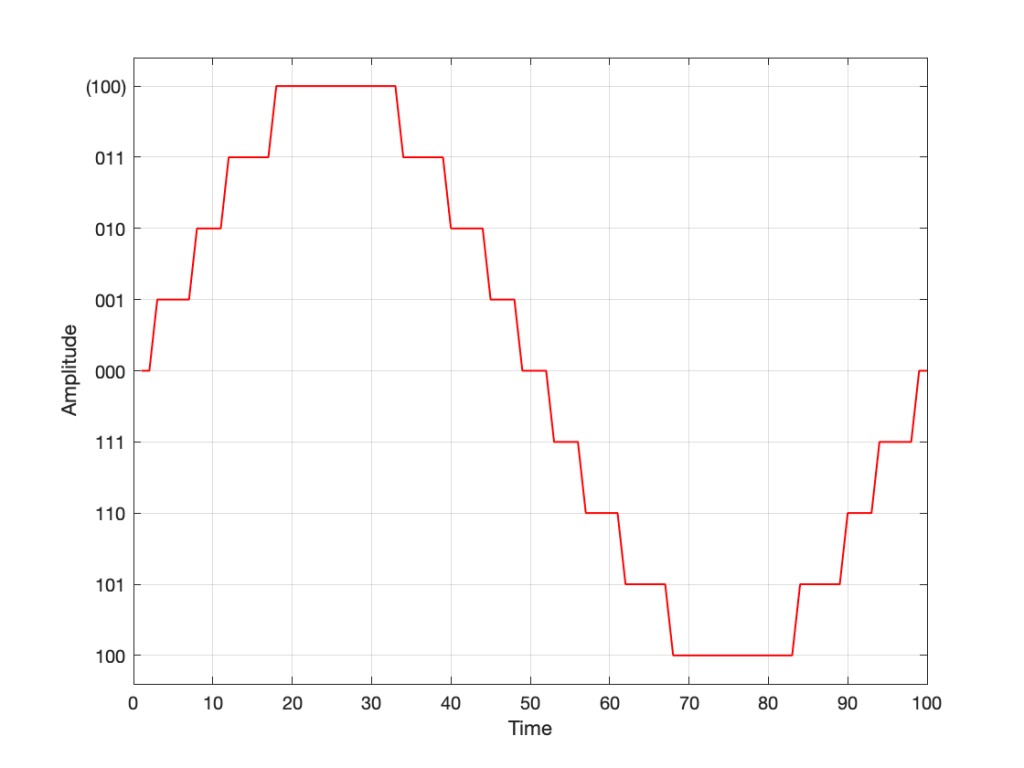Fig 10. Notice that top quantisation value. I’ve labeled it as (100) because that’s the binary number after 011 – but 100 is ACTUALLY already used for the bottom-most negative value… Bad things are about to happen.

As you can see there, your code (because you’ve forgotten to write an IF-THEN statement) will think that the top-most positive quantisation value is just the number after 011, which is 100. However, that value means something totally different… So, the result coming out will ACTUALLY look like this:

As you can see there, the signal is very different from what we think it should be.

This error is called a “wrapping” error, because the signal is “wrapped” too far around the circle shown in Figure 5, shown above. It sounds very bad – much worse than “normal” clipping (as shown in Figure 7) because of that huge nearly-instantaneous transition from maximum positive to maximum negative and back.

Of course, the wrapping can also happen in the opposite direction; a negatively-clipped signal can wrap around and show up at the top of the positive values. The reason is the same because the values are trying to go around the same circle.

As I said: this is actually the result of two problems that both have to occur in the same system:

• The signal has to be trying to get to a level that is beyond the limits of the quantisation values
• Someone forgot to write a line of code that makes sure that, when that happens, the signal is “just” clipped and not wrapped.

So, if the second of these issues is sitting there, unresolved, but the signal never exceeds the limits, then you’ll never have a problem. However, I will never need the airbags in my car, unless I have an accident. So, it’s best to remember to look after that second issue… just in case.

## P.S.

This method of encoding the quantisation values is called the “Two’s Complement” method. If you want to know more about it, read this.